## Info

Publication number
WO2009081981A1
WO2009081981A1 PCT/JP2008/073606 JP2008073606W WO2009081981A1 WO 2009081981 A1 WO2009081981 A1 WO 2009081981A1 JP 2008073606 W JP2008073606 W JP 2008073606W WO 2009081981 A1 WO2009081981 A1 WO 2009081981A1
Authority
WO
WIPO (PCT)
Prior art keywords
target
unit
correlation matrix
data
frequency
Prior art date
Application number
PCT/JP2008/073606
Other languages
French (fr)
Japanese (ja)
Inventor
Junji Kanamoto
Hiroyuki Akimoto
Original Assignee
Honda Elesys Co., Ltd.
Priority date (The priority date is an assumption and is not a legal conclusion. Google has not performed a legal analysis and makes no representation as to the accuracy of the date listed.)
Filing date
Publication date
Priority to JP2007-331567 priority Critical
Priority to JP2007331567A priority patent/JP5114187B2/en
Priority to JP2008002313A priority patent/JP5114217B2/en
Priority to JP2008-002312 priority
Priority to JP2008002312A priority patent/JP2009162688A/en
Priority to JP2008-002313 priority
Application filed by Honda Elesys Co., Ltd. filed Critical Honda Elesys Co., Ltd.
Publication of WO2009081981A1 publication Critical patent/WO2009081981A1/en

• 239000011159 matrix materials Substances 0.000 claims abstract description 496
• 230000035559 beat frequency Effects 0.000 claims abstract description 169
• 230000005540 biological transmission Effects 0.000 claims abstract description 139
• 238000000034 methods Methods 0.000 claims description 231
• 238000004364 calculation methods Methods 0.000 claims description 213
• 238000001228 spectrum Methods 0.000 claims description 161
• 230000000875 corresponding Effects 0.000 claims description 79
• 238000000354 decomposition reactions Methods 0.000 claims description 46
• 230000007274 generation of a signal involved in cell-cell signaling Effects 0.000 claims description 25
• 238000010606 normalization Methods 0.000 claims description 12
• 230000001276 controlling effects Effects 0.000 claims description 2
• 230000001702 transmitter Effects 0.000 claims 2
• 239000000203 mixtures Substances 0.000 description 68
• 230000001174 ascending Effects 0.000 description 50
• 230000000630 rising Effects 0.000 description 43
• 238000010586 diagrams Methods 0.000 description 42
• 238000006243 chemical reactions Methods 0.000 description 18
• 238000004891 communication Methods 0.000 description 12
• 230000000694 effects Effects 0.000 description 11
• 230000000051 modifying Effects 0.000 description 9
• 238000005070 sampling Methods 0.000 description 9
• 230000002596 correlated Effects 0.000 description 7
• 241000212893 Chelon labrosus Species 0.000 description 6
• 230000001629 suppression Effects 0.000 description 6
• 230000001131 transforming Effects 0.000 description 6
• 280000819004 Esprit companies 0.000 description 5
• 239000000562 conjugates Substances 0.000 description 4
• 230000004044 response Effects 0.000 description 4
• 238000000926 separation method Methods 0.000 description 4
• 230000002093 peripheral Effects 0.000 description 3
• 238000007476 Maximum Likelihood Methods 0.000 description 2
• 230000003044 adaptive Effects 0.000 description 2
• 230000001419 dependent Effects 0.000 description 2
• 230000001809 detectable Effects 0.000 description 2
• 238000005516 engineering processes Methods 0.000 description 2
• 239000000284 extracts Substances 0.000 description 2
• 238000001914 filtration Methods 0.000 description 2
• 280000456968 Formi companies 0.000 description 1
• 238000004458 analytical methods Methods 0.000 description 1
• 230000003247 decreasing Effects 0.000 description 1
• 239000006185 dispersions Substances 0.000 description 1
• 230000004048 modification Effects 0.000 description 1
• 238000006011 modification reactions Methods 0.000 description 1

## Images

•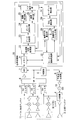•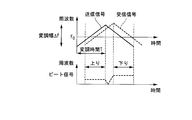•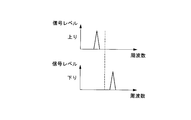•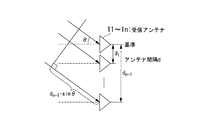•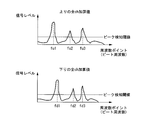•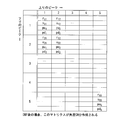••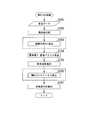•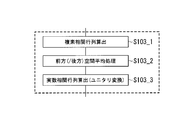••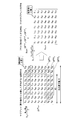•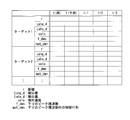•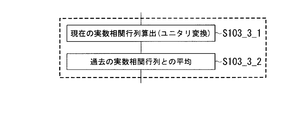•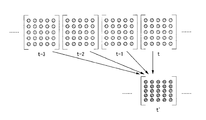•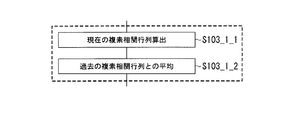•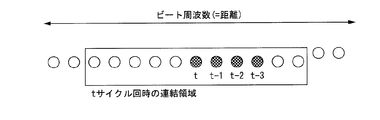•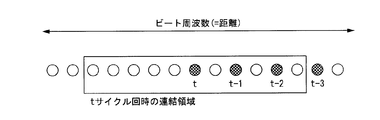•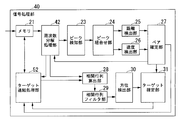•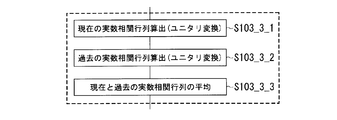••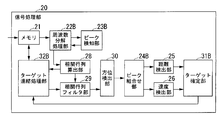•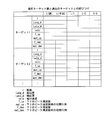•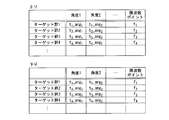•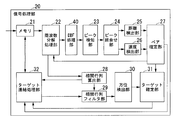•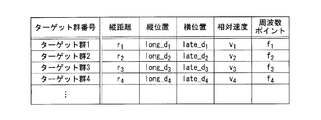••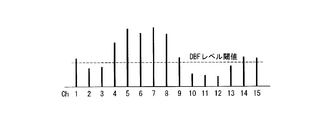•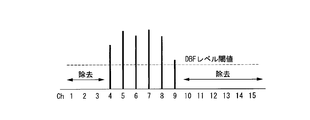•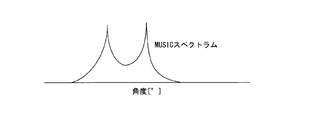•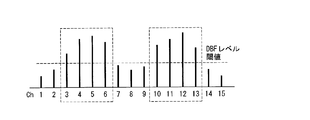••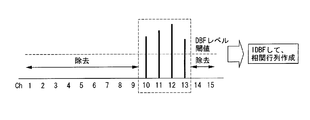•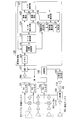•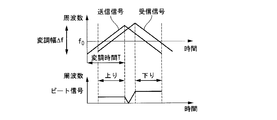•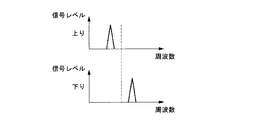•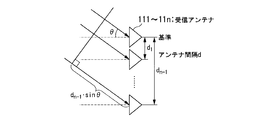•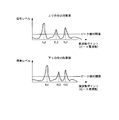•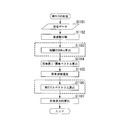•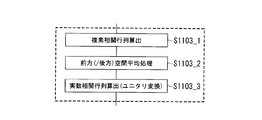•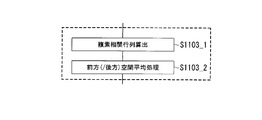•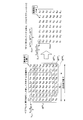•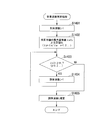•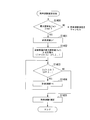•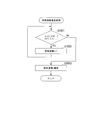•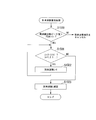•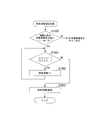•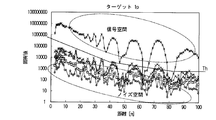•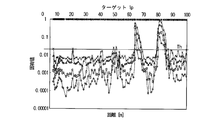•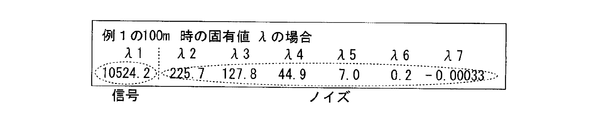•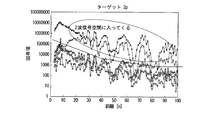•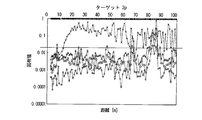•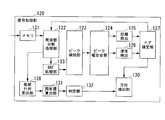•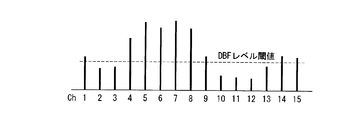•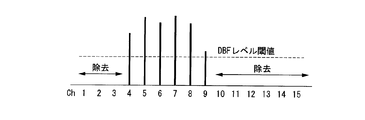•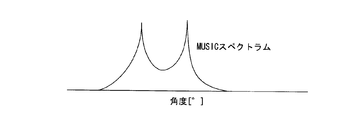•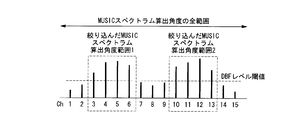•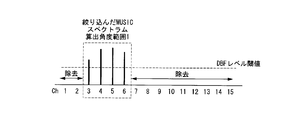•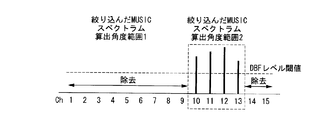•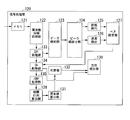•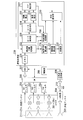•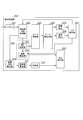•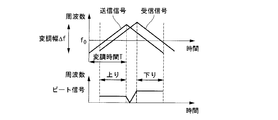•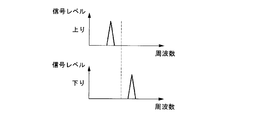•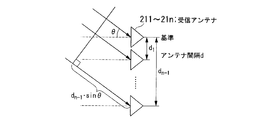•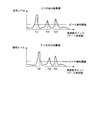•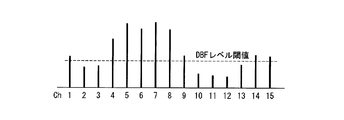•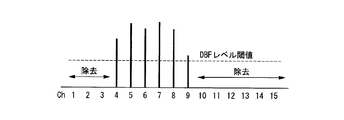•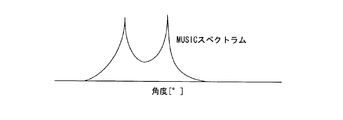•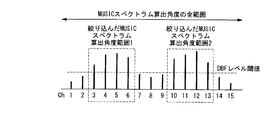•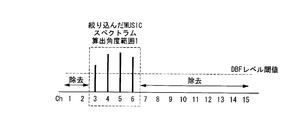•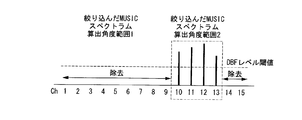•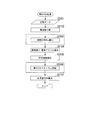•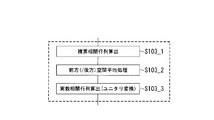•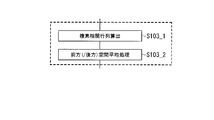•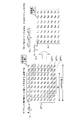•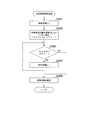•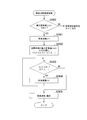•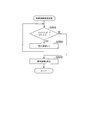•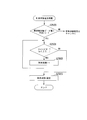•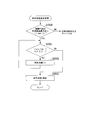•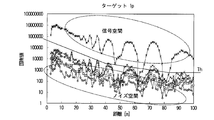•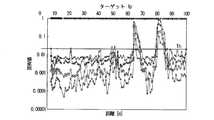•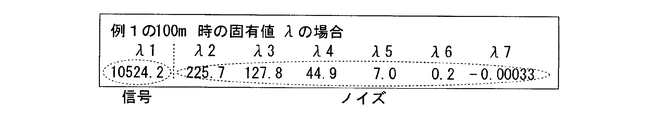•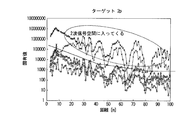•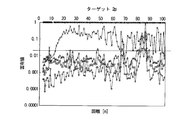•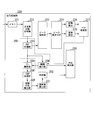•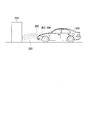## Classifications

• GPHYSICS
• G01MEASURING; TESTING
• G01S13/00Systems using the reflection or reradiation of radio waves, e.g. radar systems; Analogous systems using reflection or reradiation of waves whose nature or wavelength is irrelevant or unspecified
• G01S13/02Systems using reflection of radio waves, e.g. primary radar systems; Analogous systems
• G01S13/06Systems determining position data of a target
• G01S13/42Simultaneous measurement of distance and other co-ordinates
• GPHYSICS
• G01MEASURING; TESTING
• G01S13/00Systems using the reflection or reradiation of radio waves, e.g. radar systems; Analogous systems using reflection or reradiation of waves whose nature or wavelength is irrelevant or unspecified
• G01S13/02Systems using reflection of radio waves, e.g. primary radar systems; Analogous systems
• G01S13/04Systems determining the presence of a target
• GPHYSICS
• G01MEASURING; TESTING
• G01S13/00Systems using the reflection or reradiation of radio waves, e.g. radar systems; Analogous systems using reflection or reradiation of waves whose nature or wavelength is irrelevant or unspecified
• G01S13/02Systems using reflection of radio waves, e.g. primary radar systems; Analogous systems
• G01S13/06Systems determining position data of a target
• G01S13/08Systems for measuring distance only
• G01S13/32Systems for measuring distance only using transmission of continuous unmodulated waves, amplitude-, frequency- or phase-modulated waves
• G01S13/34Systems for measuring distance only using transmission of continuous unmodulated waves, amplitude-, frequency- or phase-modulated waves using transmission of frequency-modulated waves and the received signal, or a signal derived therefrom, being heterodyned with a locally-generated signal related to the contemporaneous transmitted signal to give a beat-frequency signal
• G01S13/345Systems for measuring distance only using transmission of continuous unmodulated waves, amplitude-, frequency- or phase-modulated waves using transmission of frequency-modulated waves and the received signal, or a signal derived therefrom, being heterodyned with a locally-generated signal related to the contemporaneous transmitted signal to give a beat-frequency signal using triangular modulation
• GPHYSICS
• G01MEASURING; TESTING
• G01S13/00Systems using the reflection or reradiation of radio waves, e.g. radar systems; Analogous systems using reflection or reradiation of waves whose nature or wavelength is irrelevant or unspecified
• G01S13/02Systems using reflection of radio waves, e.g. primary radar systems; Analogous systems
• G01S13/50Systems of measurement based on relative movement of target
• G01S13/58Velocity or trajectory determination systems; Sense-of-movement determination systems
• G01S13/583Velocity or trajectory determination systems; Sense-of-movement determination systems using transmission of continuous unmodulated waves, amplitude-, frequency-, or phase-modulated waves and based upon the Doppler effect resulting from movement of targets
• G01S13/584Velocity or trajectory determination systems; Sense-of-movement determination systems using transmission of continuous unmodulated waves, amplitude-, frequency-, or phase-modulated waves and based upon the Doppler effect resulting from movement of targets adapted for simultaneous range and velocity measurements
• GPHYSICS
• G01MEASURING; TESTING
• G01S13/00Systems using the reflection or reradiation of radio waves, e.g. radar systems; Analogous systems using reflection or reradiation of waves whose nature or wavelength is irrelevant or unspecified
• G01S13/93Radar or analogous systems specially adapted for specific applications for anti-collision purposes
• G01S13/931Radar or analogous systems specially adapted for specific applications for anti-collision purposes of land vehicles
• GPHYSICS
• G01MEASURING; TESTING
• G01S3/00Direction-finders for determining the direction from which infrasonic, sonic, ultrasonic, or electromagnetic waves, or particle emission, not having a directional significance, are being received
• G01S3/02Direction-finders for determining the direction from which infrasonic, sonic, ultrasonic, or electromagnetic waves, or particle emission, not having a directional significance, are being received using radio waves
• G01S3/74Multi-channel systems specially adapted for direction-finding, i.e. having a single antenna system capable of giving simultaneous indications of the directions of different signals
• GPHYSICS
• G01MEASURING; TESTING
• G01S13/00Systems using the reflection or reradiation of radio waves, e.g. radar systems; Analogous systems using reflection or reradiation of waves whose nature or wavelength is irrelevant or unspecified
• G01S13/93Radar or analogous systems specially adapted for specific applications for anti-collision purposes
• G01S13/931Radar or analogous systems specially adapted for specific applications for anti-collision purposes of land vehicles
• G01S2013/9327Sensor installation details
• G01S2013/93271Sensor installation details in the front of the vehicles

## Abstract

An electronic scanning radar apparatus equipped in a moving body is characterized by comprising a transmitting unit for transmitting a transmission wave, a receiving unit for receiving a reflected wave of the transmission wave from a target, a beat signal generating unit for generating a beat signal from the transmission wave and the reflected wave, a frequency decomposing unit for calculating complex number data, a target detecting unit for detecting a target if any, a correlation matrix calculating unit for calculating a correlation matrix from the complex number data on a detected beat frequency, a target connecting unit for associating the target in the present detection cycle with the same in the past detection cycle, a correlation matrix filter unit for generating an average correlation matrix defined by weight-averaging of the correlation matrix of the target in the present detection cycle and the correlation matrix of the same in the associated past detection cycle, and a direction detecting unit for calculating the direction in which the received wave has arrived from the average correlation matrix.

## Description

The present invention relates to an on-vehicle electronic scanning radar apparatus, a reception wave direction estimation method, and an arrival wave direction estimation program used therefor, which detect a target using a reflected wave from a target with respect to a radiated transmission wave. .
The present application is Japanese Patent Application No. 2007-331567, filed on Dec. 25, 2007, Japanese Patent Application No. 2008-002312, filed on Jan. 9, 2008, on Jan. 9, 2008. The priority is claimed based on Japanese Patent Application No. 2008-002313 filed, the contents of which are incorporated herein.

Conventionally, as an in-vehicle radar, an electronic scanning type radar using a system such as a FMCW (Frequency Modulated Continuous Wave) radar, a multi-frequency CW (Continuous Wave) radar, a pulse radar, or the like is used.
In each of the above radars, an array antenna arrival wave direction estimation method is used as a technique for detecting the direction of an incoming wave (or a received wave) from a target.
This arrival wave direction estimation method includes a beam scanning method such as a beam former method and a Capon method, a linear prediction method such as a maximum entropy method (MEM) method, a minimum norm method, a MUSIC (Multiple Signal Classification) method, and an ESPRIT method. There is a null operation method called a super-resolution (high accuracy) algorithm such as (Estimation of Signal Parameters via Rotational Invariance Techniques) method (for example, see Non-Patent Documents 1 and 2).

In addition, the direction of arrival wave used for in-vehicle radar can be estimated only by a digital beam forming (DBF) method (for example, refer to Patent Document 1) of beamformer method, or the detection accuracy of the direction of arrival of a received wave ( In recent years, in order to improve the resolution of the target, a method combining DBF and the maximum entropy method (for example, see Patent Documents 2 and 3) has been performed.
Furthermore, in order to apply super-resolution algorithms such as MUSIC for in-vehicle radar, the estimation algorithm is simplified because it uses a microprocessor for in-vehicle use that has a lower arithmetic processing function than a normal personal computer. Such a method has been developed (for example, Patent Documents 4, 5, 6, and 7).

The super resolution algorithm such as MUSIC creates a correlation matrix from the received wave data of each array antenna, calculates an eigenvalue from the correlation matrix, and detects the arrival direction of the received wave. Here, eigenvalue calculation (calculation) is to obtain eigenvalues and eigenvectors.
At this time, the super-resolution algorithm improves the accuracy of detection of the direction of arrival as the noise component of the correlation matrix is removed. Therefore, the super-resolution algorithm creates a correlation matrix by ensemble averaging of received data.
For example, the FMCW radar acquires the number of samples of the received beat signal data set (time series data in a certain time interval that can be converted into frequency domain data) as much as possible, and uses an averaged correlation matrix. The number of samples is called the number of snapshots (see, for example, Non-Patent Documents 1 and 2).

However, in the in-vehicle radar, since the distance and relative speed with respect to the target constantly fluctuate, even if the number of snapshots is increased vaguely, the detection accuracy of the arrival direction of the received wave is not always improved.
Conversely, in order to earn the number of snapshots during the control cycle (or detection cycle, eg, 100 ms) for detecting the target, the number of times the array antenna is multiplied by the number of snapshots at the same time as the frequency resolution processing for the received signal. There is a limit to the number of
In order to improve target detection accuracy without increasing the number of snapshots, in Patent Document 4, for example, a correlation matrix for each beat frequency at the previous (or last) control cycle is stored, and the current There is described a method of performing weighted addition (weighted average) between the correlation matrix of the beat frequency where the target exists in the control cycle and the previous (or the previous) correlation matrix of the same beat frequency. Further, in Patent Document 4, the weighted correlation matrix is stored for each beat frequency, and the weighted correlation of the same beat frequency as the correlation matrix of the beat frequency where the target exists in the current control cycle is stored. A method for further weighted addition to a matrix is also described.

Moreover, in the said patent document 5, it produces with the frequency which shows a peak value, and its vicinity (for example, +/- 2 frequency resolution part) from the same peak waveform in which the target exists in beat frequency. A method is described in which each correlation matrix is averaged to increase the number of snapshots.
In this Patent Document 5, it is described that an average of correlation matrices between frequency domains is further averaged by using a past correlation matrix.
Patent Document 6 describes estimation of the direction of arrival of a received wave by combining a roadside shape estimation method and a method of averaging current and past correlation matrices.

Further, Patent Document 7 describes a method for determining the weighting coefficient (or forgetting coefficient: a constant indicating the degree of forgetting) in real time in order to average the current and past correlation matrices.

Conventionally, as an in-vehicle radar, an electronic scanning type radar using a system such as a FMCW (Frequency Modulated Continuous Wave) radar, a multi-frequency CW (Continuous Wave) radar, or a pulse radar is used.
In each of the above radars, an arrival direction estimation method of an array antenna is used as a technique for detecting the direction of an incoming wave (or a received wave) that is a reflected wave from a target with respect to a transmission wave.
This arrival wave direction estimation method includes a beam scanning method such as a beam former method and a Capon method, a linear prediction method such as a maximum entropy method (MEM) method, a minimum norm method, a MUSIC (Multiple Signal Classification) method, and an ESPRIT method. There is a null operation method called a super-resolution (high accuracy) algorithm such as an (Estimation of Signal Parameters via Rotational Invariance Techniques) method (for example, see Non-Patent Documents 1 and 2).

In addition, the direction of arrival wave used for in-vehicle radar can be estimated only by a digital beam forming (DBF) method (for example, refer to Patent Document 1) of beamformer method, or the detection accuracy of the direction of arrival of a received wave ( In recent years, in order to improve the resolution of the target, a method combining DBF and the maximum entropy method (for example, see Patent Documents 2 and 3) has been performed.
Furthermore, compared with a normal personal computer, such as a method in which a super-resolution algorithm such as MUSIC is applied to in-vehicle radar for the purpose of simplifying processing (for example, see Patent Documents 4 and 8), an arithmetic processing function A method that can be applied to in-vehicle use is being developed.

In a super-resolution algorithm such as MUSIC, in order to improve the accuracy of direction estimation, it is desirable to estimate the direction of the incoming wave after estimating the number of incoming waves.
Non-Patent Documents 1 and 2 introduce AIC (Akaike Information Criteria), MDL (Minimum Description Length), and the like as methods for estimating the number of incoming waves based on the maximum likelihood method in statistical processing.
However, in the estimation methods of Non-Patent Documents 1 and 2 described above, since it is necessary to collect and evaluate a large number of data, it is suitable for use as an on-vehicle radar in which the relative distance and the relative speed with the target are fast. Not.

Patent Document 8 describes a method for estimating the number of incoming waves necessary for calculating a MUSIC spectrum with a light calculation load. That is, Patent Document 8 describes a technique that applies a threshold method that estimates signal space and noise space by performing eigenvalue calculation and then distinguishing between signal space and noise space.
In this case, since the radar reception intensity decreases as the measurement distance increases, the above-described method stores and sets a threshold value for each relative distance to the target, and this threshold value and an eigenvalue (equivalent to the reception intensity). Is used to estimate the number of incoming waves.
Although not intended for in-vehicle use, there is a method in which the eigenvalue is normalized by one of the diagonal component values of the original covariance matrix (that is, the correlation matrix) and then distinguished by one threshold value. (For example, refer to Patent Document 9).

In addition, as an accurate method for estimating the number of incoming waves, spectrum calculation is always performed assuming that the number of incoming waves is the maximum number that can be received, and in the subsequent power calculation, unnecessary peak values are removed and the final estimated number of incoming waves is obtained. (Patent Document 10).

Conventionally, as an in-vehicle radar, an electronic scanning type radar using a system such as a FMCW (Frequency Modulated Continuous Wave) radar, a multi-frequency CW (Continuous Wave) radar, or a pulse radar is used.
In each of the above radars, an arrival direction estimation method of an array antenna is used as a technique for detecting the direction of an incoming wave (or a received wave) that is a reflected wave from a target with respect to a transmission wave.
This arrival wave direction estimation method includes a beam scanning method such as a beam former method and a Capon method, a linear prediction method such as a maximum entropy method (MEM) method, a minimum norm method, a MUSIC (Multiple Signal Classification) method, and an ESPRIT method. There is a null operation method called a super-resolution (high accuracy) algorithm such as an (Estimation of Signal Parameters via Rotational Invariance Techniques) method (for example, see Non-Patent Documents 1 and 2).

In addition, the direction of arrival wave used for in-vehicle radar can be estimated only by a digital beam forming (DBF) method (for example, refer to Patent Document 1) of beamformer method, or the detection accuracy of the direction of arrival of a received wave ( In recent years, in order to improve the resolution of the target, a method combining DBF and the maximum entropy method (for example, see Patent Documents 2 and 3) has been performed.
Furthermore, compared with a normal personal computer, such as a method in which a super-resolution algorithm such as MUSIC is applied to in-vehicle radar for the purpose of simplifying processing (for example, see Patent Documents 4 and 8), an arithmetic processing function A method that can be applied to in-vehicle use is being developed.

In a super-resolution algorithm such as MUSIC, in order to improve the accuracy of direction estimation, it is desirable to estimate the direction of the incoming wave after estimating the number of incoming waves.
Non-Patent Documents 1 and 2 introduce AIC (Akaike Information Criteria), MDL (Minimum Description Length), and the like as methods for estimating the number of incoming waves based on the maximum likelihood method in statistical processing.
However, the estimation methods of Non-Patent Documents 1 and 2 described above need to collect a large number of data and perform dispersion evaluation, and thus are suitable for use as an on-vehicle radar in which the relative distance to the target and the relative speed vary quickly. Absent.

Patent Document 8 describes a method for estimating the number of incoming waves necessary for calculating a MUSIC spectrum with a light calculation load. That is, Patent Document 8 describes a technique that applies a threshold method that estimates signal space and noise space by performing eigenvalue calculation and then distinguishing between signal space and noise space.
In this case, since the radar reception intensity decreases as the measurement distance increases, the above-described method stores and sets a threshold value for each relative distance to the target, and this threshold value and an eigenvalue (proportional to the reception intensity). Is used to estimate the number of incoming waves.
Although not intended for in-vehicle use, there is a method in which the eigenvalue is normalized by one of the diagonal component values of the original covariance matrix (that is, the correlation matrix) and then distinguished by one threshold value. (For example, refer to Patent Document 9).
Nobuyoshi Kikuma, Adaptive signal processing with array antenna, Science and Technology Publishers, 1998 Nobuyoshi Kikuma, Adaptive Antenna Technology, Ohmsha, 2003 JP 2000-284044 A JP 2006-275840 A JP 2006-308542 A JP 2007-040806 A JP 2006-145251 A JP 2006-242695 A JP 2006-284182 A JP 2006-047282 A JP 2006-153579 A JP 2000-121716 A

However, in Patent Document 4, it is necessary to frequency-resolve the past correlation matrix into all beat frequencies in the conventional correlation matrix averaging process. For example, when Fourier transform is performed at 256 discrete times, it is necessary to store 128 discrete frequencies. For this reason, the conventional averaging process of the correlation matrix has a problem that a large-capacity memory corresponding to “the number of data of the correlation matrix × the total beat frequency” is required. In addition, since the beat frequency of the past correlation matrix is the same as the beat frequency of the current target, when the distance to the target is tracking at a constant distance, the detection data is averaged. It becomes. On the other hand, when the distance to the target fluctuates, the target may not exist at the previous frequency, and there is a concern that the data for detection will deteriorate.

Further, in Patent Document 5, as a conventional correlation matrix averaging method, the correlation matrix is always averaged between neighboring beat frequencies, and then averaged with the past correlation matrix.
For this reason, the conventional correlation matrix averaging method is based on the premise that the signal intensity as the presence level of the target is shown in the frequency band including the nearby beat frequency, and the discrete frequency resolution of the beat frequency is very fine. Only applicable to cases. Further, since the above-described method is always performed in combination with the averaging of correlation matrices between neighboring beat frequencies, the arrival direction of the received wave is not detected using the past correlation matrix alone.
Furthermore, Patent Document 6 is based on the premise that the roadside shape estimation method is performed in the conventional correlation matrix averaging method as well as Patent Document 5, and the received wave using the past correlation matrix alone is used. The direction of arrival is not detected, and the processing is complicated.

The present invention has been made in view of such circumstances, and an electronic scanning radar apparatus and a reception device that detect the arrival direction of a received wave with high accuracy by simple calculation using a past correlation matrix alone. One of the purposes is to provide a wave direction estimation program.

However, the arrival wave estimation method according to Patent Document 8 needs to store threshold values for all distances in target detection, and needs to secure a necessary memory capacity (form a threshold table map in a ROM or the like). In addition, this incoming wave estimation method has a problem that it is troublesome to create a programming for comparing a threshold value with a threshold value for each distance.
In addition, since the threshold value is an absolute amount, it cannot cope with a state in which the eigenvalue fluctuates up and down due to RCS (Radar Cross Section) of the target or clutter and noise that are reflections from unnecessary objects, There is concern about the lack of robustness.

In addition, the arrival wave estimation method according to Patent Document 9 normalizes the calculated eigenvalue by one of the diagonal components of the original covariance matrix, but always estimates the number of arrival waves. There is a possibility of calculating an incorrect estimated number when the wave is weak. Here, “weak” refers to a signal level that is far below the level at which the eigenvalue fluctuates up and down due to the RCS, clutter, and noise of the target.
In particular, in in-vehicle radar applications, there is a phenomenon called multipath (a kind of clutter) from the road surface, and a distance region in which the received wave has the above weak signal level is always generated.
For this reason, the estimation of the number of incoming waves in the distance region where the signal level is weak outputs an incorrect result.

Further, although the arrival wave estimation method according to Patent Document 10 does not require the process of estimating the number of arrival waves before the MUSIC spectrum calculation, it is necessary to always execute the power calculation with the inverse matrix calculation after the spectrum calculation. There is a problem that the calculation load becomes heavy.

The present invention has been made in view of such circumstances, and has high robustness and high accuracy when estimating an incoming wave direction by estimating an incoming wave using eigenvalue decomposition such as the MUSIC method and the Esprit method. An object of the present invention is to provide an electronic scanning radar apparatus that estimates the number of incoming waves, a reception wave direction estimation method, and a reception wave direction estimation program.

However, in the MUSIC method, as shown below, the accuracy of direction-of-arrival estimation changes due to the variable step Δθ of θ in the arrival angle evaluation function.
That is, when the variable step Δθ is increased, the amount of calculation over the entire range in which θ is varied decreases, but the peak direction of the arrival direction evaluation function cannot be accurately detected, and the accuracy deteriorates.
On the other hand, by reducing the variable step Δθ, it is possible to accurately detect the peak direction of the arrival direction evaluation function, but the MUSIC method has a disadvantage that the amount of calculation over the entire range in which θ is varied increases. ing.

The present invention has been made in view of such circumstances, and when performing arrival wave direction detection using eigenexpansion such as the MUSIC method or the minimum norm method, the variable step is reduced and the amount of calculation for angle detection is reduced. An object of the present invention is to provide an electronic scanning millimeter-wave radar device and a reception wave direction estimation program that can be reduced.

(1) As one aspect of the present invention, the following configuration is provided: an electronic scanning radar device mounted on a moving body: a transmission unit that transmits a transmission wave; and a reflected wave from a target of the transmission wave is received A reception unit composed of a plurality of antennas; a beat signal generation unit that generates a beat signal from the transmission wave and the reflected wave; and complex data obtained by frequency-decomposing the beat signal into a predetermined number of beat frequencies A frequency resolving processing unit that calculates a peak value from the intensity value of the beat frequency to detect the presence of the target; complex data of a detected beat frequency at which the target is detected for each antenna A correlation matrix calculation unit for calculating a correlation matrix from each of the targets; the target in the current detection cycle and the past detection cycle; A target concatenation processing unit that correlates with separation and relative velocity; a correlation matrix that generates an average correlation matrix that weights and averages the correlation matrix of the target of the current detection cycle and the correlation matrix of the target of the associated past detection cycle An electronic scanning radar apparatus comprising: a filter unit; and an azimuth detection unit that calculates an arrival direction of the reflected wave from the average correlation matrix.

(2) The electronic scanning radar apparatus may be configured as follows: When the target connection processing unit associates the targets in the current and past detection cycles, the detection beat of the current detection cycle Depending on whether the distance and relative speed obtained by the frequency are included in the distance range and the relative speed range that are predicted and set in advance by the distance and relative speed obtained by the past detection cycle, respectively, between the targets. Detects whether there is an association.

(3) The electronic scanning radar apparatus may be configured as follows: distance, relative velocity, and correlation matrix respectively corresponding to one or more past cycles of the associated target are stored. A storage unit that performs weighted averaging of a correlation matrix between a target in a current detection cycle and a target in a plurality of time-series past detection cycles associated with the current target. An average correlation matrix is generated, and the current target distance, relative velocity, and correlation matrix are associated with the associated past target distance, relative velocity, and correlation matrix, and stored in the storage unit.

(4) The electronic scanning radar apparatus described above may be configured as follows: Complex number data of a detected beat frequency corresponding to the associated target is stored corresponding to one or a plurality of cycles. A storage unit; when a past detection cycle target associated with a current detection cycle target is detected, the correlation matrix calculation unit calculates the correlation matrix from complex number data of the past detection cycle. The target concatenation processor generates a weighted average correlation matrix between a target in a current detection cycle and a past target associated with the current target, and the associated current target Complex data of distance, relative speed and detected beat frequency is stored in the associated past detection cycle. Distance of the target in Le, in association with the relative velocity and complex data stored.

(5) The electronic scanning radar apparatus described above may be configured as follows: digital that performs digital beamforming in the channel direction and detects the presence and orientation of the target based on the complex data for each antenna. A beam forming unit; detecting the azimuth of the target by the digital beam forming from the beat frequency in the current detection cycle, and associating the target in the detection cycle with the present and the past by the distance, relative velocity, and azimuth. .

(6) The electronic scanning radar apparatus described above may be configured as follows: digital that performs digital beamforming in the channel direction and detects the presence and orientation of the target based on the complex data for each antenna. It further has a beam forming unit, detects the direction of the target by the digital beam forming from the beat frequency in the current detection cycle, and associates the target in the detection cycle with the current and the past based on the distance, relative speed, and direction. .

(7) The electronic scanning radar apparatus described above may be configured as follows: the digital beam forming unit indicates the intensity of the spectrum for each angle channel by performing digital beam forming using the complex number data. When spatial complex number data is calculated and the spectrum intensity of the adjacent angle channel exceeds the preset digital beamforming threshold in the range of the preset angle channel number, the digital beamforming detection target exists in this angle channel A channel deleting unit that detects and replaces the spectrum intensity of the angle channel in which the presence of the digital beamforming detection target is not detected with “0” and outputs the new spatial complex data; and reverses the new spatial complex data By digital beamforming, and the inverse digital beam forming unit for generating a restored complex number data; further wherein the correlation matrix calculation section calculates a correlation matrix from the restored complex number data.

(8) The electronic scanning radar apparatus described above may be configured as follows: the digital beam forming unit indicates the intensity of the spectrum for each angle channel by performing digital beam forming using the complex number data. When spatial complex number data is calculated and the spectrum intensity of the adjacent angle channel exceeds the preset digital beamforming threshold in the range of the preset angle channel number, the digital beamforming detection target exists in this angle channel A channel deleting unit that detects and replaces the spectrum intensity of the angle channel in which the presence of the digital beamforming detection target is not detected with “0” and outputs the new spatial complex data; and reverses the new spatial complex data By digital beamforming, and the inverse digital beam forming unit for generating a restored complex number data; further wherein the correlation matrix calculation section calculates a correlation matrix from the restored complex number data.

(9) The electronic scanning radar apparatus may be configured as follows: when the channel deletion unit detects a plurality of digital beamforming detection targets, an angle corresponding to each of the digital beamforming detection targets By dividing the spectrum for each channel range, spatial complex number data is generated for each digital beamforming detection target, and the inverse digital beamforming unit performs inverse digital beamforming for the spatial complex number data for each digital beamforming detection target. Thus, the reproduction complex number data for each digital beam forming detection target is generated, and the correlation matrix calculation unit calculates the digital beam from the reproduction complex number data for each digital beam forming detection target. Calculating a correlation matrix for each Omingu detection target.

(10) The electronic scanning radar apparatus may be configured as follows: when the channel deletion unit detects a plurality of digital beamforming detection targets, an angle corresponding to each digital beamforming detection target Spatial complex number data for each digital beamforming detection target is generated by dividing the spectrum for each channel range; and the inverse digital beamforming unit performs inverse digital beamforming for the spatial complex number data for each digital beamforming detection target. Thus, the reproduction complex number data for each digital beam forming detection target is generated; and the correlation matrix calculating unit calculates the digital complex number from the reproduction complex number data for each digital beam forming detection target. Calculating a correlation matrix for each forming detection target.

(11) The electronic scanning radar apparatus described above may be configured as follows: the correlation matrix filter unit corresponds to the relative speed, and a weighting coefficient for weighted averaging is changed for each target. Let

(12) The electronic scanning radar apparatus described above may be configured as follows: The lateral positional change amount of the target obtained from the past and present azimuth and distance exceeds a preset range. In this case, the correlation matrix filter unit changes the weighting coefficient for weighted averaging for each target.

(13) The electronic scanning radar apparatus may be configured as follows: The number of past cycles used when the target connection processing unit averages is changed in accordance with the relative speed.

(14) As one aspect of the present invention, the following method is provided: a method of estimating a received wave direction by an electronic scanning radar device mounted on a moving body: a transmission process of transmitting a transmission wave from a transmission unit; A reception process in which a reception unit including a plurality of antennas receives a reflected wave by the target of the transmission wave; a beat signal generation process in which a beat signal generation unit generates a beat signal from the transmission wave and the reflection wave; and frequency decomposition A frequency resolving process in which the processing unit frequency-decomposes the beat signal into beat frequencies of a predetermined number of decompositions to calculate complex number data; a target detection unit detects a peak value from the intensity value of the beat frequency and A target detection process for detecting presence; and a complex of a detected beat frequency at which the correlation matrix calculation unit detects the target for each antenna. A correlation matrix calculation process for calculating a correlation matrix from each data; a target connection processing unit in which a target connection processing unit associates a target in a current detection cycle and a past detection cycle with a distance and a relative speed; a correlation matrix filter unit A correlation matrix filtering process for generating an average correlation matrix obtained by weighted averaging the correlation matrix of the detection cycle target and the correlation matrix of the associated past detection cycle target; A method for controlling an electronic scanning radar apparatus, comprising:

(15) As one aspect of the present invention, the following program is provided: a program for causing a computer to control the operation of estimating a received wave direction by an electronic scanning radar apparatus mounted on a moving body: a transmission unit transmits A transmission process for transmitting a wave; a reception process for receiving a reflected wave from the target of the transmission wave by a plurality of antennas; and a beat for generating a beat signal from the transmission wave and the reflected wave by a beat signal generating part A signal generation process; a frequency resolution processing process in which the frequency resolution processing unit frequency-decomposes the beat signal into beat frequencies of a preset resolution number to calculate complex number data; a target detection unit peaks from the intensity value of the beat frequency Target detection processing for detecting a value to detect the presence of a target; and a correlation matrix calculation unit for each antenna A correlation matrix calculation process for calculating a correlation matrix from each complex number data of the detected beat frequency at which the target is detected; target connection in which the target connection processing unit associates the target in the current detection cycle and the past detection cycle with the distance and relative velocity Correlation matrix filter processing in which the correlation matrix filter unit generates an average correlation matrix obtained by weighted averaging the correlation matrix of the target of the current detection cycle and the correlation matrix of the associated past detection cycle target; A received wave direction estimation program comprising: a direction detecting process in which a detection unit calculates an arrival direction of a received wave from the average correlation matrix.

(16) As one aspect of the present invention, the following configuration is provided: an electronic scanning radar apparatus mounted on a moving body: a transmission unit that transmits a transmission wave; a reflected wave from a target of the transmission wave; A receiving unit configured by a plurality of antennas that receive a certain incoming wave; a beat signal generating unit that generates a beat signal having a frequency difference between the transmitted wave and the reflected wave; and presetting the beat signal in time series A frequency decomposition processing unit that calculates complex number data by frequency decomposition into beat frequencies of the number of decompositions; a peak detection unit that detects a peak value from the intensity value of each beat frequency and detects the presence of a target; A correlation matrix calculation unit for calculating a correlation matrix from each complex number data of the detected beat frequency at which the target is detected; an eigenvalue for calculating an eigenvalue from the correlation matrix An output unit; a comparison unit that compares the normalized eigenvalue and a preset threshold value; among eigenvalues corresponding to the beat frequency at which the target is detected, the number of eigenvalues that exceed the threshold value An electronic scanning radar apparatus, comprising:

(17) The electronic scanning radar apparatus may be configured as follows: the eigenvalue calculation unit divides and normalizes all eigenvalues by the eigenvalue having the maximum value among the calculated eigenvalues. The comparison unit compares the normalized eigenvalue with the preset threshold value.

(18) The electronic scanning radar apparatus described above may be configured as follows: the eigenvalue calculation unit divides all elements of the correlation matrix by elements of the maximum value in the correlation matrix, and The eigenvalue is calculated by using the normalized correlation matrix, and the comparison unit compares the eigenvalue with the preset threshold value.

(19) The electronic scanning radar apparatus described above may be configured as follows: the determination unit compares a maximum eigenvalue threshold set in advance with a maximum eigenvalue in the obtained eigenvalue, and the maximum When the eigenvalue exceeds the maximum eigenvalue threshold, the eigenvalue is recognized as an effective reception level, and the number of incoming waves is estimated.

(20) The electronic scanning radar apparatus described above may be configured as follows: the eigenvalue calculation unit converts all elements of the correlation matrix by elements having maximum values in diagonal elements of the correlation matrix. The eigenvalue is calculated by the correlation matrix with the elements normalized, and the comparison unit compares the eigenvalue with the preset threshold value.

(21) The electronic scanning radar apparatus may be configured as follows: a peak value comparison unit that compares the peak value with a preset effective reception level; When the effective reception level is exceeded, the determination unit estimates the number of incoming waves assuming that the eigenvalue is the effective determination level.

(22) The electronic scanning radar apparatus described above may be configured as follows: a maximum correlation matrix diagonal element threshold that is determined in advance by the determination unit, and a diagonal element in the obtained correlation matrix; When the maximum value of the diagonal element exceeds the maximum correlation matrix diagonal element threshold value, the arrival wave is estimated as the eigenvalue is an effective reception level.

(23) The electronic scanning radar apparatus may be configured as follows: the peak detection unit performs digital beam forming in the channel direction based on the complex number data for each antenna, and detects the presence of the target. It has a digital beam forming unit for detection.

(24) The above electronic scanning radar apparatus may be configured as follows: a space indicating the intensity of the spectrum for each angle channel by the digital beam forming unit performing digital beam forming using the complex number data. Calculates complex data and detects the presence of the target when the intensity of the spectrum of the adjacent angle channel exceeds the preset digital beamforming threshold within the range of the preset number of angle channels. A channel deletion unit that replaces the spectrum intensity of the angle channel that has not been replaced with “0” and outputs new spatial complex data; and an inverse that generates reproduced complex data by performing inverse digital beamforming on the new spatial complex data Digital beam formi A grayed portion; further wherein the correlation matrix calculation section calculates a correlation matrix from the restored complex number data.

(25) The above-described electronic scanning radar apparatus may be configured as follows: the peak detector converts the sum of complex data of all antennas into a frequency spectrum and detects a target based on the peak value of the spectrum. To do.

(26) As one aspect of the present invention, the following method is provided: a method of estimating a received wave direction by an electronic scanning radar device mounted on a moving body: a transmission process in which a transmission unit transmits a transmission wave; and reception A reception process in which a unit receives an incoming wave that is a reflected wave from a target of the transmission wave by a plurality of antennas; and a beat signal generation unit generates a beat signal having a frequency that is a difference between the transmission wave and the reflected wave A beat signal generating process to be generated; a frequency resolving process in which the frequency resolving unit frequency-decomposes the beat signal into beat frequencies of a predetermined number of decompositions in time series to calculate complex data; A peak detection process in which a peak value is detected from the intensity value of the frequency to detect the presence of the target; and a correlation matrix calculation unit detects the target for each antenna A correlation matrix calculation process for calculating a correlation matrix from each of the complex number data of the detected beat frequency; an eigenvalue calculation process for the eigenvalue calculation unit to calculate an eigenvalue from the correlation matrix; and an eigenvalue obtained by normalizing the comparison unit; A comparison process for comparing with a preset threshold value; a determination process in which a determination unit sets the number of eigenvalues that exceed the threshold value among the eigenvalues corresponding to the beat frequency at which the target is detected as the number of incoming waves. A received wave direction estimating method.

(27) As one aspect of the present invention, the following program is provided: a program for causing a computer to control an operation of estimating a received wave direction of an electronic scanning radar apparatus mounted on a moving body: a transmission unit transmits A transmission process for transmitting a wave; a reception process in which a reception unit receives an incoming wave that is a reflected wave from the target of the transmission wave by a plurality of antennas; and a beat signal generation unit for the transmission wave and the reflected wave A beat signal generation process for generating a beat signal having a frequency difference between the frequency and a frequency decomposition process in which the frequency decomposition processing unit frequency-decomposes the beat signal into beat frequencies of a predetermined number of decompositions to calculate complex data Processing; peak detection processing in which the peak detector detects the peak value from the intensity value of each beat frequency and detects the presence of the target; correlation matrix calculation A correlation matrix calculation process that calculates a correlation matrix from each complex number data of a detected beat frequency at which the target is detected for each antenna; an eigenvalue calculation process in which an eigenvalue calculation unit calculates an eigenvalue from the correlation matrix; A comparison process for comparing the obtained eigenvalue and a preset threshold; among eigenvalues corresponding to the beat frequency at which the target is detected by the determination unit, the number of eigenvalues that exceed the threshold A received wave direction estimation program comprising: a determination process for determining the number of incoming waves.

(28) As one aspect of the present invention, the following configuration is provided: an electronic scanning radar device mounted on a moving body: a transmission unit that transmits a transmission wave; a reflected wave from a target of the transmission wave; A receiving unit configured by a plurality of antennas that receive a certain incoming wave; a beat signal generating unit that generates a beat signal having a frequency difference between the transmitted wave and the reflected wave; and presetting the beat signal in time series A frequency resolution processing unit that calculates complex number data by frequency decomposition into beat frequencies of the decomposed number; an angle range setting unit that calculates an angle range in which a target exists from the complex number data; and calculates an angle spectrum within the angle range An electronic scanning radar apparatus, comprising:

(29) The electronic scanning radar apparatus may be configured as follows: the angle range setting unit performs digital beam forming on the complex number data in an antenna arrangement direction, and the spectrum of each angle channel is calculated. A digital beam forming processing unit that calculates intensity, detects the presence of a target, and obtains azimuth information; and range detection that sets an angular range for calculating an angular spectrum based on frequency axis data and azimuth information on the target And a section.

(30) The electronic scanning radar apparatus described above may be configured as follows: the angle range setting unit in the angle channel direction based on the spectrum intensity for each angle channel calculated by the digital beamforming processing unit. A channel deletion unit that divides the signal into a plurality of groups according to the presence or absence of the target and sets the spectrum intensity of the angle channel where the target does not exist to “0”; and inverse digital beamforming the spectrum intensity for each angle channel; An inverse digital beamforming processing unit that returns the complex number data for each antenna and outputs the reproduced complex number data, and the range detecting unit includes the reproduced complex number data, direction information in which the target exists, and a received wave number estimation value. The angle range for calculating the angle spectrum based on A constant.

(31) The electronic scanning radar apparatus described above may be configured as follows: the angle range setting unit is arranged in the angle channel direction according to the spectrum intensity for each angle channel calculated by the digital beamforming processing unit. A channel deletion unit that divides the signal into a plurality of groups according to the presence or absence of the target and sets the spectrum intensity of the angle channel where the target does not exist to “0”; and inverse digital beamforming the spectrum intensity for each angle channel; An inverse digital beamforming processing unit that returns the complex number data for each antenna and outputs the reproduced complex number data, and the azimuth detecting unit converts the angle based on the reproduced complex number data and the received wave number estimation value. Calculate the corresponding solution.

(32) The electronic scanning radar apparatus may be configured as follows: the angle range setting unit is arranged in the angle channel direction according to the spectrum intensity for each angle channel calculated by the digital beamforming processing unit. A channel deletion unit that divides the signal into a plurality of groups according to the presence or absence of the target and sets the spectrum intensity of the angle channel where the target does not exist to “0”; and inverse digital beamforming the spectrum intensity for each angle channel; An inverse digital beamforming processing unit that returns the complex number data for each antenna and outputs it as reproduced complex number data; and a storage unit that stores azimuth information of each target in a past azimuth detection cycle, and the range detection unit , The reproduction complex number data, and stored in the storage unit Past and azimuth information of the bearing detection cycle that sets the angle range for calculating the angular spectrum based on the reception wave number estimating value.

(33) The electronic scanning radar apparatus described above may be configured as follows: the received wave number estimation value is a fixed value.

(34) The electronic scanning radar apparatus described above may be configured as follows: the angle range setting unit includes a peak detection unit that detects the presence of a target from the peak value of the intensity of the frequency axis; A storage unit that stores azimuth information of each target in the azimuth detection cycle; and the azimuth information of the past azimuth detection cycle stored in the storage unit is used to limit the angle range and store the obtained angle range. A range detection unit for writing to the unit.

(35) As one aspect of the present invention, the following method is provided: a method of estimating a received wave direction by an electronic scanning radar device mounted on a moving body: a transmission process in which a transmission unit transmits a transmission wave; and reception A reception process in which a unit includes a plurality of antennas that receive an incoming wave that is a reflected wave from the target of the transmission wave; and a beat signal generation unit that generates a beat signal having a frequency that is a difference between the transmission wave and the reflected wave A beat signal generation process to be generated; a frequency resolution processing process in which a frequency resolution processing unit frequency-decomposes the beat signal into beat frequencies of a preset number of resolutions to calculate complex data; and an angle range setting unit An angle range setting process for calculating an angle range where the target exists from complex data; and an azimuth detection in which the azimuth detection unit calculates an angle spectrum within the angular range. Extent and; receiving wave direction estimating method characterized by having a.

(36) As one aspect of the present invention, the following program is provided: a program for causing a computer to control an operation of estimating a direction of a received wave by an electronic scanning radar apparatus mounted on a moving body: a transmission unit transmits a transmission wave A transmission process for transmission; a reception process for receiving an incoming wave that is a reflected wave from the target of the transmission wave by a plurality of antennas; and a beat signal generator for calculating a frequency of a difference between the transmission wave and the reflected wave A beat signal generating process for generating a beat signal having; a frequency resolving process in which a frequency resolving unit frequency-decomposes the beat signal into beat frequencies of a predetermined number of decompositions in time series to calculate complex number data; and angle range setting An angle range setting process in which a unit calculates an angle range in which a target exists from the complex number data; and an azimuth detection unit within the angle range Receiving wave direction estimation program characterized by having; and azimuth detection process of calculating the angular spectrum.

According to the aspect described in (1) above, the target concatenation processing unit performs the correlation matrix averaging process after associating the same target in the present and the past. In addition, eigenvalue calculation and spectrum calculation (for example, MUSIC) in the azimuth detection processing performed in the subsequent stage can be executed with high accuracy, and the final calculation is performed as compared with the case where the calculation is performed using the correlation matrix of only the current time. Recognition performance in the distance and direction of the target can be improved.

Further, according to the aspect described in (1) above, a plurality of correlation matrices or complex number data is stored in the target unit, and averaging processing is performed using all of these, so regardless of variations in the distance to the target. The recognition performance in the final target distance and orientation can be improved.

Further, according to the aspect described in (13), the target matrix unit is arbitrarily changed in number of detection cycles of the correlation matrix used for averaging the correlation matrix based on the relative speed with the target. The number of detection cycles is reduced when the distance of the sensor is changing, and the number of detection cycles is increased when the distance to the target is stable. Therefore, the correlation matrix filter unit can have a unique filter characteristic, and the recognition performance in the final target distance and direction can be improved.

Further, according to the aspect described in (11), the weighting factor to be averaged used for averaging of the correlation matrix is changed in units of targets based on the relative speed with the target. The correlation matrix filter unit can have an appropriate filter characteristic according to the state of the relative velocity, and the recognition performance in the final target distance and direction can be improved.

Further, according to the aspect described in (11) above, when the amount of change in the lateral position obtained from the azimuth and distance in the past and the present exceeds a preset range, the weighting coefficient for weighted averaging is calculated. In order to change for each target, that is, to reduce the number of past cycles to be averaged, or to change the weighting factor to substantially reduce the number of connections, the correlation matrix filter unit can have an appropriate filter characteristic. The recognition performance in the final target distance and direction can be improved.

Further, according to the aspect described in (3) above, since the complex number data of the target frequency is stored, the past data stored in the storage unit per target is the number of channels of the original plurality of antennas × 2 (real number) Complex data of a part and an imaginary part), and the storage capacity can be reduced as compared with a case where data is simply stored as a correlation matrix.

In addition, according to the aspect described in (5) above, since the DBF unit that detects the azimuth by the DBF from the frequency-resolved beat frequency is included, not only the prediction range from the distance and the relative speed but also the azimuth range is included. Therefore, the accuracy of associating current and past correlation matrices can be improved.

Further, according to the aspect described in (7), the spectrum is divided for each angular channel range corresponding to the DBF detection target and each spatial complex number data is generated, and the IDBF unit generates the spatial complex number for each DBF detection target. Since the correlation matrix for each DBF detection target is calculated from the reproduction complex number data obtained by inverse DBF of the data, the correlation matrix used when performing eigenvalue calculation includes only the received wave component for each DBF detection target. Even if reception waves arrive from more than that number of receiving antennas and sub-arrays, it is possible to improve the direction and distance recognition performance with high accuracy without erroneous eigenvalue calculation for each DBF detection target. .

Further, according to the aspect described in (16) above, in the normalized eigenvalue, the number of eigenvalues exceeding a preset threshold value is output as the number of incoming waves. Regardless of the distance to the target), by setting one threshold value for the eigenvalues at all beat frequencies that have been Fourier-transformed without setting a threshold value for each beat frequency as in the prior art, The number of eigenvalues exceeding the threshold can be estimated as the number of incoming waves, it is not necessary to set a threshold for comparison with the eigenvalue for each beat frequency as in the prior art, the storage capacity can be reduced, and Since only a simple calculation for comparing the eigenvalue and the threshold is performed, it is possible to reduce the processing time for calculating the number of incoming waves.
Further, according to the aspect described in (16), since the determination is performed by comparing the normalized eigenvalue and the threshold value, the fluctuation of the entire eigenvalue due to the target RCS, clutter, noise, or the like is detected. Robustness can be given.

Further, according to the aspect described in (16) above, the spectrum peak value (the added value of the spectrum for each receiving antenna or the peak value in the DBF) at the frequency point (beat frequency value) on the frequency axis after frequency conversion is used as the target. Since the processing after the correlation matrix is not executed for beat frequency values with a low peak value, even if the eigenvalue exceeds the above threshold, the incoming wave from the target by multipath from the road surface, etc. When the frequency becomes weak, it is possible to prevent an erroneous estimation of the number of incoming waves.

Further, according to the aspect described in (24), when the DBF is performed on the beat frequency value where the target exists and the spectrum is lower than the set value, the spectrum corresponding to the angle channel is set to “0”, and IDBF ( When inverse DBF) is performed and the correlation matrix is calculated with the reproduction complex data in the direction of the receiving antenna, the eigenvalue is only the range divided for each target in the DBF, which is equivalent to receiving only the divided incoming waves. Thus, even when the number of incoming waves is larger than the number of receiving antennas, erroneous estimation is not performed in the eigenvalue calculation.

In addition, according to the aspect described in (19), since the determination unit performs the process of estimating the number of incoming waves only when the value of the maximum eigenvalue in the obtained eigenvalue exceeds a preset maximum eigenvalue threshold, In the case of an embodiment in which eigenvalues are obtained from the correlation matrix of all frequency points or a specific frequency point range without using the result of detecting the target from the peak value of the spectrum at the later frequency point, multipath from the road surface, etc. Therefore, when the incoming wave from the target becomes weak, no erroneous estimation is performed.

Further, according to the aspect described in (20) above, normalization is performed by dividing all elements by the maximum value of elements in the correlation matrix, and eigenvalues are calculated by calculating eigenvalues using the normalized correlation matrix. The accuracy of floating-point arithmetic at the time is improved, the number of computations until the convergence of eigenvalue and eigenvector computation algorithms (Jacobi method, QR method, etc.) can be reduced, and the computation of eigenvalues and eigenvectors can be speeded up.

Furthermore, according to the aspect described in (28) above, rough azimuth detection is possible by using the angle range output by the angle range setting unit, so that when the angle spectrum is calculated by the azimuth detection unit, detection is performed. In order to calculate the angle spectrum of the specified angle range according to the orientation of the selected target preferentially, it is possible to reduce the variable step Δθ and make the calculation resolution finer, and the calculation accuracy of the arrival direction of the incoming wave Can be improved. As a result, according to one aspect of the present invention, in the spectrum calculation processing with the heavy processing load next to the eigenvalue calculation, the calculation angle range is limited and narrowed to reduce the load, and the calculation resolution is improved. It becomes possible.

Further, according to the aspect described in (32) above, since the target orientation at the past cycle can be confirmed by referring to the target orientation in the past cycle stored in the storage unit, the orientation detection unit For example, when calculating the angle spectrum, the angle spectrum can be calculated preferentially in a specific angle range that matches the direction of the past cycle, and the variable step Δθ can be reduced to make the calculation resolution finer. Thus, the calculation accuracy of the direction of the arrival direction of the incoming wave can be improved.

Further, according to the aspect described in (30) above, DBF of the beat frequency where the target exists is performed, unnecessary angle channels are deleted (spectrum intensity is set to “0”), IDBF is performed after deletion, and reproduction is performed. Since the correlation matrix is generated in the direction of the receiving channel from the complex number data and the eigenvalue calculation is performed, it is equivalent to receiving only the divided incoming waves, and it is assumed that more incoming waves are received than the number of receiving antennas. However, the direction in which the incoming wave arrives can be calculated without error in the eigenvalue calculation.

Further, according to the aspect described in (31) above, since the entire detectable angle range is divided into a plurality of angle ranges, the maximum number of incoming waves (number of targets) in practical use is determined if the divided narrow angle range is used. Since it is possible to assume a value, spectrum estimation can be performed with a fixed number of incoming waves.

Further, according to the aspect described in (30), when there are a plurality of target groups and each is located in different directions, a plurality of angle ranges are set corresponding to a plurality of incoming waves, In the angle range, it is possible to separate the incoming waves and estimate the direction with high accuracy.

Explanation of symbols

11 to 1n: receiving antenna, 61 to 6n: mixer (beat signal generating unit), 3 ... transmitting antenna, 4 ... distributor, 51-5n ... filter, 6 ... SW, 7 ... ADC, 8 ... control unit, 9 ... Triangular wave generation unit, 10 ... VCO, 20 ... signal processing unit, 21 ... memory (storage unit), 22 ... frequency decomposition processing unit, 23 ... peak detection unit, 24 ... peak combination unit, 25 ... distance detection unit, 26 ... speed Detection unit, 27, 27B ... Pair determination unit, 28 ... Correlation matrix calculation unit, 29 ... Correlation matrix filter unit, 30 ... Direction detection unit, 31, 31B ... Target determination unit, 32, 32B ... Target connection processing unit, 40 ... DBF processing unit, 41 ... Ch deletion unit, 42, 43 ... IDBF processing unit, 111 to 11n ... reception antenna, 161 to 16n ... mixer (beat signal generation unit), 103 ... transmission antenna, 104 ... distributor 151 to 15n: filter, 106 ... SW, 107 ... ADC, 108 ... control unit, 109 ... triangular wave generation unit, 110 ... VCO, 120 ... signal processing unit, 121 ... memory (storage unit), 122 ... frequency decomposition processing unit, 123: Peak detection unit, 124: Peak combination unit, 125 ... Distance detection unit, 126 ... Speed detection unit, 127, 127B ... Pair determination unit, 128 ... Correlation matrix calculation unit, 130 ... Direction detection unit, 131 ... Eigen value calculation unit , 132 ... determination unit, 133 ... DBF processing unit, 134 ... Ch deletion unit, 135 ... IDBF processing unit, 211 to 21n ... reception antenna, 261 to 26n ... mixer (beat signal generation unit), 203 ... transmission antenna, 204 ... Distributor, 251 to 25n ... filter, 206 ... SW, 207 ... ADC, 208 ... control unit, 209 ... triangular wave generation unit, 210 ... VC , 220 ... Signal processing unit, 221 ... Memory (storage unit), 222 ... Frequency resolution processing unit, 223 ... Peak detection unit, 224 ... Peak combination unit, 225 ... Distance detection unit, 226 ... Speed detection unit, 227, 227B ... Pair determining unit, 228 ... correlation matrix calculating unit, 230 ... direction detecting unit, 231 ... eigen value calculating unit, 232 ... determining unit, 233 ... DBF processing unit, 234 ... Ch deletion unit, 235 ... IDBF processing unit, 236 ... range detection , 250 ... Angular range setting unit, 301 ... Mobile object, 302 ... Electronic scanning radar device, 303 ... Transmission wave, 304 ... Target, 305 ... Reflected wave, 306 ... Computer

<First Embodiment>
Hereinafter, an electronic scanning radar device (FMCW millimeter wave radar) according to a first embodiment of the present invention will be described with reference to the drawings. FIG. 1 is a block diagram showing a configuration example of the first embodiment.
In this figure, the electronic scanning radar apparatus according to the first embodiment includes receiving antennas 11 to 1n, mixers (beat signal generating units) 61 to 6n, transmitting antenna 3, distributor 4, filters 51 to 5n, and SW (switch ) 6, ADC (A / D converter) 7, controller 8, triangular wave generator 9, VCO 10, and signal processor 20.
The signal processing unit 20 includes a memory (storage unit) 21, a frequency separation processing unit 22, a peak detection unit 23, a peak combination unit 24, a distance detection unit 25, a speed detection unit 26, a pair determination unit 27, and a correlation matrix calculation unit 28. , A correlation matrix filter unit 29, an orientation detection unit 30, a target determination unit 31, and a target connection processing unit 32.

Next, the operation of the electronic scanning radar apparatus according to the first embodiment will be described with reference to FIG.
The receiving antennas 11 to 1n receive the reflected wave, that is, the received wave, which is transmitted from the target after the transmitted wave is reflected by the target.
Each of the mixers 61 to 6n mixes a transmission wave transmitted from the transmission antenna 3 and a signal obtained by amplifying the reception wave received at each of the reception antennas 11 to 1n by an amplifier to cope with each frequency difference. Generated beat signal.
The transmission antenna 3 transmits a transmission signal obtained by frequency-modulating the triangular wave signal generated by the triangular wave generation unit 9 in a VCO (Voltage Controlled Oscillator) 10 to the target as a transmission wave.
The distributor 4 distributes the frequency-modulated transmission signal from the VCO 10 to the mixers 61 to 6n and the transmission antenna 3.

Each of the filters 51 to 5n performs band limitation on the beat signals of Ch1 to Chn corresponding to the reception antennas 11 to 1n generated in the mixers 61 to 6n, respectively, and the band-limited beat signal is SW (switch). 6 is output.
SW6 sequentially switches beat signals of Ch1 to Chn corresponding to each of the receiving antennas 11 to 1n that have passed through the filters 51 to 5n in response to the sampling signal input from the control unit 8, and performs ADC (A / A D converter) 7.
The ADC 7 A / D converts the beat signals of Ch1 to Chn corresponding to the receiving antennas 11 to 1n input from the SW6 in synchronization with the sampling signal into digital signals in synchronization with the sampling signal. The signal is converted and sequentially stored in the waveform storage area of the memory 21 in the signal processing unit 20.
The control unit 8 is constituted by a microcomputer or the like, and controls the entire electronic scanning radar apparatus shown in FIG. 1 based on a control program stored in a ROM (not shown) or the like.

<Principle to detect distance, relative speed, angle (azimuth)>
Next, using FIG. 2A and FIG. 2B, the principle of detecting the distance, relative speed, and angle (azimuth) between the electronic scanning radar apparatus and the target used in the signal processing unit 20 in the first embodiment will be briefly described. Explained.
2A and 2B show a transmission signal obtained by frequency-modulating the signal generated in the triangular wave generation unit 9 of FIG. 1 by the VCO 10 and a state in which the transmission signal is reflected by the target and input as a reception signal. The example of FIGS. 2A and 2B shows a case where there is one target.
As can be seen from FIG. 2A, a received signal which is a reflected wave from the target is received with a delay in the right direction (time delay direction) in proportion to the distance from the target with respect to the signal to be transmitted. Further, the reception signal varies in the vertical direction (frequency direction) with respect to the transmission signal in proportion to the relative speed with the target. Then, after the frequency conversion (Fourier transform, DTC, Hadamard transform, wave red transform, etc.) of the beat signal obtained in FIG. 2A, the above signal rises when there is one target as shown in FIG. 2B. Each region and descending region has one peak value. Here, in FIG. 2B, the horizontal axis represents frequency and the vertical axis represents intensity.

The frequency decomposition processing unit 22 performs frequency decomposition on each of the rising portion (up) and the falling portion (down) of the triangular wave from the sampled data of the beat signal stored in the memory 21. For example, the frequency decomposition processing unit 22 performs frequency conversion to discrete time by Fourier transform or the like.
As a result, as shown in FIG. 2B, a graph of the signal level for each beat frequency subjected to frequency decomposition is obtained in the rising portion and the falling portion.
The peak detector 23 detects the peak value from the signal level for each beat frequency shown in FIG. 2B, detects the presence of the target, and determines the beat frequency of the peak value (both ascending and descending parts) as the target frequency. Output as.

Next, the distance detector 25 calculates the distance from the rising portion target frequency fu and the falling portion target frequency fd input from the peak combination portion 24 by the following equation (1).
(Equation 1)
r = {C · T / (2 · Δf)} · {(fu + fd) / 2} (1)
Further, the speed detection unit 26 calculates a relative speed by the following equation (2) from the target frequency fu of the rising portion and the target frequency fd of the falling portion input from the peak combination unit 24.
(Equation 2)
v = {C / (2 · f0)} · {(fu−fd) / 2} (2)
In the equation for calculating the distance r and the relative velocity v,
C: speed of light Δf: frequency modulation width of triangular wave f0 　 : Triangular wave center frequency T: Modulation time (rising part / falling part)
fu: target frequency in the rising part fd 　 : Target frequency in the descending part

Next, the receiving antennas 11 to 1n in the first embodiment are array-shaped antennas arranged at intervals d as shown in FIG.
The receiving antennas 11 to 1n are incident waves from the target (incident waves, that is, transmitted waves transmitted from the transmitting antenna 3), which are incident from the direction of the angle θ with respect to the axis perpendicular to the antenna array surface. The reflected wave from the target) is input.
At this time, the incoming wave is received at the same angle by the receiving antennas 11 to 1n.
A phase difference “dn −1 · sin θ” obtained by the same angle, for example, the angle θ and the distance d between the antennas, is generated between the adjacent receiving antennas.
Using the above phase difference, digital beam forming (DBF) for further Fourier transforming the frequency-resolved value for each antenna in the time direction for each antenna, and signal processing such as a super-resolution algorithm The angle θ can be detected.

<Signal Processing for Received Wave in Signal Processing Unit 20>
Next, the memory 21 stores time series data (ascending portion and descending portion) obtained by A / D converting the received signal in the waveform storage area by the ADC 7 in association with each antenna 11 to 1n. . For example, when 256 pieces are sampled in each of the ascending portion and the descending portion, data of 2 × 256 pieces × the number of antennas is stored in the waveform storage area.
The frequency resolution processing unit 22 converts each beat signal corresponding to each Ch1 to Chn (each antenna 11 to 1n) into a frequency with a preset resolution by, for example, Fourier transform, and the like, and a frequency point indicating the beat frequency. The complex number data of the beat frequency is output. For example, if each of the ascending and descending portions has 256 sampled data for each antenna, the beat signal is converted into a beat frequency as complex frequency domain data for each antenna, and the ascending and descending portions. In each case, there are 128 complex data (2 × 128 × number of antennas data). The beat frequency is indicated by a frequency point.
Here, the only difference between the complex number data for each antenna is the phase difference depending on the angle θ, and the absolute values (reception intensity, amplitude, etc.) of the complex number data on the complex plane are equivalent.

The peak combination unit 24 exceeds the preset numerical value from the peak in the signal intensity (or amplitude, etc.) using the complex number data, with the peak value of the intensity rising and falling areas of the triangular wave having the frequency converted beat frequency. By detecting a beat frequency having a peak value, the presence of a target for each beat frequency is detected, and a target frequency is selected.
Therefore, the peak detection unit 23 converts the complex number data of any antenna or the addition value of complex number data of all antennas into a frequency spectrum so that each peak value of the spectrum exists as a target depending on the beat frequency, that is, the distance. Can be detected as By adding complex number data of all antennas, noise components are averaged, and the S / N ratio is improved.

The peak combination unit 24 combines the beat frequency and its peak value shown in FIG. 4 input from the peak detection unit 23 with the beat frequency and its peak value in each of the ascending region and the descending region in a matrix form. . That is, the peak combination unit 24 combines all the beat frequencies of the ascending region and the descending region and sequentially outputs them to the distance detection unit 25 and the speed detection unit 26. Here, in FIG. 4, the horizontal axis indicates the frequency point of the beat frequency, and the vertical axis indicates the signal level (intensity).
The distance detection unit 25 calculates the distance r to the target based on a numerical value obtained by adding the beat frequencies of the combinations of the rising region and the falling region that are sequentially input.
Further, the speed detection unit 26 calculates the relative speed v with respect to the target based on the difference between the beat frequencies of the combinations of the ascending region and the descending region that are sequentially input.

The pair determination unit 27 generates a table shown in FIG. 5 based on the input distance r, relative speed v, and peak value levels pu and pd of descending and rising. Further, the pair confirmation unit 27 determines an appropriate combination of peaks of the ascending region and the descending region corresponding to each target, confirms a pair of peaks of the ascending region and the descending region as a table shown in FIG. The target group number indicating the distance r and the relative speed v is output to the target determination unit 31. FIG. 6 shows distance, relative speed, and frequency point (ascending region and descending region) corresponding to the target group number. The tables of FIGS. 5 and 6 are stored in the internal storage unit of the pair determination unit 27. Here, since the direction of each target group is not determined, the position in the horizontal direction parallel to the arrangement direction of the receiving antennas 11 to 1n with respect to the vertical axis with respect to the arrangement direction of the antenna array in the electronic scanning radar apparatus is Not decided.

Here, the pair determination unit 27 gives priority to the value predicted in the current detection cycle from the distance r and the relative speed v with each target finally determined in the previous detection cycle, for example. A group combination can also be selected.
Further, the correlation matrix calculation unit 28 selects the beat frequency frequency-resolved by the frequency resolution processing unit 22 based on the frequency point of the beat frequency in the target group whose combination is determined by the pair determination unit 27, and the rising portion in this combination And a correlation matrix corresponding to the beat frequency of one of the falling portions (the falling portion in the first embodiment) is generated and output to the correlation matrix filter unit 29 and the target concatenation processing unit 32.

Next, the target connection processing unit 32 combines the distance r, the relative speed v, and the frequency point f in FIG. The past correlation matrix for each target is output to the correlation matrix filter 29.
The correlation matrix filter unit 29 multiplies the past correlation matrix input from the target concatenation processing unit 32 and the current correlation matrix by a weighting factor, and then performs an averaging process on these correlation matrices to perform averaging. The averaged correlation matrix obtained as a result of the processing is output to the direction detection unit 30.
The azimuth detecting unit 30 detects the corresponding azimuth of the target from the averaged correlation matrix using the super resolution algorithm MUSIC or the like, and outputs the detected target azimuth to the target determining unit 31.
In addition, the target connection processing unit 32 adds the identification information of the distance, the relative speed, and the direction output from the target determination unit 31 to the current correlation matrix, and stores them in the memory 21.

<Super-resolution algorithm for estimating direction of arrival of received waves>
Next, a super-resolution algorithm for estimating the arrival direction of the received wave in the correlation matrix calculation unit 28, the correlation matrix filter unit 29, and the direction detection unit 30 will be described with reference to FIG. 7 taking MUSIC as an example. FIG. 7 is a flowchart showing a general MUSIC process flow. Since the MUSIC process itself is generally used (for example, Non-Patent Documents 1 and 2, or Patent Documents 3 to 6), only the necessary parts in the first embodiment will be described.
The frequency resolution processing unit 22 reads the beat signal based on the received wave stored in the memory 21 (step S101), and converts the frequency of the beat signal for each antenna (step S102).
Then, as already described, the correlation matrix calculation unit 28 calculates the frequency-resolved complex frequency domain data (hereinafter referred to as complex number data) corresponding to the target frequency point of the descending domain whose combination is determined by the pair determination unit 27. The frequency matrix is selected and read from the frequency resolution processing unit 22, and a correlation matrix indicating the correlation for each antenna is generated in the descending region (step S103).

For example, there are methods shown in FIGS. 8A and 8B in the generation of the correlation matrix in the correlation matrix calculation unit 28 in step S103, and each method will be briefly described below.
In the method shown in FIG. 8A, the correlation matrix calculation unit 28 generates a correlation matrix (complex correlation matrix) as complex data (step S103_1), and only forward spatial average (Forward spatial average method) or forward / rear spatial average Processing is performed by (Forward-Backward space averaging method) (step S103_2).
Spatial averaging is processing in which the number of antennas in the original array of receiving antennas is further divided into sub-arrays having a smaller number of antennas, and the sub-arrays are averaged. The basic principle of this spatial averaging method is that the phase relationship of correlated waves differs depending on the reception position. Therefore, if the correlation matrix is obtained by appropriately moving the reception point, the correlation of the correlation interference wave is suppressed by the average effect. This is the principle. In general, a plurality of subarrays having the same arrangement are extracted from the entire array of receiving antennas without moving the array of receiving antennas, and the correlation matrix is averaged.

For example, as shown in FIG. 9, in the case of an array having nine receiving antennas 11 to 1n (n = 9), the correlation matrix calculation unit 28 uses the correlation matrix CR of the following equation (5) shown below. against f f, seeking backward correlation matrix CR b f of the rear (6), (5) of the correlation matrix and (6) of the corresponding elements of the rear correlation matrix (equation 3)
CR fb f = (CR f f + CR b f ) / 2 (3)
The average processing of the elements in the front / rear is averaged as follows.
As described above, the correlation matrix calculation unit 28 divides the correlation matrix CR fb f obtained by the forward / backward averaging process into subarrays and averages them to obtain the correlation matrix Rxx used for estimating the arrival direction of the received wave. That is, the correlation matrix obtained by the front / rear spatial averaging process is expressed by the following equation (4).
(Equation 4)
Rxx = (CR fb1 f + CR fb2 f + CR fb3 f ) / 3 (4)
Here, the correlation matrix calculation unit 28 divides the array of nine reception antennas 11 to 19 into three subarrays of seven antennas 11 to 17, 12 to 18, and 13 to 19, and the matrix of each subarray The correlation matrix Rxx is obtained by averaging the corresponding elements.

On the other hand, in the case of the forward spatial average, the matrix of V 11 to V 99 may remain the matrix of the formula (5) from W 11 to W 99 .
(Equation 7)
V 11 = (W 11 + W 99) / 2 (7)
The average of each element shown in the example is unnecessary.
In applications that estimate the direction of arrival of received waves in radar, the transmitted waves transmitted from all incoming received waves are reflected waves reflected by the target, so the received wave data received for each antenna is strongly correlated. Correlation will be shown. Therefore, the result of eigenvalue calculation in the subsequent stage does not appear correctly. Therefore, the spatial average has the effect of suppressing the cross-correlation, extracting the autocorrelation, and correctly estimating the arrival wave direction.

Next, the correlation matrix calculation unit 28 performs unitary conversion for converting the correlation matrix of the complex number data spatially averaged by the above-described processing into a real number correlation matrix.
Here, by converting to a real number correlation matrix, the eigenvalue calculation with the heaviest calculation load in the subsequent steps can be calculated only with the real number, and the calculation load can be greatly reduced.
On the other hand, FIG. 8B is a type in which eigenvalue calculation in the next step is also calculated as a complex number without performing conversion to a real correlation matrix by unitary conversion as in FIG. 8A.
Further, in step S103, in the correlation matrix Rxx obtained in S103_3 in FIG. 8A and S103_2 in FIG. 8B, the value of each element is normalized based on the maximum value of the correlation matrix (or the diagonal component of the correlation matrix). (= Divide by the maximum value).

Next, the azimuth detecting unit 30 calculates the eigenvalues of the correlation matrix Rxx (actually, the correlation matrix after correlation matrix filter processing described later) obtained in step S103 and the corresponding eigenvectors,
(Equation 8)
Rxxe = λe (8)
Is calculated as an eigenvalue λ and an eigenvector e that satisfy the eigen equation (step S104).
Then, the azimuth detecting unit 30 estimates the number of incoming waves necessary for removing the signal component vector from the obtained eigenvalue λ (step S105).
Next, the azimuth detection unit 30 creates an angle spectrum by performing an inner product calculation of a vector including only a noise component, excluding the signal vector, and a direction vector for each azimuth angle set in advance. (Step S106). Thereby, directivity null can be matched with the arrival direction of a received wave.

Then, the azimuth detecting unit 30 detects a peak exceeding a preset threshold from the angle spectrum, thereby detecting the peak and calculating the incoming wave direction (angle θ) (step S107).
Further, the azimuth detecting unit 30 is positioned laterally with respect to the vertical axis of the antenna array in the electronic scanning radar apparatus by the angle (= the arrival direction of the received wave) and the distance calculated by the distance detecting unit 25. It can also be converted.
The above is a standard MUSIC, but in the MUSIC spectrum calculation in step S106, a method called Root-MUSIC that obtains a solution from the root of a polynomial instead of a type that searches by a direction vector can be used.

Further, after step S107 in FIG. 7, processing for calculating received power and deleting unnecessary waves (unnecessary received wave data) may be added.
That is, the azimuth detection unit 30 compares the power appearing in the diagonal component of the matrix S in the following expression with a preset threshold value, and detects whether the power exceeds the threshold value. And the direction detection part 30 has a process which determines with a required received wave when electric power exceeds a threshold value, and determines with an unnecessary received wave when electric power is below this threshold value.
(Equation 9)
S = (A H A) −1 A H (Rxx−σ 2 I) A (A H A) −1 (9)
Here, S is a correlation matrix of a received wave signal, A is a direction matrix, A H is a conjugate transpose matrix of A, I is a unit matrix, Rxx is a correlation matrix calculated by the correlation matrix calculation unit 28, and σ 2 is noise The variance of the vector.
By adding the processing of the received power calculation and unnecessary wave deletion described above, when estimating the number of received waves in the estimation of the received wave number in step S105, it is possible to delete unnecessary received waves by this process. it can.

<Current and Past Averaging Processing in Correlation Matrix Filter Unit 29>
Next, the process of averaging the correlation matrix with the present and the past in the first embodiment will be described. This averaging process is a process mainly performed by the correlation matrix calculation unit 28, the correlation matrix filter unit 29, and the target connection processing unit 32 in FIG.
In order to perform the averaging process in the correlation matrix filter unit 29, the target connection processing unit 32 is predicted from the current target group (t) and the determined past target data for each target in the table shown in FIG. The following processing is performed to connect the target (t) and the targets (t-1, t-2, t-3) determined in the past.

In FIG. 10, t-1 is the result of the detection cycle one cycle before (immediately before), t-2 is the result of the detection cycle two cycles before, and t-3 is the result of three cycles before.
As a result of each detection cycle, the distance r, the vertical position Long_d (perpendicular to the antenna arrangement direction), and the horizontal position Late_d (a position parallel to the antenna arrangement direction) are determined for each determined target. , Relative velocity to the target velo (ie, v), downlink peak frequency point f_dwn, and correlation matrix mat_dwn (ie, Rxx) at the time of the downlink peak frequency are stored in the memory 21 in the table format of FIG. 10 (for example, direction detection) In the case of using downstream data, the storage area of mat_dwn is exactly larger than the others, but it is the same for convenience of illustration in the table). Here, the vertical position Long_d and the horizontal position Late_d of the target are obtained from the angle with respect to the target (the angle in the arrival direction of the received wave) and the distance r. When the angle is θ and the distance is r, the vertical position Long_d is calculated by r · cos θ, and the horizontal position is calculated by Long_d · sin θ.

Further, the target connection processing unit 32 calculates the distance r, the vertical position Long_d, and the horizontal position of each target in the current cycle from the target distance r, the vertical position Long_d, the horizontal position Late_d, and the relative velocity velo that have been determined in the past. Late_d, relative speed, and peak frequency point are predicted. For example, in order to predict the vertical position Long_d, the horizontal position Late_d, and the peak frequency point, the movable range in the time after the detection cycle period based on the previous distance r, the vertical position Long_d, the horizontal position Late_d, and the relative speed. Ask for. The relative speed can be predicted by calculating the inclination of the change in the relative speed value transition in the past several cycles.
For example, the target connection processing unit 32 sets a predetermined movable range corresponding to the distance r predicted from the result determined in the past, the vertical position Long_d, the horizontal position Late_d, the peak frequency point, and the relative speed. A frequency point range and a relative speed range are provided, and it is determined whether or not each value calculated in the current cycle falls within the range. If the value is outside the range, it is determined that the target is different.

Then, when the target in the current detection cycle is linked to the past target in the table of FIG. 10, the target connection processing unit 32 moves the result of t-2 to t-3 and the result of t-1 to t -2 to move the result of the current detection cycle to the result of t-1, and calculate the prediction result of the next cycle.
On the other hand, the target connection processing unit 32 sets the current target that is not linked to any past target result as a new target, and performs the direction estimation without filtering with the past correlation matrix, so that the correlation matrix Rxx remains as it is. It is output to the direction detection unit 30.
Further, when there is a past target that is not associated with the current target group result, the target connection processing unit 32 clears all the past target information.
Therefore, when the target enters a distance affected by multipath and a detection cycle in which no peak is detected at the beam frequency is reached, the filter effect using the past target group results is reset. In the case of the first embodiment shown in FIG. 10, the results of the targets of the past three detection cycles are stored in the memory 21.

Further, in another embodiment, the target connection processing unit 32 may determine that a past target result that has been determined is a predetermined cycle even when a past target that is not associated with a target in the current detection cycle is detected. You may make it last only the number.
And the target connection process part 32 updates also the prediction result estimated from the past result one by one, and even if the target in the present detection cycle is not detected by the influence of multipath, it is tied after the next detection cycle. In this case, past data other than the number of cycles in which no peak is detected due to the influence of multipath can be used for the filter processing.
In addition, as in the extrapolation method in tracking, it is possible to continue the presence state of the target by using the prediction result as the result in the current detection cycle in the detection cycle times in which the peak value is not detected.

Then, as shown in FIG. 11, the correlation matrix filter unit 29 performs the process of S103_3 in FIG. 8A by averaging the correlation matrix Rxx to generate a correlation matrix for estimating the arrival direction of the received wave. .
Here, the correlation matrix filter unit 29 calculates one past detection cycle in the table of FIG. 10 stored in the memory 21 after the current real correlation matrix calculation (unitary transformation, step S103_3_1) calculated from the complex number data in the current detection cycle. A weighted average process with a correlation matrix of minutes or more is performed (step S103_3_2).

Alternatively, the state of the complex correlation matrix in step S103_1 in FIG. 8A is stored in the memory 21, and the above-described averaging process is performed before the spatial averaging process in step S103_2 and the unitary transformation in step S103_3. A value is obtained. However, since the amount of data stored in the memory 21 increases, a method of storing and using a unitary transformed real correlation matrix is preferable.
For example, FIG. 12 shows an example in which a 5 × 5 correlation matrix (real number) subarrayed by the spatial averaging process is created.
In this example, the result of the past detection cycle stored in the memory 21 is the result up to three cycles before and can be expressed by the following equation (10).
(Equation 10)
Rxx ′ (t ′) = K1 · Rxx (t) + K2 · Rxx (t−1)
+ K3 · Rxx (t-2) + K4 · Rxx (t-3)
... (10)

When the weighting coefficient K1 (present) = K2 (one cycle before) = K3 (two cycles before) = K4 (three cycles before) = 0.25 in the above equation (10), the average of the four correlation matrices is simply calculated. It becomes.
It is also possible to change the weighting factor for each cycle. For example, the weighting factor can be increased as it is closer to the current cycle, or cycles that are not included in the average can be multiplied by a zero factor. In particular, the number of past cycles is not limited, but considering the effect of the filter and the capacity of the memory, the value up to three previous cycles in the first embodiment is considered an appropriate value.
The correlation matrix filter unit 29 outputs the above-described correlation matrix Rxx ′ to the direction detection unit 30 as a correlation matrix used for estimating the arrival direction of the received wave.

On the other hand, when the averaging process is performed using the complex correlation matrix illustrated in FIG. 8B, the process of the step illustrated in FIG. 13 is performed in step S103_1.
After performing the current complex correlation matrix calculation (step S103_1_1) calculated from the complex number data of the current detection cycle (step S103_1_1), the correlation matrix filter unit 29 stores the complex number correlation matrix of one past cycle or more stored in the memory 21. Is performed (step S103_1_2). In FIG. 13, as an embodiment different from the example of FIG. 11, correlation matrix filter processing is performed using a complex correlation matrix before spatial averaging. However, as a matter of course, the amount of calculation and the amount of data stored in the memory can be reduced when the correlation matrix filter processing is performed using the complex correlation matrix after spatial averaging shown in step S103_2 of FIG. 8B.
Here, when the relative speed with respect to the target is very large, the change in the distance for each detection cycle of the target becomes large.
For this reason, the range (= distance range) of the beat frequency to be filtered by the correlation matrix filter unit 29 becomes wide, and the change in the angle θ may become large between the cycles of the connected targets.

As a response to this case, when connecting the correlation matrix of the same target in the past detection cycle as shown in FIG. 14A and FIG. 14B, the beat frequency point range that can be connected is determined from the result of the current detection cycle, and stored. The past detection cycles of the correlation matrix to be used can be left as they are, and the number of past cycles used for averaging can be selected, or the number of connections can be substantially reduced by changing the weighting factor.

FIG. 14B shows an example in which the target is approaching at a high relative speed. In this case, since the beat frequency peak moves quickly, the cycle of t-3 is outside the range of data to be averaged. Therefore, the past detection cycle number of the correlation matrix stored as the table of FIG. 10 is left as it is, the past cycle number used for averaging is selected, or the weighting factor is variable (for example, the weighting factor of the correlation matrix) The number of connections can be substantially reduced.

Further, a threshold value may be provided for the relative speed between the result in the target in the past detection cycle and the result in the target in the current detection cycle.
In this case, the correlation matrix filter unit 29 calculates the average when any of the relative speeds of the result in the target in the past detection cycle and the result in the target in the current detection cycle is equal to or higher than the relative speed of the set threshold value. Alternatively, the number of past cycles may be reduced, or the weighting coefficient may be varied to substantially reduce the number of connections.

Further, as yet another configuration of the correlation matrix filter unit 29, the change of the lateral position value determined in the target of the past detection cycle is directly calculated, and it is detected whether or not it is larger than a preset specified value, When it is detected that the number is large, the number of past cycles to be averaged may be reduced, or the weighting coefficient may be varied to substantially reduce the number of connections. For example, a threshold value ΔLate_d is provided for each cycle in the current cycle and the past cycle. For example, when a lateral movement of ΔLate_d or more is detected between t−1 and t−2, t−2 and t−3 The data weighting factor may be set to zero. After the azimuth is detected by the azimuth detection unit 30, the target determination unit 31 stores the target distance, vertical position, horizontal position, relative speed, down peak frequency point, and correlation matrix via the target connection processing unit 32. As described above, the table shown in FIG. 10 is stored as t-1 information for the next detection cycle, and the t-3 information is deleted.

Further, the signal processing unit 40 shown in FIG. 15 has a configuration corresponding to the signal processing unit 20 of FIG. 1, and in the result of each target group shown in the table of FIG. The complex number data may be stored. The same reference numerals are assigned to configurations that perform similar processing, and only differences from the configuration in FIG. 1 will be described.
The frequency decomposition processing unit 42 outputs frequency data that is complex number data after frequency decomposition to the target connection processing unit 52. The frequency resolution processing unit 42 is the same as the frequency resolution processing unit 22 shown in FIG.

Then, the target concatenation processing unit 52 replaces the complex number data corresponding to this correlation matrix with the correlation matrix in the distance, vertical position, horizontal position, relative speed, downlink peak frequency point and correlation matrix determined in the target determination unit 31. Store in table 10. The target connection processing unit 52 is the same as the target connection processing unit 32 shown in FIG.
With the configuration shown in FIG. 15 described above, the data stored in the memory 21 is smaller than the correlation matrix. However, when the correlation matrix filter unit 29 averages the current correlation matrix and the past correlation matrix, the correlation matrix filter unit 29 recalculates the correlation matrix Rxx from the complex number data in the past detection cycle result. There is a need. FIG. 16 shows the flow of processing corresponding to FIG. 11, and FIG. 17 shows the flow of processing corresponding to FIG. In this case, as in the example of FIG. 17, the amount of calculation in FIG. 16 may be smaller if the averaging process using the correlation matrix filter is performed before the spatial averaging process and the unitary transformation.

<Second Embodiment>
Next, an electronic scanning radar apparatus according to a second embodiment of the present invention will be described with reference to FIG. FIG. 18 is a block diagram illustrating a configuration example of an electronic scanning radar apparatus according to the second embodiment.
As in the first embodiment, the second embodiment is configured to perform azimuth estimation using only the super-resolution algorithm. The same components as those in the first embodiment shown in FIG. 1 are denoted by the same reference numerals, and only differences from the first embodiment will be described below.
The frequency resolution processing unit 22B converts the beat signals of the ascending region and the descending region for each antenna into complex number data, and outputs a frequency point indicating the beat frequency and complex number data to the peak detecting unit 23B.
Then, the peak detection unit 23B detects the peak value of each of the ascending region and the descending region and the frequency point where the peak value exists, and outputs the frequency point to the frequency resolution processing unit 22B.
Next, the frequency resolution processing unit 22B outputs the corresponding complex number data for each of the ascending region and the descending region to the next correlation matrix calculating unit 28.
The correlation matrix calculation unit 28 generates a correlation matrix from the input complex number data.

This complex number data becomes the respective target groups (beat frequencies having peaks in the ascending region and the descending region) in the ascending region and the descending region.
In the target connection processing unit 32B, since it is necessary to link the target determined in the past with both the upstream and downstream target groups, the memory 21 stores a table shown in FIG. The table shown in FIG. 19 includes the frequency points (peak frequencies) in the ascending region (up) and the descending region (down) and the frequency points in both the ascending region and the descending region in each target group in addition to the configuration of FIG. And a correlation matrix corresponding to.
The target connection unit 32B performs a connection process between the current detection cycle and the past detection cycle by the same process as the target connection unit 32 of FIG.

The correlation matrix filter unit 29 averages the correlation matrix in the current detection cycle and the correlation matrix in the past detection cycle in each of the ascending region and the descending region, and outputs the result to the azimuth detecting unit 26.
Next, the direction detection unit 30 detects the angle θ for each of the correlation matrix of the ascending region and the correlation matrix of the descending region, and outputs it to the peak combination unit 24B as a table shown in FIG.
Then, the peak combination unit 24B performs a combination having the same angle based on the information in the table shown in FIG. 20, and sets the beat frequency combination of the rising region and the falling region as the distance detection unit 25 and the speed detection unit 26. Output to.

Similar to the first embodiment, the distance detection unit 25 calculates the distance based on the beat frequencies of the ascending region and the descending region of the combination.
Also, the speed detector 26 calculates the relative speed based on the beat frequencies of the ascending region and the descending region of the combination, as in the first embodiment.
Here, each of the distance detection unit 25 and the speed detection unit 26 does not need to filter the values of the distance and the relative speed by averaging the current detection cycle and the past detection cycle as in the direction detection. Therefore, the calculation is performed with a combination of the rising region and the falling region of the beat frequency of the current detection cycle.
The target determination unit 31B determines the correlation matrix of the ascending region and the descending region, the frequency point, the distance, and the relative speed in the ascending region and the descending region as the current state.
Then, the target connection processing unit 32B receives the frequency points of the ascending region and the descending region, the correlation matrix of each of the ascending region and the descending region, the distance, and the vertical position for each target input from the target determination unit 31B. The lateral position and the relative speed are stored in the table of FIG. 19 by the same processing as in the first embodiment.

Also in the second embodiment, it is possible to store not the correlation matrix but the complex data of the beat frequency at which the peak value is detected in the table of FIG. Here, in the second embodiment, not only the complex number data corresponding to the falling region in the first embodiment but also the complex number data in both the rising region and the falling region are associated with the frequency points of the respective beat frequencies. Are stored in the table of FIG.
In the configuration for storing the complex number data, when the correlation matrix filter unit 29 performs the averaging process of the correlation matrix of the current detection cycle and the correlation matrix of the past detection cycle for each target group, the correlation matrix calculation unit 28 calculates a correlation matrix from the complex number data of the past detection cycle read from the memory 21.

<Third Embodiment>
Next, an electronic scanning radar apparatus according to a third embodiment of the present invention will be described with reference to FIG. FIG. 21 is a block diagram illustrating a configuration example of an electronic scanning radar apparatus according to the third embodiment.
Unlike the first embodiment, the third embodiment first performs azimuth estimation using a DBF (Digital Beam Forming) whose resolution is lower than that of a super-resolution algorithm such as MUSIC, and is a correlation matrix that is averaged later. It is the structure which performs the direction estimation from a super-resolution algorithm. The same components as those in the first embodiment shown in FIG. 1 are denoted by the same reference numerals, and only differences from the first embodiment will be described below.
As shown in FIG. 21, a DBF processing unit 40 is provided between the frequency decomposition processing unit 22 and the peak detection unit 23 in the first embodiment of FIG. The point which detects the azimuth | direction which arrives differs from 1st Embodiment.

Similarly to the first embodiment, the frequency decomposition processing unit 22 performs frequency decomposition (time-axis Fourier transform) on the input beat signal, and sends the frequency point indicating the beat frequency and the complex number data to the DBF processing unit 40. Output.
Next, the DBF processing unit 40 Fourier-transforms the complex number data corresponding to each input antenna in the antenna arrangement direction. That is, the DBF processing unit 40 performs a spatial axis Fourier transform.
Then, the DBF processing unit 40 calculates spatial complex number data for each angle channel corresponding to the angle, that is, corresponding to the angular resolution, and outputs the data to the peak detection unit 23 for each beat frequency.

Thereby, the spectrum indicated by the spatial complex number data (beat frequency unit) for each angle channel output from the DBF processing unit 40 depends on the arrival direction estimation of the received wave by the beam scanning resolution.
Further, since the Fourier transform is performed in the antenna arrangement direction, the same effect as that obtained by adding complex data between the angle channels can be obtained. Further, the complex number data for each angle channel has an improved S / N ratio, and the accuracy in detecting the peak value can be improved as in the first embodiment.
Both the complex number data and the spatial complex number data described above are calculated in both the rising and falling regions of the triangular wave, as in the first embodiment.

Next, after the processing by the DBF processing unit 40, the peak detection unit 23 detects a peak for each angle channel based on the DBF result, and sends the detected peak value of each channel to the next peak combination unit 24 as an angle channel. Output every time (in the case of spatial axis Fourier transform with 16 resolutions, 15 angle channels).
Similar to the first embodiment, the peak combination unit 24 combines the beat frequency having the peak value in the ascending region and the descending region and the peak value, and sends the angle channel to the distance detection unit 25 and the speed detection unit 26. Output every time.

Then, the pair determination unit 27 generates the table of FIG. 5 for each angle channel based on the distance r and the relative speed v sequentially input from the distance detection unit 25 and the speed detection unit 26, respectively. Further, the pair determination unit 27 determines, for each angle channel, an appropriate combination of peaks in the ascending region and the descending region corresponding to each target, as in the first embodiment. Here, in the DBF resolution, since the target indicates existence across a plurality of angle channels, the ascending region and the descending region for each angle channel are considered for each angle channel, taking into account the consistency with neighboring angle channels (matrix). Appropriate combinations of peaks can be made. Then, the pair determination unit 27 determines the pair of peaks in each of the ascending region and the descending region, and outputs a target group number indicating the determined distance r and relative speed v to the target determining unit 31. The table shown in FIG. create.
Further, since the pair determination unit 27 can obtain not only the distance r and the relative speed v but also the information on the angle channels of the respective targets and obtain the vertical position and the horizontal position, the pair determination unit 27 can obtain the vertical position with respect to the table of FIG. The table shown in FIG. 22 is generated with the results corresponding to each target group of the current detection cycle, including the position and lateral position.

Then, the target linking unit 32 uses the information in the table of FIG. 22 to perform a process of linking the target in the current detection cycle and the target in the past detection cycle in FIG. Since the vertical position and the horizontal position are used in addition to the relative speed and the peak frequency point, it is possible to perform the linking process with higher accuracy.
Furthermore, the reliability of direction detection can be improved by estimating by AND theory of the direction information from the direction detection unit 30 and the direction information from the DBF. Then, for example, since the angular resolution may be rough at a short distance, the azimuth information can be shared, for example, using DBF angle information.

Also in the third embodiment, the table of FIG. 10 may be configured to store not the correlation matrix but complex data of the beat frequency at which the peak value is detected.
In the configuration for storing the complex number data, as in the first embodiment, the correlation matrix filter unit 25 averages the correlation matrix of the current detection cycle and the correlation matrix of the past detection cycle for each target group. When performing the processing, the correlation matrix calculation unit 24 calculates a correlation matrix from complex number data of the past detection cycle read from the memory 21.

<Fourth Embodiment>
Next, an electronic scanning radar apparatus according to a fourth embodiment of the present invention will be described with reference to FIG. FIG. 23 is a block diagram illustrating a configuration example of an electronic scanning radar apparatus according to the fourth embodiment.
Unlike the first embodiment, the fourth embodiment first performs azimuth estimation using a DBF (Digital Beam Forming) whose resolution is lower than that of a super resolution algorithm such as MUSIC, narrows down the target angle range, and IDBF. (Inverse DBF, that is, inverse space axis Fourier transform) is performed to return to complex data on the time axis, and the accuracy of azimuth estimation performed by a super resolution algorithm performed later is improved. The same components as those in the third embodiment shown in FIG. 21 are denoted by the same reference numerals, and only differences from the third embodiment will be described below.
The fourth embodiment is an embodiment in which a Ch (channel) deletion unit 41 and an IDBF processing unit 42 are added to the third embodiment.

As in the third embodiment, the DBF processing unit 40 performs spatial axis Fourier transform, outputs spatial complex number data to the peak detection unit 23, and outputs it to the Ch deletion unit 41.
Here, as shown in FIG. 24A, the DBF processing unit 40 performs a spatial axis Fourier transform in the arrangement direction of the receiving antennas in the fourth embodiment, for example, with a resolution of 16 points, resulting in 15 angle channels. Is generated and output to the Ch deleting unit 41.
Then, the Ch deletion unit 41 continuously adjoins the spectrum level of the spatial complex number data corresponding to the peak frequency point (for example, the descending portion) of the DBF target determined by the pair determination unit 27 within a preset angle range. In addition, it is detected whether or not a preset DBF threshold level is exceeded. Further, the Ch deletion unit 41 performs a process of replacing the spectrum of the angle channel that does not exceed the DBF threshold with “0”, and outputs the narrowed-down spatial complex data.
In the processing described above, for example, when the adjacent four-angle channels are at a level that exceeds the DBF threshold as shown in FIG. The spectrum of these angle channels is left, and the intensity of the spectrum of other angles is replaced with “0”.

Then, the IDBF processing unit 42 narrows down the spectrum, that is, leaves only the data of the angle channel region that continuously exceeds the DBF threshold in the set number of angle channels, and sets the intensity of the other regions to “0”. The replaced spatial complex number data is subjected to inverse spatial axis Fourier transform, converted back to time axis complex number data, and output to the correlation matrix calculation unit 28.
Then, since the correlation matrix calculation unit 28 calculates the correlation matrix from the input complex number data, it is possible to obtain a correlation matrix with good orthogonality by removing roadside objects and reducing noise components. FIG. 24C shows a target matrix at the DBF resolution shown in FIG. 24B (actually, there is a possibility that there are two or more targets), a correlation matrix is created by the above method, and the target is further detected by the super-resolution algorithm. An example in which is separated is shown.
Also, as shown in FIG. 25A, when receiving a reception wave including reflection components from a plurality of target groups, the spatial complex data output from the DBF processing unit 40 exceeds the DBF level in a continuous angle channel. There are multiple angular channel ranges.

Then, if the spectrum level of the adjacent angle channel continuously exceeds the DBF threshold level in the set angle channel range in the input spatial complex data, the Ch deletion unit 41 receives the angle channel that has been exceeded. Each region is extracted, the intensity of the spectrum other than the angle channel region is replaced with “0”, and divided into separate spatial complex number data identified in the angle channel region as shown in FIGS. 25B and 25C.
Here, as in the third embodiment, the pair determination unit 27 obtains the distance, the relative speed, the vertical position and the horizontal position, outputs them to the Ch deletion unit 41, and outputs them to the target connection processing unit 32.
The Ch deletion unit 41 selects the spatial complex number data corresponding to the frequency point of the DBF target, performs the above-described Ch deletion, and outputs it to the IDBF processing unit 42.

Then, the IDBF processing unit 42 performs inverse spatial Fourier transform on the input spatial complex number data and outputs the obtained time axis complex number data to the correlation matrix calculating unit 28.
Thereby, the correlation matrix calculation unit 28 calculates a correlation matrix from the input complex number data, and outputs it to the correlation matrix filter unit 29 as a correlation matrix in the current detection cycle.
The target connection processing unit 32 extracts the correlation matrix of the past detection cycle corresponding to the input distance, relative speed, and vertical position and horizontal position from the table of FIG. .
The correlation matrix filter unit 29 performs an averaging process on the input correlation matrix of the current detection cycle and the correlation matrix (data subjected to IDBF processing in the past) associated with the past detection cycle. The converted correlation matrix is output to the direction detection unit 30.

With the processing described above, the detection direction range can be narrowed down when calculating the spectrum in the MUSIC of the azimuth detecting unit 30, and the resolution can be further increased compared to the first to third embodiments.
Further, with the configuration described above, the azimuth detecting unit 30 virtually receives the received wave divided into the reflection components for each target group in the correlation matrix used for eigenvalue calculation. Therefore, for example, even if reception waves including reflection components from many targets more than the number of reception antennas and subarrays are received, calculation can be performed without error in eigenvalue calculation.

Also in the fourth embodiment, it may be configured to store not the correlation matrix but the IDBF complex number data of the beat frequency at which the peak value is detected, in the table of FIG.
In the configuration for storing the complex number data, as in the first embodiment, the correlation matrix filter unit 29 averages the correlation matrix of the current detection cycle and the correlation matrix of the past detection cycle for each target group. When processing is performed, the correlation matrix calculation unit 28 calculates a correlation matrix from complex number data of past detection cycles read from the memory 21.

The first to fourth embodiments have been described based on the configuration example used for the FMCW radar shown in FIG. 1, but can be applied to other antenna configurations of the FMCW scheme.
Also, the present invention can be applied to systems other than the FMCW system such as multi-frequency CW and pulse radar.
Furthermore, in the fourth embodiment, the MUSIC of the super-resolution algorithm is described as an example of the azimuth detector, but similarly, a correlation matrix (or covariance matrix) is created, and detection is performed so that noise is removed in this portion. An algorithm based on the principle of improving accuracy can be applied to the present invention.

A program for realizing the functions of the signal processing unit 20 in FIGS. 1, 15, 18, 21, and 23 is recorded on a computer-readable recording medium, and the program recorded on the recording medium is recorded. The signal processing for detecting the azimuth from the received wave may be performed by being read into the computer system and executed. The “computer system” here includes an OS and hardware such as peripheral devices. The “computer system” includes a WWW system having a homepage providing environment (or display environment). The “computer-readable recording medium” refers to a storage device such as a flexible medium, a magneto-optical disk, a portable medium such as a ROM or a CD-ROM, and a hard disk incorporated in a computer system. Further, the “computer-readable recording medium” refers to a volatile memory (RAM) in a computer system that becomes a server or a client when a program is transmitted via a network such as the Internet or a communication line such as a telephone line. In addition, those holding programs for a certain period of time are also included.

The program may be transmitted from a computer system storing the program in a storage device or the like to another computer system via a transmission medium or by a transmission wave in the transmission medium. Here, the “transmission medium” for transmitting the program refers to a medium having a function of transmitting information, such as a network (communication network) such as the Internet or a communication line (communication line) such as a telephone line. Further, the program may be a program for realizing a part of the functions described above. Furthermore, a so-called difference file (difference program) that can realize the above-described functions in combination with a program already recorded in the computer system may be used.

<Fifth Embodiment>
Hereinafter, an electronic scanning radar apparatus (FMCW millimeter wave radar) according to a fifth embodiment of the present invention will be described with reference to the drawings. FIG. 26 is a block diagram illustrating a configuration example of the fifth embodiment.
In this figure, the electronic scanning radar apparatus according to the fifth embodiment includes receiving antennas 111 to 11n, mixers (beat signal generating units) 161 to 16n, transmitting antenna 103, distributor 104, filters 151 to 15n, SW (switch ) 106, ADC (A / D converter) 107, control unit 108, triangular wave generation unit 109, VCO 110, and signal processing unit 120.
The signal processing unit 120 includes a memory (storage unit) 121, a frequency separation processing unit 122, a peak detection unit 123, a peak combination unit 124, a distance detection unit 125, a speed detection unit 126, a pair determination unit 127, and a correlation matrix calculation unit 128. , Direction detection unit 130, eigenvalue calculation unit 131, and determination unit 132.

Next, the operation of the electronic scanning radar apparatus according to the fifth embodiment will be described with reference to FIG.
The receiving antennas 111 to 11n receive the reflected wave, that is, the received wave, which is transmitted from the target after the transmitted wave is reflected by the target.
Each of the mixers 161 to 16n mixes a transmission wave transmitted from the transmission antenna 103 and a signal obtained by amplifying the reception wave received at each of the reception antennas 111 to 11n by an amplifier, and supports each frequency difference. Generated beat signal.
The transmission antenna 103 transmits a transmission signal obtained by frequency-modulating a triangular wave signal generated by the triangular wave generation unit 109 in a VCO (Voltage Controlled Oscillator) 110 to a target as a transmission wave.
The distributor 104 distributes the frequency-modulated transmission signal from the VCO 110 to the mixers 161 to 16n and the transmission antenna 103.

Each of the filters 151 to 15n performs band limitation on the beat signals of Ch1 to Chn corresponding to the respective reception antennas 111 to 11n generated in the mixers 161 to 16n, and SW (switch) the band limited beat signal. To 106.
The SW 106 sequentially switches beat signals of Ch1 to Chn corresponding to the receiving antennas 111 to 11n that have passed through the filters 151 to 15n in response to the sampling signal input from the control unit 108, and performs ADC (A / A D converter) 107.
The ADC 107 performs A / D conversion on the beat signals of Ch1 to Chn corresponding to the respective receiving antennas 111 to 11n inputted in synchronization with the sampling signal from the SW 106 into a digital signal in synchronization with the sampling signal. The data is converted and sequentially stored in the waveform storage area of the memory 121 in the signal processing unit 120.
The control unit 108 is constituted by a microcomputer or the like, and controls the entire electronic scanning radar apparatus shown in FIG. 26 based on a control program stored in a ROM (not shown) or the like.

<Principle to detect distance, relative speed, angle (azimuth)>
Next, using FIG. 27A and FIG. 27B, the principle of detecting the distance, relative speed, and angle (azimuth) between the electronic scanning radar apparatus and the target used in the signal processing unit 120 in the fifth embodiment will be briefly described. Explained.
27A and 27B show a transmission signal obtained by frequency-modulating the signal generated by the triangular wave generation unit 109 of FIG. 26 in the VCO 110, and a state in which the transmission signal is reflected by the target and input as a reception signal. The example of FIGS. 27A and 27B shows a case where there is one target.
As can be seen from FIG. 27A, a received signal that is a reflected wave from the target is received with a delay in the right direction (time axis direction) in proportion to the distance from the target with respect to the signal to be transmitted. Further, the reception signal varies in the vertical direction (frequency direction) with respect to the transmission signal in proportion to the relative speed with the target. Then, after the frequency conversion (Fourier transform, DCT, Hadamard transform, wavelet transform, etc.) of the beat frequency obtained from FIG. Each descending region will have one peak value. Here, in FIG. 27B, the horizontal axis represents frequency and the vertical axis represents intensity.

The frequency decomposition processing unit 122 performs frequency decomposition for each of the rising portion (up) and the falling portion (down) of the triangular wave from the sampled data of the beat signal stored in the memory 121. For example, the frequency decomposition processing unit 122 performs frequency conversion to discrete time by Fourier transform or the like.
As a result, as shown in FIG. 27B, a graph of signal levels for each beat frequency subjected to frequency decomposition is obtained in the rising portion and the falling portion.
Then, the peak detection unit 123 detects the peak value from the signal level for each beat frequency shown in FIG. 27B, detects the presence of the target, and determines the beat frequency of the peak value (both the rising part and the falling part) as the target frequency. Output as.

Next, the distance detection unit 125 calculates the distance from the rising portion target frequency fu and the falling portion target frequency fd input from the peak combination unit 124 by the following equation (11).
(Equation 11)
r = {C · T / (2 · Δf)} · {(fu + fd) / 2} (11)
Further, the speed detection unit 126 calculates a relative speed by the following equation (12) from the target frequency fu of the rising portion and the target frequency fd of the falling portion input from the peak combination unit 124.
(Equation 12)
v = {C / (2 · f0)} · {(fu−fd) / 2} (12)
In the equation for calculating the distance r and the relative velocity v,
C: speed of light Δf: frequency modulation width of triangular wave f0 　 : Triangular wave center frequency T: Modulation time (rising part / falling part)
fu: target frequency in the rising part fd 　 : Target frequency in the descending part

Next, the receiving antennas 111 to 11n in the fifth embodiment are array-like antennas arranged at intervals d as shown in FIG.
The receiving antennas 111 to 11n are incident waves from the target (incident waves, that is, transmitted waves transmitted from the transmitting antenna 103) that are incident on the receiving antennas 111 to 11n from an angle θ direction with respect to an axis perpendicular to the plane on which the antennas are arranged. Wave reflected from the target).
At this time, the incoming wave is received at the same angle by the receiving antennas 111 to 11n.
A phase difference “dn −1 · sin θ” obtained by the same angle, for example, the angle θ and the distance d between the antennas, is generated between the adjacent receiving antennas.
Using the above phase difference, digital beam forming (DBF) for further Fourier transforming the frequency-resolved value for each antenna in the time direction for each antenna, and signal processing such as a super-resolution algorithm The angle θ can be detected.

<Signal Processing for Received Wave (Incoming Wave) in Signal Processing Unit 120>
Next, the memory 121 stores time series data (ascending portion and descending portion) obtained by A / D converting the received signal in the waveform storage area by the ADC 107 in association with each antenna 111 to 11n. . For example, when 256 pieces are sampled in each of the ascending portion and the descending portion, data of 2 × 256 pieces × the number of antennas is stored in the waveform storage area.
The frequency resolution processing unit 122 converts each beat signal corresponding to each Ch1 to Chn (each antenna 111 to 11n) into a frequency with a preset resolution by, for example, Fourier transform, The complex number data of the beat frequency is output. For example, if each of the ascending and descending portions has 256 sampled data for each antenna, the beat signal is converted into a beat frequency as complex frequency domain data for each antenna, and the ascending and descending portions. In each case, there are 128 complex data (2 × 128 × number of antennas data). The beat frequency is indicated by a frequency point.
Here, the only difference between the complex number data for each antenna is the phase difference depending on the angle θ, and the absolute values (reception intensity, amplitude, etc.) of the complex number data on the complex plane are equivalent.

The peak combination unit 124 exceeds the preset numerical value from the peak in the signal intensity (or amplitude, etc.) using complex number data for each of the rising and falling areas of the triangular wave having the frequency-converted beat frequency. By detecting a beat frequency having a peak value, the presence of a target for each beat frequency is detected, and a target frequency is selected.
Therefore, the peak detector 123 converts the complex number data in any antenna or the sum of complex number data in all antennas into a frequency spectrum, thereby making each spectrum peak value a target depending on the beat frequency, that is, the distance. Can be detected as By adding complex number data of all antennas, noise components are averaged, and the S / N ratio is improved.

The peak combination unit 124 combines the beat frequency and the peak value shown in FIG. 29 input from the peak detection unit 123 with the beat frequency and the peak value of each of the ascending region and the descending region in a matrix form. . That is, the peak combination unit 124 combines all the beat frequencies of the ascending region and the descending region and sequentially outputs them to the distance detection unit 125 and the speed detection unit 126, and the pair determination unit 127 determines the combination. Here, in FIG. 29, the horizontal axis represents the frequency point of the beat frequency, and the vertical axis represents the signal level (intensity).

The distance detection unit 125 calculates the distance r to the target based on a numerical value obtained by adding the beat frequencies of the combinations of the ascending region and the descending region that are sequentially input.
Further, the speed detection unit 126 calculates the relative speed v with respect to the target based on the difference in beat frequency of each combination of the ascending region and the descending region that are sequentially input.
Further, the correlation matrix calculation unit 128 selects the beat frequency that the frequency decomposition processing unit 122 has frequency-resolved according to the frequency point of the beat frequency in the pair for which the combination is determined, and either one of the rising part and the falling part in this combination. A correlation matrix corresponding to the beat frequency (the falling portion in the fifth embodiment) is generated.

<Super-resolution algorithm for estimating direction of arrival of received waves>
Next, the super-resolution algorithm for estimating the arrival direction of the received wave in the correlation matrix calculation unit 128, eigenvalue calculation unit 131, determination unit 132, and azimuth detection unit 130 will be described with reference to FIG. 30 taking MUSIC as an example. To do. FIG. 30 is a flowchart showing the flow of processing of a general MUSIC. Since the MUSIC process itself is generally used (for example, Non-Patent Documents 1 and 2, or Patent Documents 3, 4, 8, and 9), only the necessary portions in the fifth embodiment will be described.
The frequency resolution processing unit 122 reads the beat signal based on the received wave stored in the memory 121 (step S1101), and converts the frequency of the beat signal for each antenna (step S1102).
Then, as already described, the correlation matrix calculation unit 128 calculates the frequency-resolved complex frequency domain data (hereinafter referred to as complex number data) corresponding to the target frequency point of the descending domain whose combination is determined by the pair determination unit 127. A selection is made from the frequency resolution processing unit 122 for reading, and a correlation matrix indicating the correlation for each antenna is generated in the descending region (step S1103).

For example, there are methods shown in FIGS. 31A and 31B in the generation of the correlation matrix of the correlation matrix calculation unit 128 in step S1103, and each will be briefly described below.
In the method shown in FIG. 31A, the correlation matrix calculation unit 128 generates a correlation matrix (complex correlation matrix) as complex data (step S1103_1), and only forward spatial average (Forward spatial average method) or forward / rear spatial average Processing is performed by (Forward-Backward space averaging method) (step S1103_2).
Spatial averaging is processing in which the number of antennas in the original array of receiving antennas is further divided into sub-arrays having a smaller number of antennas, and the sub-arrays are averaged. The basic principle of this spatial averaging method is that the phase relationship of correlated waves differs depending on the reception position. Therefore, if the correlation matrix is obtained by appropriately moving the reception point, the correlation of the correlation interference wave is suppressed by the average effect. This is the principle. In general, a plurality of subarrays having the same arrangement are extracted from the entire array of receiving antennas without moving the array of receiving antennas, and the correlation matrix is averaged.

For example, as shown in FIG. 32, in the case of an array with nine receiving antenna antennas 111 to 11n (n = 9), the correlation matrix calculation unit 128 uses the correlation matrix of the following equation (5) shown below. For CR f f , the backward correlation matrix CR b f of the rear expression (6) is obtained, and the corresponding elements of the correlation matrix of the expression (5) and the rear correlation matrix of the expression (6) are expressed as follows:
CR fb f = (CR f f + CR b f ) / 2 (13)
The average processing of the elements in the front / rear is averaged as follows.
In this way, the correlation matrix calculation unit 128 divides the correlation matrix CR fb f obtained by the forward / backward averaging process into subarrays and averages them to obtain the correlation matrix Rxx used for estimating the arrival direction of the received wave. That is, the correlation matrix obtained by the front / rear spatial averaging process is expressed by the following equation (14).
(Equation 14)
Rxx = (CR fb1 f + CR fb2 f + CR fb3 f ) / 3 (14)
Here, correlation matrix calculation section 128 divides the array of nine receiving antennas 111 to 119 into three subarrays of seven antennas 111 to 117, 112 to 118, and 113 to 119, and the matrix of each subarray The correlation matrix Rxx is obtained by averaging the corresponding elements.

On the other hand, in the case of the forward spatial average, the matrix of V 11 to V 99 may remain the matrix of the formula (5) from W 11 to W 99 .
(Equation 15)
V 11 = (W 11 + W 99 ) / 2 (15)
The average of each element shown in the example is unnecessary.
In applications that estimate the direction of arrival of received waves in radar, the transmitted waves transmitted from all incoming received waves are reflected waves reflected by the target, so the received wave data received for each antenna is strongly correlated. Correlation will be shown. Therefore, the result of eigenvalue calculation in the subsequent stage does not appear correctly. Therefore, the spatial average has the effect of suppressing the cross-correlation, extracting the autocorrelation, and correctly estimating the arrival wave direction.

Next, the correlation matrix calculation unit 128 performs unitary conversion for converting the correlation matrix of the complex number data spatially averaged by the above-described processing into a real number correlation matrix.
Here, by converting to a real number correlation matrix, the eigenvalue calculation with the heaviest calculation load in the subsequent steps can be calculated only with the real number, and the calculation load can be greatly reduced.
On the other hand, FIG. 31B is a type in which eigenvalue calculation in the next step is also calculated with a complex number without performing conversion to a real correlation matrix by unitary conversion as in FIG. 31A.
In step S1103, the correlation matrix Rxx obtained in S1103_3 in FIG. 31A and S1103_2 in FIG. 31B is further normalized with respect to the maximum value of the correlation matrix (or the diagonal component of the correlation matrix). (= Divide by the maximum value).

Next, the eigenvalue calculation unit 131 calculates the eigenvalues of the correlation matrix Rxx obtained in step S1103 and the eigenvectors corresponding thereto,
(Equation 16)
Rxx = λe (16)
Are calculated as an eigenvalue λ and an eigenvector e, and output to the azimuth detection unit 130, and the eigenvalue λ is output to the determination unit 132 (step S1104).
Then, the determination unit 132 estimates the number of incoming waves necessary to remove the signal component vector from the eigenvalue λ obtained by the eigenvalue calculation unit 131, and outputs the number of incoming waves to the direction detection unit 130 (step S1105). .
Here, the determination unit 132 performs the estimation of the arrival wave by an arrival wave estimation process described later.
Next, the azimuth detecting unit 130 creates an angle spectrum by performing an inner product calculation of a vector including only a noise component excluding the signal vector and a direction vector for each azimuth angle set in advance. (Step S1106). Thereby, directivity null can be matched with the arrival direction of a received wave.

Then, the azimuth detecting unit 130 detects the peak from the spectrum of the angle that exceeds a preset threshold, thereby calculating the direction of the incoming wave (angle θ) (step S1107).
Further, the azimuth detecting unit 130 is positioned laterally with respect to the vertical axis of the antenna array in the electronic scanning radar apparatus based on the angle (= the arrival direction of the received wave) and the distance calculated by the distance detecting unit 125. It can also be converted.
The above is a standard MUSIC, but in the MUSIC spectrum calculation in step S1106, a method called Root-MUSIC that obtains a solution from the root of a polynomial instead of a type that searches by a direction vector can be used.

Further, after step S1107 in FIG. 30, processing for calculating received power and deleting unnecessary waves (unnecessary received wave data) may be added.
That is, the determination unit 132 compares the power that appears in the diagonal component of the matrix S in the following equation with a preset threshold value, and detects whether the power exceeds the threshold value. And the determination part 132 has a process which determines as a required received wave when electric power exceeds a threshold value, and determines as an unnecessary received wave when electric power is below this threshold value.
(Equation 17)
S = (A H A) −1 A H (Rxx−σ 2 I) A (A H A) −1 (17)
Here, S is a correlation matrix of a received wave signal, A is a direction matrix, A H is a conjugate transpose matrix of A, I is a unit matrix, Rxx is a correlation matrix calculated by the correlation matrix calculation unit 128, and σ 2 is noise The variance of the vector.

By adding the processing of the received power calculation and unnecessary wave deletion described above, when estimating the number of received waves in the estimation of the received wave number in step S1105, it is possible to delete unnecessary received waves by this process. it can. Therefore, it is possible to secure a margin for setting the threshold λth and the threshold λth ′ in the estimation process of the number of incoming waves to be described later (that is, to delete a received wave with insufficient received power even if each threshold is not strictly set). it can.

<Incoming wave estimation processing>
Next, the arrival wave estimation process in step S1105 of FIG. 30 which is a characteristic process of the fifth embodiment will be described with reference to FIG. The process of estimating the number of incoming waves shown in the flowchart of FIG. 33 is a process that is mainly performed by the determination unit 132 in FIG.
As already described in the flowchart of FIG. 30, at the time of entering step S1105 in FIG. 30, the peak combination unit 124 detects the target, and the eigenvalue calculation unit 131 has already calculated the eigenvalue and eigenvalue vector of the correlation matrix Rxx. ing.
Therefore, the determination unit 132 assumes that there is at least one incoming wave number, and substitutes 1 for the incoming wave number L (step S1401).

Then, the determination unit 132 detects the maximum eigenvalue λa having the maximum value from the eigenvalues obtained from the correlation matrix, and determines all eigenvalues λx (x = 1, 2, 3,...) From the maximum eigenvalue λa. Division is performed (the ratio between the maximum eigenvalue λa and all eigenvalues λx including the maximum eigenvalue λa is obtained) to normalize the eigenvalue λx, and λy (y = 1, 2, 3,... ) (Step S1402). At this time, the determination unit 132 rearranges the normalized eigenvalues λy in descending order.
Next, the determination unit 132 compares the preset threshold value λth and the eigenvalue λy in order of decreasing eigenvalue λy (step S1403), and detects that the eigenvalue λy is greater than or equal to the threshold λth. , The process proceeds to step S1404.

Then, the determination unit 132 increments the incoming wave number L (adds 1), and returns the process to step S1403.
On the other hand, when the determination unit 132 detects that the eigenvalue λy is less than the threshold value λth, it is not necessary to perform the subsequent comparison process between the eigenvalue λy and the threshold value λth (the eigenvalue λy from which the subsequent eigenvalue λy is currently compared). Because it is smaller, the process proceeds to step S1405 (step S1403).
Then, the determination unit 132 determines the current arrival wave number L as the detected arrival wave number, and outputs the determined arrival wave number L to the direction detection unit 130 (step S1405).
In this arrival wave number estimation process, the determination unit 132 performs the above-described processing from step S1401 to step S1405 for each input of the eigenvalue from the eigenvalue calculation unit 131.

Also, as shown in the flowchart of FIG. 34, the determination unit 132 detects the maximum eigenvalue λa from the eigenvalue λx input from the eigenvalue calculation unit 131 before performing the arrival wave number estimation process.
Then, the determination unit 132 detects whether or not the detected maximum eigenvalue λa is greater than or equal to a preset threshold value λmax (step S1400). The incoming wave number processing after step S1401 in FIG. 33 described is performed. On the other hand, when the determination unit 132 detects that the maximum eigenvalue λa is less than the threshold value λmax, the determination unit 132 does not perform the arrival wave number estimation process and does not output the arrival wave number L to the direction detection unit 130.
That is, even in the case of an embodiment in which eigenvalues are obtained from the correlation matrix of all frequency points or a specific frequency point range, the arrival wave number estimation process can be canceled (stopped) in the estimation of the arrival wave number. Due to the influence of the path, erroneous estimation of the number of incoming waves can be avoided even when the reception level is low.

Next, another incoming wave number estimation process will be described with reference to the flowchart of FIG. In FIG. 35, unlike the flowcharts of FIGS. 33 and 34, the normalization is not performed after the eigenvalue is calculated, but the correlation matrix calculation unit 128 is not correlated as described in the description of the correlation matrix calculation unit 128. After the elements of the correlation matrix Rxx are divided by the maximum value of the diagonal elements among the elements in the matrix Rxx to normalize the elements, the eigenvalue calculation unit 131 may calculate the eigenvalue and the eigenvalue vector. .
In addition, the correlation matrix calculation unit 128 does not normalize the correlation matrix Rxx, and the eigenvalue calculation unit 131 calculates the eigenvalue and the eigenvalue vector after performing the above normalization process before calculating the eigenvalue. You may make it perform.

As a result, the precision of the floating-point calculation for eigenvalue calculation is improved, and the number of operations until convergence such as the Jacobian method and QR method, which are eigenvalue and eigenvalue vector calculation algorithms, can be reduced, thereby reducing the calculation time. be able to. In addition, it is not necessary to perform eigenvalue normalization processing in the arrival wave number estimation processing. Further, when the maximum value of all elements including the diagonal elements among the elements in the correlation matrix Rxx is used as a standard for normalization, the normalization of the eigenvalue in step S1402 in FIG. 33 is performed before step S1501 in FIG. What is necessary is just to process.
In any case, the eigenvalue λx (x = 1, 2, 3,...) Calculated by the normalized correlation matrix is input to the determination unit 132, and the arrival wave number estimation process shown in the flowchart of FIG. 35 is started. The At this time, the determination unit 132 resets the incoming wave L to 0.

The determination unit 132 rearranges the input eigenvalues λx in descending order, and compares each eigenvalue λx with a preset threshold value λth ′ in descending order of the eigenvalue λx (step S1501).
At this time, if the eigenvalue λx is equal to or greater than the preset threshold value λth ′, the determination unit 132 advances the process to step S1502. On the other hand, when the eigenvalue λx is less than the preset threshold value λth ′, the determination unit 132 advances the process to step S1503.
Then, when the eigenvalue λx is equal to or greater than the preset threshold value λth ′, the determination unit 132 increments the number of incoming waves L (step S1502) and returns the process to step S1501.
Further, when the eigenvalue λx is less than the preset threshold λth ′, the determination unit 132 determines the current arrival wave number L as the estimated arrival wave number and outputs the estimated arrival wave number to the azimuth detection unit 130 (step S1503).
In this incoming wave number estimation process, the determination unit 132 performs the above-described processing from step S1501 to step S1503 for each eigenvalue input from the eigenvalue calculation unit 131.

Also, as shown in FIG. 36, before the comparison processing in step S1501 of the flowchart in FIG. 35, step S1500 for comparing the peak value detected by the peak detector 123 after frequency decomposition with a preset threshold value PEAK-th is performed. It may be provided.
Then, the determination unit 132 detects whether the peak value input from the peak detection unit 123 is equal to or greater than a preset threshold value PEAK-th (step S1500), and the peak value is equal to or greater than the threshold value PEAK-th. If it is detected, the incoming wave number processing after step S1501 in FIG. On the other hand, when the determination unit 132 detects that the peak value is less than the threshold value PEAK-th, the determination unit 132 does not perform the arrival wave number estimation process and does not output the arrival wave number L to the direction detection unit 130.
That is, even in the case of an embodiment in which eigenvalues are obtained from the correlation matrix of all frequency points or a specific frequency point range, the arrival wave number estimation process can be canceled (stopped) in the estimation of the arrival wave number. Due to the influence of the path, erroneous estimation of the number of incoming waves can be avoided even when the reception level is low. Furthermore, as shown in FIG. 37, instead of step S1500 in FIG. 36, step S1500 may be provided in which the maximum value of the diagonal elements in the obtained correlation matrix is compared with a preset threshold value.

38A, 38B, 38C, and FIGS. 39A, 39B are graphs showing a state in which the distribution of eigenvalues actually varies for each distance (for each beat frequency). 38A, 38B and 38C show the case where the incoming wave is one wave (number of incoming waves 1), and FIGS. 39A and 39B show the case where the incoming wave is two waves (number of incoming waves 2).
Here, the horizontal axis of FIGS. 38A and 39A indicates the distance, and the vertical axis indicates the eigenvalue. 38B and 39B, the horizontal axis indicates the distance, and the vertical axis indicates the value obtained by normalizing the other eigenvalue λx with the maximum eigenvalue λa.
It can be seen from FIG. 38A and FIG. 39A that there are regions where the eigenvalues are reduced due to multipath at about 65 m and about 80 m from the target.
Further, as shown in FIGS. 38B and 39B, even in the normalized value, the variation of the normalized numerical value itself becomes large at the place where the multipath is received, and the number of arriving waves erroneous in the arriving wave estimation is increased. Will be estimated.

Therefore, in the processing in step S1400 in FIG. 34, step S1500 in FIG. 36, and step S1500 in FIG. 37, the configuration in which arrival wave estimation is not performed is added to cancel the arrival wave number estimation and direction detection in this correlation matrix. Thus, it is possible not to calculate an erroneous orientation detection result.
FIG. 38C shows the numerical value of the eigenvalue λx at a distance of 100 (m) in FIG. 39A where the number of incoming waves is 1, and the numerical value of the eigenvalue λ1 in the signal space and the eigenvalue in the other noise space Showing the difference.
Conventionally, the threshold value Th is set for each distance using the eigenvalues of FIGS. 38A and 39A, and the arrival wave is estimated. However, in the fifth embodiment, as shown in FIGS. 38B and 39B Thus, in order to normalize the eigenvalue and compare it with the threshold Th, as already described, the threshold λth (or threshold λth ′) is set as one numerical value common to all distances and compared with the eigenvalues at all distances. Therefore, the number of incoming waves can be estimated easily.
Further, when the number of incoming waves cannot be estimated, the direction detection unit 130 takes measures by a method of estimating the current distance from the past distance, relative speed, and direction.

<Sixth Embodiment>
Next, an electronic scanning radar apparatus according to a sixth embodiment of the present invention will be described with reference to FIG. FIG. 40 is a block diagram illustrating a configuration example of the signal processing unit 120 of the electronic scanning radar apparatus according to the sixth embodiment.
The same components as those in the fifth embodiment shown in FIG. 26 are denoted by the same reference numerals, and only differences from the fifth embodiment will be described below.

In the sixth embodiment, unlike the fifth embodiment, the direction is first estimated using DBF (Digital Beam Forming), which has a lower resolution than the super resolution algorithm such as MUSIC, and then the direction from the correlation matrix. In this configuration, the estimation is performed using a super-resolution algorithm. The same components as those in the fifth embodiment shown in FIG. 26 are denoted by the same reference numerals, and only differences from the fifth embodiment will be described below.
As shown in FIG. 40, a DBF processing unit 133 is provided between the frequency resolution processing unit 122 and the peak detection unit 123 in the fifth embodiment of FIG. 26, and the target is separated finely at a relatively long distance. However, the fifth embodiment is different from the fifth embodiment in that the azimuth at each target group level can be detected in advance.

Similarly to the fifth embodiment, the frequency decomposition processing unit 122 performs frequency decomposition (time-axis Fourier transform) on the input beat signal, and sends the frequency point indicating the beat frequency and the complex number data to the DBF processing unit 133. Output.
Next, the DBF processing unit 133 performs Fourier transform on the complex number data corresponding to each input antenna in the antenna arrangement direction. That is, the DBF processing unit 133 performs a spatial axis Fourier transform.
Then, the DBF processing unit 133 calculates spatial complex number data for each angle channel depending on the angle, that is, corresponding to the angular resolution, and outputs the spatial complex number data to the peak detection unit 123 for each beat frequency.

Thereby, the spectrum indicated by the spatial complex number data (beat frequency unit) for each angle channel output from the DBF processing unit 133 depends on the arrival direction estimation of the received wave by the beam scanning resolution.
Further, since the Fourier transform is performed in the antenna arrangement direction, the same effect as that obtained by adding complex data between the angle channels can be obtained. Further, the complex number data for each angle channel has an improved S / N ratio, and the accuracy in detecting the peak value can be improved as in the fifth embodiment.
Both the complex number data and the spatial complex number data described above are calculated in both the rising and falling regions of the triangular wave, as in the fifth embodiment.

Next, the peak detection unit 123 detects the peak value based on the spectrum intensity indicated by the spatial complex number data for each angle channel that is input, and outputs the peak value to the peak combination unit 124. The angle channel number (or region) in which the target group exists is output as rough (low) level azimuth information. By outputting the angle channel in which the target exists, as shown in FIGS. 41A and 42A, the detection direction range is narrowed down to a narrow angle range as compared with the case where there is no information on the angle channel when calculating the MUSIC spectrum. This makes it possible to increase the resolution of the MUSIC spectrum calculation.

Here, FIGS. 41A and 42A show a case where 16-bit Fourier transform is performed in the channel direction (antenna direction) so that the angle-dependent Ch (channel) after DBF is 15 Ch. Here, the azimuth detection unit 130 sets the above-described narrowed range when the value of the spectrum intensity exceeds the DBF level threshold in the range of 4 Ch continuous angles (angles) in the MUSIC process, The detection direction range is analyzed with high accuracy in this angular range.
Further, in FIG. 42A, since the group of spectrum intensity values exceeding the DBF level threshold in a continuous angle range of 4 Ch is divided into two, the azimuth detecting unit 130 detects each range (Ch3 to Ch6 and Ch10 to Ch10). The angle range for calculating the MUSIC spectrum is narrowed down in (Angle range with Ch13).

Similar to the fifth embodiment, the peak combination unit 124 combines the beat frequencies in the ascending region and the descending region and their peak values, and outputs them to the distance detection unit 125 and the speed detection unit 126, and the pair determination unit 127 combines them. Confirm.
At this time, the peak combination unit 124 uses the angle channel as combination information, and outputs the beat frequency combination of the ascending region and the descending region to the distance detection unit 125 and the speed detection unit 126.
In FIGS. 41A, 41B, 41C and FIGS. 42A, 42B, 42C, the horizontal axis indicates the Ch number of the angle channel, and the vertical axis indicates the spectrum intensity for each Ch calculated by the DBF process.

<Seventh Embodiment>
Next, an electronic scanning radar device according to a seventh embodiment of the present invention will be described with reference to FIG. FIG. 43 is a block diagram illustrating a configuration example of an electronic scanning radar apparatus according to the seventh embodiment.
In the seventh embodiment, as in the sixth embodiment, first, orientation estimation is performed using a DBF (Digital Beam Forming) having a lower resolution than a super resolution algorithm such as MUSIC, and the angle range of the target is determined. In this configuration, narrowing down and IDBF (inverse DBF, that is, inverse space axis Fourier transform) are performed to return to complex data on the time axis, and accuracy of azimuth estimation performed by a super resolution algorithm performed later is improved. The same components as those in the fifth embodiment shown in FIG. 26 are denoted by the same reference numerals, and only differences from the fifth embodiment will be described below.
The seventh embodiment is an embodiment in which a Ch (channel) deletion unit 134 and an IDBF processing unit 135 are added to the sixth embodiment.

As in the sixth embodiment, the DBF processing unit 133 performs spatial axis Fourier transform, outputs spatial complex number data to the peak detection unit 123, and outputs it to the Ch deletion unit 134.
Here, as shown in FIG. 41A, the DBF processing unit 133 performs a spatial axis Fourier transform in the arrangement direction of the receiving antennas in the seventh embodiment, for example, with a resolution of 16 points, resulting in 15 angle channels. Is generated and output to the Ch deletion unit 134.

Then, the Ch deletion unit 134 selects spatial complex number data corresponding to the target frequency point in the descending region in which the combination is determined by the pair determination unit 127. Further, the Ch deletion unit 134 detects whether or not the spectrum level is adjacent and continuous in a preset angle range and exceeds a preset DBF threshold level. Then, the Ch deletion unit 134 performs processing for replacing the spectrum of the angle channel that does not exceed the DBF threshold with “0”, and outputs the spatial complex data shown in FIG. 41B for each narrowed beat frequency.
In the above-described processing, for example, if the adjacent four angle channels are continuously at a level exceeding the DBF threshold, the Ch deletion unit 134 leaves the spectrum of these angle channels as the target exists, and sets other angles. Replace the spectrum intensity at “0” with “0”.

Then, the IDBF processing unit 135 narrows down the spectrum, that is, leaves only the data of the angle channel area that continuously exceeds the DBF threshold in the set number of angle channels, and replaces the intensity of other areas with “0”. The spatial complex number data is subjected to inverse spatial axis Fourier transform, converted back to time axis complex number data, and output to the correlation matrix calculation unit 128.
Then, since the correlation matrix calculation unit 128 calculates the correlation matrix from the input complex number data, it is possible to obtain a correlation matrix with good orthogonality by removing roadside objects and reducing noise components. FIG. 41C shows a target matrix at the DBF resolution shown in FIG. 41B (there is actually a target group since there may be two or more targets), and a correlation matrix is created by the above method. The example which isolate | separated the target is shown.

Further, as shown in FIG. 42A, when receiving a reception wave including reflection components from a plurality of target groups, the spatial complex data output from the DBF processing unit 133 exceeds the DBF level in a continuous angle channel. There are multiple angular channel ranges.
Then, if the spectrum level of adjacent angular channels continuously exceeds the DBF threshold level in the set angular channel range in the input spatial complex data, the Ch deletion unit 134 Each region is extracted, the intensity of the spectrum other than the angle channel region is replaced with “0”, and divided into separate spatial complex number data identified in the angle channel region as shown in FIGS. 42B and 42C.
The Ch deletion unit 134 selects the spatial complex number data corresponding to the target frequency point of the descending region whose combination is determined by the pair determination unit 127, performs the above-described Ch deletion, and outputs the data to the IDBF processing unit 135.

Then, the IDBF processing unit 135 performs inverse spatial Fourier transform on the input spatial complex number data and outputs the obtained time axis complex number data to the correlation matrix calculation unit 128.
Thereby, the correlation matrix calculation unit 128 calculates a correlation matrix from the input complex number data and outputs the correlation matrix to the eigenvalue calculation unit 131.
The subsequent process for estimating the number of incoming waves is the same as the process shown in FIGS.
With the above-described processing, the detection direction range can be narrowed down when calculating the MUSIC spectrum in the MUSIC in the azimuth detection unit 130, and the resolution can be further increased as compared with the fifth embodiment.

Furthermore, with the configuration described above, the azimuth detecting unit 130 virtually receives the received wave divided into the reflection components for each target group in the correlation matrix used for eigenvalue calculation. Even if a received wave including reflection components from the number and the number of subarrays more than that number is received, it can be calculated without error in the eigenvalue calculation.
Further, after the direction of the current target is detected, the direction detection unit 130 stores the direction of the target in the memory 121, reads it from the memory 121 as past cycle information after the next direction calculation cycle, and reads the direction calculation cycle. The spectrum calculation may be performed by giving priority to the angular range around the target orientation in the past cycle.

The fifth to seventh embodiments have been described based on the configuration example used for the FMCW radar shown in FIG. 26. However, the fifth to seventh embodiments can be applied to other antenna configurations of the FMCW scheme.
Also, the present invention can be applied to other systems other than the FMCW system such as multi-frequency CW and pulse radar. In the seventh embodiment, the arrival wave number estimation and the direction detection are performed for the correlation matrix corresponding to the beat frequency of one of the rising and falling portions of the triangular wave. However, the arrival wave number estimation and the direction detection may be performed for each of the rising part and the falling part, and the peak combination may be performed after the direction detection. Furthermore, in the seventh embodiment, the super resolution algorithm MUSIC is described as an example of the azimuth detection unit. It is possible to apply a detection algorithm based on the principle of estimation.

Note that a program for realizing the functions of the signal processing unit 120 in FIGS. 26, 40, and 43 is recorded on a computer-readable recording medium, and the program recorded on the recording medium is read into a computer system. By executing the signal processing, signal processing for detecting the direction including estimation processing for the number of incoming waves shown in FIGS. 33 to 36 for estimating the number of received waves may be performed. The “computer system” here includes an OS and hardware such as peripheral devices. The “computer system” also includes a WWW system provided with a homepage providing environment (or display environment). The “computer-readable recording medium” refers to a storage device such as a flexible medium, a magneto-optical disk, a portable medium such as a ROM or a CD-ROM, or a hard disk built in a computer system.
Further, the “computer-readable recording medium” refers to a volatile memory (RAM) in a computer system that becomes a server or a client when a program is transmitted via a network such as the Internet or a communication line such as a telephone line. In addition, those holding programs for a certain period of time are also included.

The program may be transmitted from a computer system storing the program in a storage device or the like to another computer system via a transmission medium or by a transmission wave in the transmission medium. Here, the “transmission medium” for transmitting the program refers to a medium having a function of transmitting information, such as a network (communication network) such as the Internet or a communication line (communication line) such as a telephone line. The program may be for realizing a part of the functions described above. Furthermore, a program that can realize the above-described functions in combination with a program already recorded in the computer system, that is, a so-called difference file (difference program) may be used.

<Eighth Embodiment>
Hereinafter, an electronic scanning radar apparatus (FMCW millimeter wave radar) according to an eighth embodiment of the present invention will be described with reference to the drawings. FIG. 44 is a block diagram illustrating a configuration example of the eighth embodiment.
In this figure, the electronic scanning radar apparatus according to the eighth embodiment includes receiving antennas 211 to 21n, mixers (beat signal generating units) 261 to 26n, transmitting antenna 203, distributor 204, filters 251 to 25n, and SW (switch ) 206, ADC (A / D converter) 207, control unit 208, triangular wave generation unit 209, VCO 210, and signal processing unit 220.
The signal processing unit 220 includes a memory (storage unit) 221, a frequency separation processing unit 222, a peak detection unit 223, a peak combination unit 224, a distance detection unit 225, a speed detection unit 226, a pair determination unit 227, and a correlation matrix calculation unit 228. , Orientation detection unit 230, eigenvalue calculation unit 231, determination unit 232, and angle range setting unit 250. Here, the angle range setting unit 250 is configured to estimate the angle range where the target exists, and is a characteristic part of the present invention. In the eighth embodiment, as shown in FIG. 45, the angle range setting unit 250 includes a DBF processing unit 233 and a range detection unit 236.

Next, the operation of the electronic scanning radar apparatus according to the eighth embodiment will be described with reference to FIGS.
The receiving antennas 211 to 21n receive a reflected wave, that is, a received wave, which is transmitted from the target after the transmitted wave is reflected by the target.
Each of the mixers 261 to 26n mixes a transmission wave transmitted from the transmission antenna 203 with a signal obtained by amplifying the reception wave received at each of the reception antennas 211 to 21n by an amplifier, and corresponds to each frequency difference. Generated beat signal.
The transmission antenna 203 transmits a transmission signal obtained by frequency-modulating the triangular wave signal generated by the triangular wave generation unit 209 in a VCO (Voltage Controlled Oscillator) 210 to the target as a transmission wave.
The distributor 204 distributes the frequency-modulated transmission signal from the VCO 210 to the mixers 261 to 26n and the transmission antenna 203.

Each of the filters 251 to 25n performs band limitation on the beat signals of Ch1 to Chn corresponding to the receiving antennas 211 to 21n generated in the mixers 261 to 26n, respectively, and switches the band-limited beat signal to SW (switch). It outputs to 206.
In response to the sampling signal input from the control unit 208, the SW 206 sequentially switches the beat signals of Ch1 to Chn corresponding to the receiving antennas 211 to 21n that have passed through the filters 251 to 25n, respectively, so that the ADC (A / A D converter) 207.
The ADC 207 performs A / D conversion on the beat signals of Ch1 to Chn corresponding to the respective receiving antennas 211 to 21n inputted in synchronization with the sampling signal from the W206, into digital signals in synchronization with the sampling signal. The data is converted and sequentially stored in the waveform storage area of the memory 221 in the signal processing unit 220.
The control unit 208 is constituted by a microcomputer or the like, and controls the entire electronic scanning radar apparatus shown in FIG. 44 based on a control program stored in a ROM (not shown) or the like.

<Principle to detect distance, relative speed, angle (azimuth)>
46A and 46B, the principle of detecting the distance, relative speed, and angle (azimuth) between the electronic scanning radar apparatus and the target used in the signal processing unit 220 in the eighth embodiment will be briefly described. Explained.
46A and 46B show a transmission signal obtained by frequency-modulating the signal generated in the triangular wave generation unit 209 in FIG. 44 by the VCO 210 and a state in which the transmission signal is reflected by the target and input as a reception signal. The example of FIG. 46A and FIG. 46B shows a case where there is one target.
As can be seen from FIG. 46A, a received signal that is a reflected wave from the target is received with a delay in the right direction (time delay direction) in proportion to the distance from the target with respect to the signal to be transmitted. Further, the reception signal varies in the vertical direction (frequency direction) with respect to the transmission signal in proportion to the relative speed with the target. Then, after the frequency conversion of the beat frequency obtained from FIG. 46A (Fourier transform, DCT (Discrete Cosine Transform), Hadamard transform, wavelet transform, etc.), the signal has one target as shown in FIG. 46B. In this case, each of the ascending region and the descending region has one peak value. Here, in FIG. 46A, the horizontal axis represents frequency and the vertical axis represents intensity.

The frequency resolution processing unit 222 performs frequency resolution for each of the rising part (up) and the falling part (down) of the triangular wave from the sampled data of the beat signal stored in the memory 221. For example, the frequency decomposition processing unit 222 performs frequency conversion to discrete time by Fourier transform or the like.
As a result, as shown in FIG. 46B, a graph of the signal level for each beat frequency subjected to frequency decomposition is obtained in the rising portion and the falling portion.
Then, the peak detector 223 detects the peak value from the signal level for each beat frequency shown in FIG. 46B, detects the presence of the target, and sets the beat frequency of the peak value (both the rising part and the falling part) to the target frequency. Output as.

Next, the distance detection unit 225 calculates a distance from the target frequency fu of the rising portion and the target frequency fd of the falling portion input from the peak combination unit 224 by the following equation (18).
(Equation 18)
r = {C · T / (2 · Δf)} · {(fu + fd) / 2} (18)
Further, the speed detection unit 226 calculates a relative speed by the following equation (19) from the target frequency fu of the rising portion and the target frequency fd of the falling portion input from the peak combination unit 224.
(Equation 19)
v = {C / (2 · f0)} · {(fu−fd) / 2} (19)
In the equation for calculating the distance r and the relative velocity v,
C: speed of light Δf: frequency modulation width of triangular wave f0 　 : Triangular wave center frequency T: Modulation time (rising part / falling part)
fu: target frequency in the rising part fd 　 : Target frequency in the descending part

Next, the receiving antennas 211 to 21n in the eighth embodiment are array-shaped antennas arranged at intervals d as shown in FIG.
The receiving antennas 211 to 21n are incident waves from the target (incident waves, that is, transmitted waves transmitted from the transmitting antenna 203) that are incident from an angle θ direction with respect to an axis perpendicular to the antenna array surface. The reflected wave from the target is input.
At this time, the incoming wave is received at the same angle by the receiving antennas 211 to 21n.
A phase difference “dn −1 · sin θ” obtained by the same angle, for example, the angle θ and the distance d between the antennas, is generated between the adjacent receiving antennas.
Using the above phase difference, digital beam forming (DBF) for further Fourier transforming the frequency-resolved value for each antenna in the time direction for each antenna, and signal processing such as a super-resolution algorithm The angle θ can be detected.

<Signal Processing for Received Wave (Incoming Wave) in Signal Processing Unit 220>
Next, the memory 221 stores time series data (ascending portion and descending portion) obtained by A / D converting the received signal in the waveform storage area by the ADC 207 in association with each of the antennas 211 to 21n. . For example, when 256 pieces are sampled in each of the ascending portion and the descending portion, data of 2 × 256 pieces × the number of antennas is stored in the waveform storage area.
The frequency resolution processing unit 222 converts each beat signal corresponding to each Ch1 to Chn (each antenna 211 to 21n) into a frequency with a preset resolution by, for example, Fourier transform, and the like, and a frequency point indicating the beat frequency. The complex number data of the beat frequency is output. For example, if each of the ascending and descending portions has 256 sampled data for each antenna, the beat signal is converted into a beat frequency as complex frequency domain data for each antenna, and the ascending and descending portions. In each case, there are 128 complex data (2 × 128 × number of antennas data). The beat frequency is indicated by a frequency point.
Here, the only difference between the complex number data for each antenna is the phase difference depending on the angle θ, and the absolute values (reception intensity, amplitude, etc.) of the complex number data on the complex plane are equivalent.

Next, the frequency decomposition processing unit 222 performs frequency decomposition (time-axis Fourier transform) on the input beat signal, and outputs a frequency point indicating the beat frequency and complex number data to the DBF processing unit 233.
Then, the DBF processing unit 233 performs Fourier transform on the complex number data corresponding to each input antenna in the antenna arrangement direction, that is, performs spatial axis Fourier transform.
Then, the DBF processing unit 233 calculates spatial complex number data for each angle channel corresponding to the angle, that is, corresponding to the angular resolution, and outputs the data to the peak detection unit 223 for each beat frequency.

Thereby, the spectrum indicated by the spatial complex number data (beat frequency unit) for each angle channel output from the DBF processing unit 233 depends on the arrival direction estimation of the received wave by the beam scanning resolution.
Further, since the Fourier transform is performed in the antenna arrangement direction, the same effect as adding complex number data between angle channels can be obtained, and the S / N ratio is improved for complex number data for each angle channel. Therefore, the accuracy in detecting the peak value can be improved.
Both the complex number data and the spatial complex number data described above are calculated in both the rising and falling regions of the triangular wave.

Next, the peak detector 223 detects the peak value based on the spectrum intensity indicated by the spatial complex number data for each angle channel that is input, and outputs the peak value to the peak combination unit 224.
When the detection result is input, the peak combination unit 224 combines the beat frequency and the peak value in the ascending region and the descending region, and outputs the combination to the distance detection unit 225 and the speed detection unit 226. Confirm.

Next, the distance detection unit 225 calculates the distance r to the target based on a numerical value obtained by adding the beat frequencies of the combinations of the ascending region and the descending region that are sequentially input.
Further, the speed detection unit 226 calculates the relative speed v with respect to the target based on the difference in beat frequency of each combination of the ascending region and the descending region that are sequentially input.
Then, the correlation matrix calculation unit 228 selects the beat frequency frequency-resolved by the frequency decomposition processing unit 222 based on the frequency point of the beat frequency at the peak where the combination is determined, and either one of the rising part and the falling part in this combination A correlation matrix corresponding to the beat frequency (the descending portion in the eighth embodiment) is generated.

Next, the range detection unit 236 has a coarser resolution than MUSIC or the like by an angle channel corresponding to the spatial complex number data of the same frequency point as the output to the correlation matrix calculation unit 228 in the pair determined by the pair determination unit 227. The angle range information is output to the azimuth detecting unit 230 as (low) level azimuth information. For example, the range detection unit 236 compares the peak value of each angle channel with a preset threshold value, detects an angle channel having a peak value equal to or greater than the threshold value, and a plurality of channels in which the detected angle channel is set. If there are more than a few (for example, 4 angle channels) adjacent to each other, this range is output to the direction detection unit 230 as angle range information.

By using the angle range information, the azimuth detecting unit 230 uses the spatial complex number data for each angle channel, so that the information of the angle channel is calculated when calculating the MUSIC spectrum, as shown in FIGS. 49A and 50A. Compared to the case where there is no signal, the detection direction range can be narrowed down to a narrow angle range, and the resolution of MUSIC spectrum calculation can be increased.

Here, FIGS. 49A and 50A show a case where 16-bit Fourier transform is performed in the channel direction (antenna direction) so that the angle-dependent Ch (channel) after DBF is 15 Ch. Here, as described above, the range detection unit 236 narrows down the above-described angle range information, that is, narrows down when the spectrum intensity value exceeds the DBF level threshold in the range of 4 Ch continuous (angle). The angle is set as a range, and this angle range information is output to the azimuth detecting unit 230.
Thereby, the azimuth detecting unit 230 analyzes the detection direction range narrowed down by the angle range information input from the range detecting unit 236 with high accuracy.

In FIG. 50A, since the group of spectrum intensity values that exceed the DBF level threshold in a continuous angle range of 4 Ch is divided into two, the range detection unit 236 displays the respective ranges (Ch3 to Ch6 and Ch10 to Ch10). The angle range with respect to Ch13) is output to the azimuth detecting unit 230 as first angle range information (angle range in FIG. 50B) and second angle range information (angle range in FIG. 50C).

Then, the azimuth detecting unit 230 detects the MUSIC spectrum sequentially and separately for each of the first and second angular range information as the angular range for calculating the MUSIC spectrum. The analysis of the detection direction range narrowed down by the angle range information input from can be performed with high accuracy.
In FIGS. 49A, 49B, 50A, 50B, and 50C, the horizontal axis indicates the Ch number of the angle channel, and the vertical axis indicates the spectrum intensity for each Ch calculated by the DBF process.

<Super-resolution algorithm for estimating direction of arrival of received waves>
Next, the super-resolution algorithm for estimating the arrival direction of the received wave in the correlation matrix calculation unit 228, eigenvalue calculation unit 231, determination unit 232, and direction detection unit 230 will be described with reference to FIG. 51 taking MUSIC as an example. To do. FIG. 51 is a flowchart showing a general MUSIC processing flow. Since the MUSIC process itself is generally used (for example, Non-Patent Documents 1 and 2, or Patent Documents 3, 4, 8, and 9), only the necessary portions in the eighth embodiment will be described.

The frequency resolution processing unit 222 reads the beat signal based on the received wave stored in the memory 221 (step S2101), and converts the frequency of the beat signal for each antenna (step S2102).
Then, as already described, the correlation matrix calculation unit 228 performs frequency-resolved complex frequency domain data (hereinafter referred to as complex number data) corresponding to the target frequency point of the falling region whose combination is determined by the pair determination unit 227. The frequency matrix is selected and read from the frequency resolution processing unit 222, and a correlation matrix indicating the correlation for each antenna is generated in the descending region (step S2103).

For example, there are methods shown in FIGS. 52A and 52B in the generation of the correlation matrix in the correlation matrix calculation unit 228 in step S2103, and each method will be briefly described below.
In the method shown in FIG. 52A, the correlation matrix calculation unit 228 generates a correlation matrix (complex correlation matrix) with complex number data (step S2103_1), and only forward spatial average (Forward spatial average method) or forward / rear spatial average Processing is performed by (Forward-Backward space averaging method) (step S2103_2).
Spatial averaging is processing in which the number of antennas in the original array of receiving antennas is further divided into sub-arrays having a smaller number of antennas, and the sub-arrays are averaged. The basic principle of this spatial averaging method is that the phase relationship of correlated waves differs depending on the reception position. Therefore, if the correlation matrix is obtained by appropriately moving the reception point, the correlation of the correlation interference wave is suppressed by the average effect. This is the principle. In general, a plurality of subarrays having the same arrangement are extracted from the entire array of receiving antennas without moving the array of receiving antennas, and the correlation matrix is averaged.

For example, as shown in FIG. 53, in the case of an array of nine receiving antenna antennas 211 to 21n (n = 9) as shown in FIG. 53, the correlation matrix calculating unit 228 calculates the correlation matrix of the following equation (5). For CR f f , the backward correlation matrix CR b f of the rear expression (6) is obtained, and the corresponding elements of the correlation matrix of the expression (5) and the rear correlation matrix of the expression (6) are expressed as follows:
CR fb f = (CR f f + CR b f ) / 2 (20)
The average processing of the elements in the front / rear is averaged as follows.
As described above, the correlation matrix calculation unit 228 divides the correlation matrix CR fb f obtained by the forward / backward averaging process into the subarrays and averages them to obtain the correlation matrix Rxx used for estimating the arrival direction of the received wave. That is, the correlation matrix obtained by the forward / backward spatial averaging process is expressed by the following equation.
(Equation 21)
Rxx = (CR fb1 f + CR fb2 f + CR fb3 f ) / 3 (21)
Here, the correlation matrix calculation unit 228 divides the array of nine reception antennas 211 to 219 into three subarrays of seven antennas 211 to 217, 212 to 218, and 213 to 219, and the matrix of each subarray The correlation matrix Rxx is obtained by averaging the corresponding elements.

On the other hand, in the case of the forward spatial average, the matrix of V 11 to V 99 may remain the matrix of the formula (5) from W 11 to W 99 .
(Equation 22)
V 11 = W 11 + W 99/2 (22)
The average of each element shown in the example is unnecessary.
In applications that estimate the direction of arrival of received waves in radar, the transmitted waves transmitted from all incoming received waves are reflected waves reflected by the target, so the received wave data received for each antenna is strongly correlated. Correlation will be shown. Therefore, the result of eigenvalue calculation in the subsequent stage does not appear correctly. Therefore, the spatial average has the effect of suppressing the cross-correlation, extracting the autocorrelation, and correctly estimating the arrival wave direction.

Next, the correlation matrix calculation unit 230 performs unitary conversion for converting the correlation matrix of the complex number data spatially averaged by the above-described processing into a real number correlation matrix.
Here, by converting to a real number correlation matrix, the eigenvalue calculation with the heaviest calculation load in the subsequent steps can be calculated only with the real number, and the calculation load can be greatly reduced.
On the other hand, FIG. 52B is a type in which eigenvalue calculation in the next step is also calculated with a complex number without performing conversion to a real number correlation matrix by unitary conversion as shown in FIG. 52A.
In step S2103, the correlation matrix Rxx obtained in S2103_3 in FIG. 52A and S2103_2 in FIG. 52B is further normalized with respect to the maximum value of the correlation matrix (or the diagonal component of the correlation matrix). (= Divide by the maximum value).

Next, the eigenvalue calculation unit 231 calculates the eigenvalue of the correlation matrix Rxx obtained in step S2103 and the eigenvector corresponding thereto,
(Equation 23)
Rxx = λe (23)
Are calculated as an eigenvalue λ and an eigenvector e, and output to the azimuth detection unit 230, and the eigenvalue λ is output to the determination unit 232 (step S2104).
Then, the determination unit 232 estimates the arrival wave number necessary for removing the signal component vector from the eigenvalue λ obtained by the eigenvalue calculation unit 231 and outputs the arrival wave number to the direction detection unit 230 (step S2105). .

Here, the determination unit 232 performs the estimation of the number of incoming waves by an incoming wave estimation process described later.
Next, the azimuth detecting unit 230 creates an angle spectrum by performing an inner product calculation of a vector including only a noise component, excluding the signal vector, and a direction vector for each azimuth angle set in advance. (Step S2106). Thereby, directivity null can be matched with the arrival direction of a received wave.
At this time, the azimuth detection unit 230 has only a noise component in the angle range indicated by the angle range information input from the range detection unit 236 already described, and a direction vector for each azimuth angle set inside. To calculate the angle spectrum P MU (θ).
(Equation 24)
P MU (θ) = a H (θ) a (θ) / {a H (θ) E N E N H a (θ)}
... (24)
Here, a (θ) is a directional vector, E N is the noise subspace eigenvectors, H is denotes the conjugate transpose.

Then, the azimuth detecting unit 230 detects a peak exceeding a preset threshold from the angle spectrum, thereby detecting the peak and calculating the incoming wave direction (angle θ) (step S2107).
Further, the azimuth detecting unit 230 is positioned at a position lateral to the vertical axis of the antenna array in the electronic scanning radar apparatus, based on the angle (= the arrival direction of the received wave) and the distance calculated by the distance detecting unit 225. It can also be converted.
The above is a standard MUSIC, but in the MUSIC spectrum calculation in step S2106, a method called Root-MUSIC that obtains a solution from the root of a polynomial instead of a type that searches by a direction vector can be used.
Here, Root-MUSIC is
(Equation 25)
a H (θ) E N E N H a (θ) = 0 (25)
This is a method for directly obtaining θ satisfying the condition, and is obtained without creating a spectrum.

In addition, after step S2107 in FIG. 51, processing for calculating received power and deleting unnecessary waves (unnecessary received wave data) may be added.
That is, the determination unit 232 compares the power that appears in the diagonal component of the matrix S in the following equation with a preset threshold value, and detects whether the power exceeds the threshold value. And the determination part 232 has a process which determines as a required received wave when electric power exceeds a threshold value, and determines as an unnecessary received wave when electric power is below this threshold value.
(Equation 26)
S = (A H A) −1 A H (Rxx−σ 2 I) A (A H A) −1 (26)
Here, S is the correlation matrix of the signal of the received wave, A is the direction matrix, A H is the conjugate transpose matrix of A, I is the unit matrix, Rxx is the correlation matrix calculated by the correlation matrix calculation unit 228, and σ 2 is the noise The variance of the vector.

By adding the processing of the received power calculation and unnecessary wave deletion described above, when estimating the number of received waves in the estimation of the received wave number in step S2105, it is possible to delete unnecessary received waves by this process. it can. Therefore, it is possible to secure a margin for setting the threshold λth and the threshold λth ′ in the estimation process of the number of incoming waves to be described later (that is, to delete a received wave with insufficient received power even if each threshold is not strictly set). it can.
As described above, the eighth embodiment first performs direction estimation using DBF (Digital Beam Forming), which has a lower resolution than the super resolution algorithm such as MUSIC, and narrows down the angle range where the target exists. In the angle range, the direction estimation from the correlation matrix is performed by a super-resolution algorithm.

<Incoming wave estimation processing>
Next, the arrival wave estimation process in step S2105 of FIG. 51 used for MUSIC spectrum detection in the azimuth detection unit 230 will be described with reference to FIG. The arrival wave number estimation process shown in the flowchart of FIG. 54 is a process performed mainly by the determination unit 232 in FIG. 44 using the eigenvalue input from the eigenvalue calculation unit 231.
As already described in the flowchart of FIG. 51, at the time of entering step S2105 in FIG. 51, the peak combination unit 224 detects the target, and the eigenvalue calculation unit 231 has already calculated the eigenvalue and eigenvalue vector of the correlation matrix Rxx. ing.
Therefore, the determination unit 232 assumes that there is at least one incoming wave number, and substitutes 1 for the incoming wave number L (step S2401).

Then, the determination unit 232 detects the maximum eigenvalue λa having the maximum value from the eigenvalues obtained from the correlation matrix, and determines all eigenvalues λx (x = 1, 2, 3,...) From the maximum eigenvalue λa. Dividing (determining the ratio of the maximum eigenvalue λa and all eigenvalues λx including the maximum eigenvalue λa) to normalize the eigenvalue λx, and to obtain λy (y = 1, 2, 3,... (Step S2402). At this time, the determination unit 232 rearranges the normalized eigenvalues λy in descending order.
Next, the determination unit 232 compares the preset threshold value λth and the eigenvalue λy in order from the largest eigenvalue λy (step S2403), and detects that the eigenvalue λy is greater than or equal to the threshold λth. , The process proceeds to step S2404.

Then, the determination unit 232 increments the incoming wave number L (adds 1), and returns the process to step S2403.
On the other hand, when the determination unit 232 detects that the eigenvalue λy is less than the threshold value λth, it is not necessary to perform a subsequent process of comparing the eigenvalue λy and the threshold value λth (the eigenvalue λy to which the subsequent eigenvalue λy is currently compared). Therefore, the process proceeds to step S2405 (step S2403).
Then, the determination unit 232 determines the current arrival wave number L as the detected arrival wave number, and outputs the determined arrival wave number L to the direction detection unit 230 (step S2405).
In this incoming wave number estimation process, the determination unit 232 performs the above-described processing from step S2401 to step S2405 for each input of the eigenvalue from the eigenvalue calculation unit 231.

As shown in the flowchart of FIG. 55, the determination unit 232 detects the maximum eigenvalue λa from the eigenvalue λx input from the eigenvalue calculation unit 231 before performing the arrival wave number estimation process.
Then, the determination unit 232 detects whether or not the detected maximum eigenvalue λa is equal to or greater than a preset threshold value λmax (step S2400), and if it is detected that the maximum eigenvalue λa is equal to or greater than the threshold value λmax, The incoming wave number processing after step S2401 in FIG. 51 described is performed. On the other hand, when the determination unit 232 detects that the maximum eigenvalue λa is less than the threshold value λmax, the determination unit 232 does not perform the arrival wave number estimation process and does not output the arrival wave number L to the direction detection unit 230.
That is, even in the case of an embodiment in which eigenvalues are obtained from the correlation matrix of all frequency points or a specific frequency point range, the arrival wave number estimation process can be canceled (stopped) in the estimation of the arrival wave number. Due to the influence of the path, erroneous estimation of the number of incoming waves can be avoided even when the reception level is low.

Next, another incoming wave number estimation process will be described with reference to the flowchart of FIG. In FIG. 56, unlike the flowcharts of FIGS. 54 and 55, normalization is not performed after eigenvalues are calculated, but the correlation matrix calculation unit 230 performs correlation as described in the description of the correlation matrix calculation unit 230. The eigenvalue calculation unit 231 may calculate the eigenvalue and the eigenvalue vector after normalizing the element by dividing each element of the correlation matrix Rxx by the maximum value of the diagonal elements among the elements in the matrix Rxx. .
In addition, the correlation matrix calculation unit 228 does not normalize the correlation matrix Rxx, but before the eigenvalue calculation unit 231 calculates the eigenvalue, the eigenvalue and the eigenvalue vector are calculated after performing the normalization process described above. You may make it perform.

As a result, the precision of the floating-point calculation for eigenvalue calculation is improved, and the number of operations until convergence such as the Jacobian method and QR method, which are eigenvalue and eigenvalue vector calculation algorithms, can be reduced, thereby reducing the calculation time. be able to. In addition, it is not necessary to perform eigenvalue normalization processing in the arrival wave number estimation processing. Further, when the maximum value of all the elements including the diagonal elements among the elements in the correlation matrix Rxx is used as a standard for normalization, the normalization of the eigenvalue in step S2402 in FIG. 54 is performed before step S2501 in FIG. What is necessary is just to process.
In any case, the eigenvalue λx (x = 1, 2, 3,...) Calculated from the normalized correlation matrix is input to the determination unit 232, and the arrival wave number estimation process shown in the flowchart of FIG. 56 is started. The At this time, the determination unit 232 resets the incoming wave L to 0.

The determination unit 232 rearranges the input eigenvalues λx in descending order, and compares each eigenvalue λx with a preset threshold value λth ′ in descending order of the eigenvalue λx (step S501).
At this time, if the eigenvalue λx is greater than or equal to the preset threshold value λth ′, the determination unit 232 advances the processing to step S2502. On the other hand, when the eigenvalue λx is less than the preset threshold value λth ′, the determination unit 232 advances the process to step S2503.
Then, when the eigenvalue λx is equal to or greater than the preset threshold value λth ′, the determination unit 232 increments the number of incoming waves L (step S2502), and returns the process to step S2501.
Further, when the eigenvalue λx is less than the preset threshold value λth ′, the determination unit 232 determines the current arrival wave number L as the estimated arrival wave number and outputs the estimated arrival wave number to the azimuth detection unit 230 (step S2503).
In this arrival wave number estimation process, the determination unit 232 performs the above-described processing from step S2501 to step S2503 for each input of the eigenvalue from the eigenvalue calculation unit 231.

Also, as shown in FIG. 57, before the comparison processing in step S2501 of the flowchart in FIG. 56, step S2500 for comparing the peak value detected by the peak detection unit 223 after frequency decomposition with a preset threshold value PEAK-th is performed. It may be provided.
Then, the determination unit 232 detects whether or not the peak value input from the peak detection unit 223 is greater than or equal to a preset threshold value PEAK-th (step S2500), and this peak value is greater than or equal to the threshold value PEAK-th. If it is detected, the number of incoming waves after step S2501 in FIG. On the other hand, when the determination unit 232 detects that the peak value is less than the threshold value PEAK-th, the determination unit 232 does not perform the arrival wave number estimation process and does not output the arrival wave number L to the direction detection unit 230.
That is, even in the case of an embodiment in which eigenvalues are obtained from the correlation matrix of all frequency points or a specific frequency point range, the arrival wave number estimation process can be canceled (stopped) in the estimation of the arrival wave number. Due to the influence of the path, erroneous estimation of the number of incoming waves can be avoided even when the reception level is low.
Furthermore, as shown in FIG. 58, instead of step S2500 in FIG. 57, step S2500 may be provided in which the maximum value of the diagonal elements in the obtained correlation matrix is compared with a preset threshold value.

FIG. 59A, FIG. 59B, FIG. 59C, and FIG. 60A and FIG. 60B are graphs showing a state in which the distribution of eigenvalues actually varies for each distance (for each beat frequency). 59A, 59B, and 59C show the case where the incoming wave is one wave (number of incoming waves 1), and FIGS. 60A and 60B show the case where the incoming wave is two waves (number of incoming waves 2).
Here, the horizontal axis of FIGS. 59A and 60A indicates the distance, and the vertical axis indicates the eigenvalue. 59B and 60B, the horizontal axis indicates the distance, and the vertical axis indicates a value obtained by normalizing another eigenvalue λx with the maximum eigenvalue λa.
It can be seen from FIG. 59A and FIG. 60A that there are regions where the eigenvalues are reduced due to multipath at around 65 m and near 80 m from the target.
Further, as shown in FIGS. 59B and 60B, even in the normalized value, the fluctuation of the normalized numerical value itself becomes large at the place where the multipath is received, and the number of arriving waves erroneous in the arriving wave estimation is increased. Will be estimated.

Therefore, in the processes in step S2400 in FIG. 55, step S2500 in FIG. 57, and step S2500 in FIG. 58, the configuration in which the arrival wave estimation is not performed is added, thereby canceling the estimation of the arrival wave number and the direction detection in this correlation matrix. Thus, it is possible not to calculate an erroneous orientation detection result.
FIG. 59C shows the numerical value of the eigenvalue λx at the distance 100 (m) in FIG. 60A where the number of incoming waves is 1, and the numerical value of the eigenvalue λ1 in the signal space and the eigenvalue in the other noise space Showing the difference.
Conventionally, the threshold value Th is set for each distance using the eigenvalues of FIGS. 59A and 60A, and the arrival wave is estimated. However, in the eighth embodiment, as shown in FIGS. 59B and 60B. Thus, in order to normalize the eigenvalue and compare it with the threshold Th, as described above, the threshold λth (or threshold λth ′) is set as one numerical value common to all distances and compared with the eigenvalues at all distances. Therefore, the number of incoming waves can be estimated easily.
Further, when the arrival wave number cannot be estimated, the direction detection unit 230 takes measures by a method of estimating the current position from the past distance, relative speed, and direction.

<Ninth Embodiment>
Next, an electronic scanning radar device according to a ninth embodiment of the present invention will be described with reference to FIG. FIG. 61 is a block diagram showing a configuration example of an electronic scanning radar apparatus according to the ninth embodiment.
In the ninth embodiment, as in the eighth embodiment, orientation estimation for range setting is performed once using a digital beam forming (DBF) whose resolution is lower than that of a super resolution algorithm such as MUSIC. It is the structure which narrows down an angle range.
The difference from the eighth embodiment is that the numerical value obtained by DBF is subjected to IDBF (inverse DBF, that is, inverse space-axis Fourier transform) to return to complex data on the time axis, and the accuracy of azimuth estimation of the super-resolution algorithm performed later is improved. It is. The same components as those in the eighth embodiment shown in FIG. 45 are denoted by the same reference numerals, and only differences from the eighth embodiment will be described below.
The ninth embodiment is an embodiment in which a Ch (channel) deletion unit 234 and an IDBF processing unit 235 are added to the angle range setting unit 250 in the eighth embodiment.

As in the eighth embodiment, the DBF processing unit 233 performs spatial axis Fourier transform, and outputs spatial complex number data to the peak detection unit 223 and also outputs to the Ch deletion unit 234.
Here, as shown in FIGS. 49A and 50A, the DBF processing unit 233 performs a spatial axis Fourier transform in the arrangement direction of the receiving antennas in the ninth embodiment, for example, with a resolution of 16 points. The angle unit spectrum of the angle channel is generated and output to the Ch deletion unit 234 and the range detection unit 236.
Then, as in the eighth embodiment, the range detection unit 236 uses, as the angle range information, a range of an angle channel having a spectrum intensity whose intensity is greater than or equal to a threshold from the angle unit spectrum of the angle channel as an azimuth detection unit. 230.

In addition, the Ch deletion unit 234 detects whether or not the spectrum level is adjacent and continuous in a preset angle range and exceeds a preset DBF threshold level. Then, the Ch deletion unit 234 performs processing for replacing the spectrum of the angle channel that does not exceed the DBF threshold with “0”, and outputs the spatial complex data shown in FIG. 49B, FIG. 50B, and FIG. .
In the processing described above, for example, if the adjacent four angle channels are at a level that exceeds the DBF threshold, the Ch deletion unit 234 leaves a spectrum of these angle channels, assuming that there is a target, and other angles. The spectrum intensity at is replaced with “0”.

Then, the IDBF processing unit 235 narrows down the spectrum, that is, leaves only the data of the angle channel region that exceeds the DBF threshold continuously in the set number of angle channels, and replaces the intensity of other regions with “0”. The spatial complex number data is subjected to inverse spatial axis Fourier transform, converted back to time axis complex number data, and output to the correlation matrix calculation unit 228.
Then, since the correlation matrix calculation unit 228 calculates a correlation matrix from the input complex number data, a correlation matrix with good orthogonality can be obtained by removing roadside objects and reducing noise components. In FIG. 49C, a target matrix at the DBF resolution in FIG. 49B (actually, it is assumed that there are two or more targets) is used to create a correlation matrix by the above method, The example which isolate | separated the target is shown.
That is, the present invention does not perform angle estimation by peak estimation or the like in azimuth detection by DBF, but only extracts a range (target group) where one or more targets exist, and performs one or more of the above-described ones by subsequent azimuth detection. It is an arrival wave direction estimation algorithm that calculates the accurate number and angle of incoming waves by finely separating the presence of a target.

Further, as shown in FIG. 50A, when receiving a reception wave including reflection components from a plurality of target groups, the spatial complex data output from the DBF processing unit 233 exceeds the DBF level in a continuous angle channel. There are multiple angular channel ranges.
Then, the Ch deletion unit 234 selects the spatial complex number data corresponding to the target frequency point of the descending region whose combination is determined by the pair determination unit 227, performs the above-described Ch (channel) deletion, and then performs the IDBF processing unit 235. Output to.

Then, the IDBF processing unit 235 performs inverse spatial Fourier transform on the input spatial complex number data, and outputs the obtained complex data on the time axis to the correlation matrix calculation unit 228.
Thereby, the correlation matrix calculation unit 228 calculates a correlation matrix from the input complex number data, and outputs it to the eigenvalue calculation unit 231 as a correlation matrix in the current detection cycle.
The subsequent process for estimating the number of incoming waves is the same as the process shown in FIGS.
By the above-described processing, the detection direction range can be narrowed down similarly to the ninth embodiment when calculating the MUSIC spectrum in the MUSIC in the azimuth detecting unit 230, and the resolution can be increased.

That is, with the above-described configuration, the eigenvalue calculation unit 231 virtually receives the received wave divided into the reflection components for each target group in the correlation matrix used for eigenvalue calculation. For this reason, for example, even if received waves containing reflected components from the target more than the number of receiving antennas and sub-arrays are received, it can be calculated without error in the eigenvalue calculation, and the subsequent angular spectrum estimation and angle can be calculated. It can be applied to Root-MUSIC or the like for calculating a desired solution.
Further, since the entire detectable angle range is divided into a plurality of angle ranges, it is possible to assume a maximum value of the number of incoming waves (number of targets) in actual use within the divided narrow angle range. Therefore, it is possible to set a fixed number of incoming waves without estimating the number of incoming waves, and obtain an angle spectrum estimation or a solution for obtaining an angle.
Further, after the direction of the current target is detected, the direction detection unit 230 stores the direction of the target in the memory 221, reads out from the memory 221 as each cycle information after the next direction calculation cycle, and calculates the direction calculation cycle. In the above, the angle range around the target orientation of the past cycle may be included in the angle range (angle range information input from the range setting unit 236) so that the spectrum can be calculated.

<Tenth Embodiment>
Also, in FIG. 44, the azimuth detector 230 stores the azimuth of the target in the memory 221 after the azimuth of the current target is detected.
Then, the angle range setting unit 250 reads each cycle information after the next azimuth calculation cycle, reads the previous azimuth of the target from the memory 221, adds a numerical range set in advance before and after this azimuth as a center, An angle range centered on the azimuth obtained as a result of the previous detection cycle is set, and this angle range is output to the azimuth detection unit 230 as angle range information.
At this time, when the azimuth of each of the plurality of targets is stored in the memory 221 in the previous detection cycle, the angle range setting unit 236 reads the azimuth of each target from the memory 221 and obtains angle range information for each azimuth. Calculate and output to the direction detection unit 230.

The eighth to tenth embodiments have been described based on the configuration example used for the FMCW radar shown in FIG. 44. However, the eighth to tenth embodiments can be applied to other antenna configurations of the FMCW scheme.
Also, the present invention can be applied to other systems other than the FMCW system such as multi-frequency CW and pulse radar. Further, in the tenth embodiment, the arrival wave number estimation and the direction detection are performed for the correlation matrix corresponding to the beat frequency of either the rising part or the falling part of the triangular wave, but each of the rising part and the falling part is performed, Peak combination may be performed after direction detection. Furthermore, in the tenth embodiment, the super-resolution algorithm MUSIC is described as an example of the azimuth detection unit. However, the detection algorithm based on the principle of estimating the number of incoming waves in the same manner, such as the minimum norm method and the ESPRIT method, is applied. Is possible.

44, 45, and 61, a program for realizing the function of the signal processing unit 220 is recorded on a computer-readable recording medium, and the program recorded on the recording medium is read into a computer system. By performing the signal processing, the signal processing for detecting the direction including the angle range setting processing shown in FIGS. 54 to 57 for calculating the angle range including the azimuth of the incoming wave may be performed. The “computer system” here includes an OS and hardware such as peripheral devices. The “computer system” also includes a WWW system provided with a homepage providing environment (or display environment). The “computer-readable recording medium” refers to a storage device such as a flexible medium, a magneto-optical disk, a portable medium such as a ROM or a CD-ROM, and a hard disk incorporated in a computer system. Further, the “computer-readable recording medium” refers to a volatile memory (RAM) in a computer system that becomes a server or a client when a program is transmitted via a network such as the Internet or a communication line such as a telephone line. In addition, those holding programs for a certain period of time are also included.

Further, the program may be transmitted from a computer system storing the program in a storage device or the like to another computer system via a transmission medium or by a transmission wave in the transmission medium. Here, the “transmission medium” for transmitting the program refers to a medium having a function of transmitting information, such as a network (communication network) such as the Internet or a communication line (communication line) such as a telephone line. The program may be for realizing a part of the functions described above. Further, the program may be a so-called difference file (difference program) that can realize the above-described functions in combination with a program already recorded in the computer system.

In FIG. 62, an electronic scanning radar apparatus 302 and a computer 306 are mounted on a moving body 301, the electronic scanning radar apparatus 302 transmits a transmission wave 303, and the transmission wave 303 reflects to a target 304. It is the schematic which shows a mode that the said electronic scanning radar apparatus 302 receives the reflected wave 305 by this. In FIG. 62, the computer 306 is integrated with the electronic scanning radar apparatus 302. However, the computer 306 may be provided at a location different from the electronic radar device 302.

According to one aspect of the present invention, the target concatenation processing unit performs the correlation matrix averaging process after associating the same target in the present and the past, so that the subsequent stage is performed regardless of the variation in the distance to the target. The eigenvalue calculation and spectrum calculation (for example, MUSIC) in the azimuth detection processing performed at the same time can be executed with high accuracy, and the final target distance is compared with the case where the calculation is performed using the correlation matrix of only the current time. And the recognition performance in the direction can be improved.

## Claims (36)

1. An electronic scanning radar device mounted on a moving object:
A transmission unit for transmitting a transmission wave;
A receiving unit comprising a plurality of antennas for receiving reflected waves from the target of the transmission wave;
A beat signal generating unit that generates a beat signal from the transmitted wave and the reflected wave;
A frequency decomposition processing unit that calculates complex number data by frequency-decomposing the beat signal into beat frequencies of a predetermined number of decompositions;
A target detection unit for detecting the peak value from the intensity value of the beat frequency and detecting the presence of the target;
A correlation matrix calculation unit for calculating a correlation matrix from each complex number data of a detected beat frequency at which the target is detected for each antenna;
A target linking processor for associating the targets in current and past detection cycles with distance and relative speed;
A correlation matrix filter unit that generates an average correlation matrix that is a weighted average of the correlation matrix of the target of the current detection cycle and the correlation matrix of the target of the associated past detection cycle;
An azimuth detector that calculates an arrival direction of the reflected wave from the average correlation matrix;
An electronic scanning radar apparatus comprising:
2. When the target connection processing unit associates the targets in the current and past detection cycles, the distance and relative speed obtained by the detected beat frequency of the current detection cycle are relative to the distance obtained by the past detection cycle. 2. The electronic scanning radar apparatus according to claim 1, wherein presence / absence of association between the targets is detected based on whether or not they are included in a distance range and a relative velocity range that are predicted in advance and set in advance. .
3. A storage unit for storing a distance, a relative velocity, and a correlation matrix respectively corresponding to one or more past cycles of the associated target;
The target concatenation processing unit generates a mean correlation matrix by weighted averaging a correlation matrix between a target in a current detection cycle and a target in a plurality of time series past detection cycles associated with the current target; 2. The electron according to claim 1, wherein a distance, a relative velocity, and a correlation matrix of a current target are stored in the storage unit in correspondence with a distance, a relative velocity, and a correlation matrix of an associated past target; Scanning radar device.
4. A storage unit for storing one or a plurality of cycles of complex number data of a detected beat frequency corresponding to the associated target;
When a past detection cycle target associated with the current detection cycle target is detected, the correlation matrix calculation unit calculates the correlation matrix from complex data of the past detection cycle;
The target concatenation processing unit generates an average correlation matrix obtained by weighted averaging a correlation matrix between a target in a current detection cycle and a past target associated with the current target, and a distance between the associated current targets. 2. The electronic scanning type according to claim 1, wherein complex data of relative speed and detected beat frequency is stored in association with target distance, relative speed, and complex data in the associated past detection cycle; Radar device.
5. A digital beam forming unit that performs digital beam forming in a channel direction based on the complex data for each antenna and detects the presence and orientation of the target;
The direction of the target is detected by the digital beam forming from the beat frequency in the current detection cycle, and the association of the target in the detection cycle between the current and the past is performed by the distance, the relative speed, and the direction. 4. An electronic scanning radar device according to 3.
6. A digital beam forming unit that performs digital beam forming in a channel direction by the complex number data for each antenna and detects the presence and orientation of the target,
5. The azimuth of the target is detected by the digital beam forming from the beat frequency in the current detection cycle, and the association of the target in the detection cycle between the present and the past is performed by distance, relative speed, and azimuth. An electronic scanning radar device according to claim 1.
7. The digital beam forming unit calculates spatial complex number data indicating the intensity of the spectrum for each angle channel by performing digital beam forming using the complex number data, and the angle channel in which the intensity of the spectrum of the adjacent angle channel is preset. When a preset digital beamforming threshold is exceeded in a range of numbers, it is detected that a digital beamforming detection target exists in this angle channel,
A channel deleting unit that replaces the spectrum intensity of the angle channel in which the presence of the digital beamforming detection target is not detected with “0” and outputs it as new spatial complex data;
An inverse digital beamforming unit that generates reproduction complex number data by performing inverse digital beamforming on the new spatial complex number data;
Further comprising
The electronic scanning radar apparatus according to claim 5, wherein the correlation matrix calculation unit calculates a correlation matrix from the reproduced complex number data.
8. The digital beam forming unit calculates spatial complex number data indicating the intensity of the spectrum for each angle channel by performing digital beam forming using the complex number data, and the angle channel in which the intensity of the spectrum of the adjacent angle channel is preset. When a preset digital beamforming threshold is exceeded in a range of numbers, it is detected that a digital beamforming detection target exists in this angle channel,
A channel deleting unit that replaces the spectrum intensity of the angle channel in which the presence of the digital beamforming detection target is not detected with “0” and outputs it as new spatial complex data;
An inverse digital beamforming unit that generates reproduction complex number data by performing inverse digital beamforming on the new spatial complex number data;
Further comprising
The electronic scanning radar apparatus according to claim 6, wherein the correlation matrix calculation unit calculates a correlation matrix from the reproduction complex number data.
9. When the channel deletion unit detects a plurality of digital beamforming detection targets, spatial spectrum data for each digital beamforming detection target is obtained by dividing the spectrum for each angle channel range corresponding to each digital beamforming detection target. Generate and
The inverse digital beam forming unit generates the reproduction complex number data for each digital beam forming detection target by performing the inverse digital beam forming for the spatial complex number data for each of the digital beam forming detection targets,
The electronic scanning radar apparatus according to claim 7, wherein the correlation matrix calculation unit calculates a correlation matrix for each digital beamforming detection target from the reproduction complex number data for each digital beamforming detection target.
10. When the channel deletion unit detects a plurality of digital beamforming detection targets, spatial spectrum data for each digital beamforming detection target is obtained by dividing the spectrum for each angle channel range corresponding to each digital beamforming detection target. Generate;
The inverse digital beamforming unit performs inverse digital beamforming on the spatial complex number data for each of the digital beamforming detection targets, thereby generating reproduction complex number data for each of the digital beamforming detection targets;
9. The electronic scanning radar apparatus according to claim 8, wherein the correlation matrix calculation unit calculates a correlation matrix for each digital beamforming detection target from the reproduction complex number data for each digital beamforming detection target.
11. 3. The electronic scanning radar apparatus according to claim 2, wherein the correlation matrix filter unit changes a weighting factor corresponding to the relative speed and weighted average for each target.
12. When the lateral position change amount of the target obtained from the azimuth and distance in the past and present exceeds a preset range, the correlation matrix filter unit calculates a weighting factor for weighted averaging when the target The electronic scanning radar apparatus according to claim 2, wherein the electronic scanning radar apparatus is changed every time.
13. 3. The electronic scanning radar apparatus according to claim 2, wherein the number of past cycles used when the target connection processing unit averages is changed in accordance with the relative speed.
14. Received wave direction estimation method by electronic scanning radar device mounted on a moving body:
A transmission process of transmitting a transmission wave from the transmission unit;
A reception process in which a reception unit including a plurality of antennas receives a reflected wave by the target of the transmission wave;
A beat signal generation process in which a beat signal generation unit generates a beat signal from the transmission wave and the reflected wave;
A frequency resolving process in which a frequency resolving unit frequency-decomposes the beat signal into a predetermined number of resolving beat frequencies to calculate complex data;
A target detection process in which a target detection unit detects the peak value from the intensity value of the beat frequency to detect the presence of the target;
A correlation matrix calculation process in which a correlation matrix calculation unit calculates a correlation matrix from each complex number data of a detected beat frequency at which the target is detected for each antenna;
A target connection processing process in which the target connection processing unit associates the target in the current detection cycle and the past detection cycle with the distance and the relative speed;
A correlation matrix filter process in which a correlation matrix filter unit generates an average correlation matrix that is a weighted average of a correlation matrix of a target of a current detection cycle and a correlation matrix of an associated past detection cycle target;
An azimuth detection process in which an azimuth detector calculates an arrival direction of a received wave from the average correlation matrix;
A method of controlling an electronic scanning radar apparatus, comprising:
15. A program that allows a computer to control the direction of received wave direction estimation using an electronic scanning radar device mounted on a moving object:
A transmission process in which the transmission unit transmits a transmission wave;
A reception process in which a reception unit receives a reflected wave from a target of the transmission wave by a plurality of antennas;
A beat signal generation process in which a beat signal generation unit generates a beat signal from the transmission wave and the reflected wave;
A frequency resolving process in which a frequency resolving unit frequency-decomposes the beat signal into a predetermined number of resolving beat frequencies to calculate complex data;
A target detection process in which a target detection unit detects a peak value from the intensity value of the beat frequency to detect the presence of the target;
A correlation matrix calculation process in which a correlation matrix calculation unit calculates a correlation matrix from each complex number data of a detected beat frequency at which the target is detected for each antenna;
A target connection processing unit in which a target connection processing unit associates a target in the current detection cycle and a past detection cycle with a distance and a relative speed;
A correlation matrix filter processing in which a correlation matrix filter unit generates an average correlation matrix obtained by weighted averaging a correlation matrix of a target of a current detection cycle and a correlation matrix of an associated past detection cycle target;
An azimuth detection process in which an azimuth detector calculates an arrival direction of a received wave from the average correlation matrix;
A reception wave direction estimation program characterized by comprising:
16. An electronic scanning radar device mounted on a moving object:
A transmission unit for transmitting a transmission wave;
A receiving unit comprising a plurality of antennas for receiving an incoming wave that is a reflected wave from a target of the transmission wave;
A beat signal generation unit that generates a beat signal having a frequency that is a difference between the transmission wave and the reflected wave;
A frequency decomposition processing unit that calculates complex number data by frequency-decomposing the beat signal into beat frequencies of a predetermined number of decompositions in time series;
A peak detector for detecting the peak value from the intensity value of each beat frequency and detecting the presence of the target;
A correlation matrix calculation unit for calculating a correlation matrix from each complex number data of a detected beat frequency at which the target is detected for each antenna;
An eigenvalue calculator for calculating eigenvalues from the correlation matrix;
A comparison unit for comparing the normalized eigenvalue and a preset threshold value;
A determination unit which sets the number of eigenvalues of a numerical value exceeding the threshold among the eigenvalues corresponding to the beat frequency at which the target is detected as the number of incoming waves;
An electronic scanning radar apparatus comprising:
17. The eigenvalue calculation unit divides and normalizes all eigenvalues by the eigenvalue having the maximum value among the calculated eigenvalues, and the comparison unit calculates the normalized eigenvalue and the preset threshold value. The electronic scanning radar apparatus according to claim 16, wherein comparison is made.
18. The eigenvalue calculation unit divides all elements of the correlation matrix by the element of the maximum value in the correlation matrix, calculates an eigenvalue by a correlation matrix in which elements are normalized, and the comparison unit preliminarily calculates the eigenvalue and the eigenvalue. The electronic scanning radar apparatus according to claim 16, wherein the set threshold value is compared.
19. The determination unit compares the maximum eigenvalue threshold set in advance with the maximum eigenvalue in the obtained eigenvalue, and if the maximum eigenvalue exceeds the maximum eigenvalue threshold, the eigenvalue is recognized as an effective reception level. The electronic scanning radar apparatus according to claim 16, wherein the number of incoming waves is estimated.
20. The eigenvalue calculation unit divides all elements of the correlation matrix by elements having the maximum values in the diagonal elements of the correlation matrix, calculates eigenvalues by a correlation matrix in which elements are normalized, and the comparison unit The electronic scanning radar apparatus according to claim 16, wherein the eigenvalue is compared with the preset threshold value.
21. A peak value comparison unit for comparing the peak value with a preset effective reception level;
The electronic scanning radar apparatus according to claim 16, wherein, when a peak value exceeds the effective reception level, the determination unit estimates the number of incoming waves assuming that the eigenvalue is an effective determination level.
22. The determination unit compares a preset maximum correlation matrix diagonal element threshold value with the maximum value of the diagonal element in the obtained correlation matrix, and the maximum value of the diagonal element is the maximum correlation matrix diagonal element. 17. The electronic scanning radar apparatus according to claim 16, wherein when the threshold value is exceeded, the arrival wave is estimated on the assumption that the eigenvalue is an effective reception level.
23. 17. The electronic scanning type according to claim 16, wherein the peak detection unit includes a digital beam forming unit that performs digital beam forming in a channel direction based on the complex data for each antenna and detects the presence of the target. Radar device.
24. The digital beamforming unit performs digital beamforming using the complex number data to calculate spatial complex number data indicating the spectrum intensity for each angle channel, and the number of angle channels in which the spectrum intensity of the adjacent angle channel is set in advance. When the preset digital beamforming threshold is exceeded in the range of, the presence of the target is detected, the spectrum intensity of the angle channel where the presence of the target is not detected is replaced with “0”, and new spatial complex data is output A channel deletion unit to perform;
An inverse digital beamforming unit that generates reproduction complex number data by performing inverse digital beamforming on the new spatial complex number data;
Further comprising
The electronic scanning radar apparatus according to claim 23, wherein the correlation matrix calculation unit calculates a correlation matrix from the reproduced complex number data.
25. 17. The electronic scanning radar apparatus according to claim 16, wherein the peak detection unit converts the added value of complex number data of all antennas into a frequency spectrum and detects a target based on the peak value of the spectrum.
26. Received wave direction estimation method by electronic scanning radar device mounted on a moving body:
A transmission process in which the transmitter transmits a transmission wave;
A reception process in which a receiving unit receives an incoming wave that is a reflected wave from a target of the transmission wave by a plurality of antennas;
A beat signal generation process in which a beat signal generation unit generates a beat signal having a frequency that is a difference between the transmission wave and the reflected wave;
A frequency resolving process in which a frequency resolving unit frequency-decomposes the beat signal in time series to a predetermined number of resolving beat frequencies to calculate complex data;
A peak detection process in which the peak detection unit detects the peak value from the intensity value of each beat frequency to detect the presence of the target;
A correlation matrix calculation process in which a correlation matrix calculation unit calculates a correlation matrix from each complex number data of a detected beat frequency at which the target is detected for each antenna;
An eigenvalue calculating process in which an eigenvalue calculating unit calculates an eigenvalue from the correlation matrix;
A comparison process in which the eigenvalue obtained by normalization of the comparison unit is compared with a preset threshold value;
A determination process in which the determination unit sets the number of eigenvalues of numerical values exceeding the threshold among the eigenvalues corresponding to the beat frequency at which the target is detected as the number of incoming waves;
A received wave direction estimation method comprising:
27. A program that causes a computer to control the direction of the received wave direction estimation of an electronic scanning radar device mounted on a moving object:
A transmission process in which the transmission unit transmits a transmission wave;
A reception process in which the receiving unit receives an incoming wave that is a reflected wave from the target of the transmission wave by a plurality of antennas;
A beat signal generation process in which a beat signal generation unit generates a beat signal having a frequency that is a difference between the transmission wave and the reflected wave;
A frequency resolving process in which a frequency resolving unit frequency-decomposes the beat signal into beat frequencies of a predetermined number of resolving times in a time series to calculate complex number data;
A peak detection process in which the peak detection unit detects the peak value from the intensity value of each beat frequency and detects the presence of the target;
A correlation matrix calculation process in which a correlation matrix calculation unit calculates a correlation matrix from each complex number data of a detected beat frequency at which the target is detected for each antenna;
An eigenvalue calculating process in which an eigenvalue calculating unit calculates an eigenvalue from the correlation matrix;
A comparison process for comparing the eigenvalue obtained by normalizing the comparison unit with a preset threshold value;
A determination process in which the determination unit sets the number of eigenvalues of a numerical value exceeding the threshold among the eigenvalues corresponding to the beat frequency at which the target is detected as the number of incoming waves;
A reception wave direction estimation program characterized by comprising:
28. An electronic scanning radar device mounted on a moving object:
A transmission unit for transmitting a transmission wave;
A receiving unit comprising a plurality of antennas for receiving an incoming wave that is a reflected wave from a target of the transmission wave;
A beat signal generation unit that generates a beat signal having a frequency that is a difference between the transmission wave and the reflected wave;
A frequency decomposition processing unit that calculates complex number data by frequency-decomposing the beat signal into beat frequencies of a predetermined number of decompositions in time series;
An angle range setting unit for calculating an angle range in which a target exists from the complex data;
An azimuth detector that calculates an angular spectrum within the angular range;
An electronic scanning radar apparatus comprising:
29. The angle range setting unit is
A digital beam forming processing unit that performs digital beam forming on the complex number data in the antenna arrangement direction, calculates a spectrum intensity for each angle channel, detects the presence of the target, and obtains orientation information;
A range detection unit for setting an angle range for calculating an angle spectrum based on data of a frequency axis on which the target exists and azimuth information;
The electronic scanning radar apparatus according to claim 28, comprising:
30. The angle range setting unit is
The spectrum intensity for each angle channel calculated by the digital beam forming processing unit is divided into a plurality of groups according to the presence or absence of the target in the angle channel direction, and the spectrum intensity of the angle channel where the target does not exist is set to “0”. A channel deletion unit to perform;
An inverse digital beamforming processing unit that performs inverse digital beamforming on the spectrum intensity for each angle channel, returns the complex number data to each antenna, and outputs the data as reproduction complex number data;
Further comprising
30. The electronic apparatus according to claim 29, wherein the range detection unit sets an angle range for calculating an angle spectrum based on the reproduction complex number data, direction information in which a target exists, and a received wave number estimation value. Scanning radar device.
31. The angle range setting unit is
The spectrum intensity for each angle channel calculated by the digital beam forming processing unit is divided into a plurality of groups depending on the presence or absence of the target in the angle channel direction, and the spectrum intensity of the angle channel where the target does not exist is set to “0”. A channel deletion unit to perform;
An inverse digital beamforming processing unit that performs inverse digital beamforming on the spectrum intensity for each angle channel, returns the complex number data to each antenna, and outputs the data as reproduction complex number data;
30. The electronic scanning radar apparatus according to claim 29, further comprising: the azimuth detecting unit that calculates a solution corresponding to an angle based on the reproduction complex number data and a received wave number estimation value. .
32. The angle range setting unit is
The spectrum intensity for each angle channel calculated by the digital beam forming processing unit is divided into a plurality of groups according to the presence or absence of the target in the angle channel direction, and the spectrum intensity of the angle channel where the target does not exist is set to “0”. A channel deletion unit to perform;
An inverse digital beamforming processing unit that performs inverse digital beamforming on the spectrum intensity for each angle channel, returns the complex number data to each antenna, and outputs the data as reproduction complex number data;
A storage unit for storing azimuth information of each target in a past azimuth detection cycle;
Further comprising
The range detection unit sets an angle range for calculating an angle spectrum based on the reproduction complex number data, azimuth information of past azimuth detection cycles stored in the storage unit, and a received wave number estimation value. 30. The electronic scanning radar apparatus according to claim 29, wherein:
33. 30. The electronic scanning radar apparatus according to claim 29, wherein the received wave number estimation value is a fixed value.
34. The angle range setting unit is
A peak detector for detecting the presence of a target from a peak value of intensity on the frequency axis;
A storage unit for storing azimuth information of each target in a past azimuth detection cycle;
A range detection unit that limits the angle range according to azimuth information of past azimuth detection cycles stored in the storage unit, and writes the obtained angle range to the storage unit;
29. The electronic scanning radar apparatus according to claim 28, comprising:
35. Received wave direction estimation method by electronic scanning radar device mounted on a moving body:
A transmission process in which the transmitter transmits a transmission wave;
A receiving process in which a receiving unit includes a plurality of antennas that receive an incoming wave that is a reflected wave from the target of the transmission wave;
A beat signal generation process in which a beat signal generation unit generates a beat signal having a frequency that is a difference between the transmission wave and the reflected wave;
A frequency resolving process in which a frequency resolving unit frequency-decomposes the beat signal in time series to a predetermined number of resolving beat frequencies to calculate complex data;
An angle range setting process in which an angle range setting unit calculates an angle range in which a target exists from the complex data;
An azimuth detection process in which an azimuth detector calculates an angular spectrum within the angular range;
A received wave direction estimation method comprising:
36. A program that causes a computer to control the direction of received wave direction estimation by an electronic scanning radar device mounted on a moving object:
A transmission process in which the transmission unit transmits a transmission wave;
A reception process in which a reception unit receives an incoming wave that is a reflected wave from the target of the transmission wave by a plurality of antennas;
A beat signal generation process in which a beat signal generation unit generates a beat signal having a frequency that is a difference between the transmission wave and the reflected wave;
A frequency resolving process in which a frequency resolving unit frequency-decomposes the beat signal into beat frequencies of a predetermined resolving number in time series to calculate complex data;
An angle range setting process in which an angle range setting unit calculates an angle range in which a target exists from the complex data;
An azimuth detection process in which an azimuth detector calculates an angle spectrum within the angular range;
A reception wave direction estimation program characterized by comprising:
PCT/JP2008/073606 2007-12-25 2008-12-25 Electronic scanning radar apparatus, received wave direction estimating method, and received wave direction estimating program WO2009081981A1 (en)

## Priority Applications (6)

Application Number Priority Date Filing Date Title
JP2007-331567 2007-12-25
JP2007331567A JP5114187B2 (en) 2007-12-25 2007-12-25 Electronic scanning radar apparatus, received wave direction estimation method, and received wave direction estimation program
JP2008-002312 2008-01-09
JP2008002312A JP2009162688A (en) 2008-01-09 2008-01-09 Electronic scanning radar device, and received wave direction estimation method and program
JP2008-002313 2008-01-09
JP2008002313A JP5114217B2 (en) 2008-01-09 2008-01-09 Electronic scanning radar apparatus, received wave direction estimation method, and received wave direction estimation program

## Applications Claiming Priority (3)

Application Number Priority Date Filing Date Title
US12/810,699 US8446312B2 (en) 2007-12-25 2008-12-25 Electronic scanning type radar device, estimation method of direction of reception wave, and program estimating direction of reception wave
US13/859,515 US8730099B2 (en) 2007-12-25 2013-04-09 Electronic scanning type radar device and method for estimating direction of reception wave
US13/859,492 US8730096B2 (en) 2007-12-25 2013-04-09 Electronic scanning type radar device and method for estimating direction of reception wave

## Related Child Applications (3)

Application Number Title Priority Date Filing Date
US12810699 A-371-Of-International
US13/859,492 Division US8730096B2 (en) 2007-12-25 2013-04-09 Electronic scanning type radar device and method for estimating direction of reception wave
US13/859,515 Division US8730099B2 (en) 2007-12-25 2013-04-09 Electronic scanning type radar device and method for estimating direction of reception wave

## Publications (1)

Publication Number Publication Date
WO2009081981A1 true WO2009081981A1 (en) 2009-07-02

# Family

## Family Applications (1)

Application Number Title Priority Date Filing Date
PCT/JP2008/073606 WO2009081981A1 (en) 2007-12-25 2008-12-25 Electronic scanning radar apparatus, received wave direction estimating method, and received wave direction estimating program

## Country Status (2)

US (3) US8446312B2 (en)
WO (1) WO2009081981A1 (en)

## Cited By (3)

* Cited by examiner, † Cited by third party
Publication number Priority date Publication date Assignee Title
JP2012163403A (en) * 2011-02-04 2012-08-30 Honda Elesys Co Ltd Electronic scanning type radar system, and method and program for estimating direction of received wave
US20120218139A1 (en) * 2011-02-28 2012-08-30 Kabushiki Kaisha Toshiba Correlation filter for target suppression, weight calculation method, weight calculation device, adaptive array antenna, and radar device
WO2020110896A1 (en) * 2018-11-28 2020-06-04 株式会社デンソー Radar device

## Families Citing this family (62)

* Cited by examiner, † Cited by third party
Publication number Priority date Publication date Assignee Title
US8799345B1 (en) * 2009-08-24 2014-08-05 The United States Of America As Represented By The Secretary Of The Air Force Low order multiple signal classification (MUSIC) method for high spectral resolution signal detection
JP5576727B2 (en) * 2010-06-30 2014-08-20 富士通テン株式会社 Signal processing device, radar device, vehicle control system, and signal processing method
US8736484B2 (en) * 2010-08-11 2014-05-27 Lockheed Martin Corporation Enhanced-resolution phased array radar
DE112010005810T5 (en) * 2010-08-18 2013-06-13 Toyota Jidosha Kabushiki Kaisha radar device
JP5677830B2 (en) * 2010-12-22 2015-02-25 日本電産エレシス株式会社 Electronic scanning radar apparatus, received wave direction estimation method, and received wave direction estimation program
US8896481B2 (en) * 2011-02-23 2014-11-25 Endress + Hauser Gmbh + Co. Kg Monitoring a production or conveyor environment by means of radar
US8681037B2 (en) * 2011-04-28 2014-03-25 Raytheon Company Performance model for synthetic aperture radar automatic target recognition and method thereof
JP5494567B2 (en) * 2011-05-17 2014-05-14 株式会社デンソー Radar apparatus, inspection system, and inspection method
JP5851752B2 (en) * 2011-07-30 2016-02-03 富士通テン株式会社 Signal processing apparatus, radar apparatus, and signal processing method
JP6028388B2 (en) * 2012-05-11 2016-11-16 富士通株式会社 Arrival direction estimation apparatus and arrival direction estimation method
JP5912879B2 (en) * 2012-05-31 2016-04-27 株式会社デンソー Radar equipment
JP6127554B2 (en) * 2013-02-08 2017-05-17 株式会社デンソー Radar equipment
JP6035165B2 (en) * 2013-02-22 2016-11-30 パナソニック株式会社 Radar equipment
JP2015155807A (en) * 2014-02-20 2015-08-27 富士通テン株式会社 Radar device, vehicle control system, and signal processing method
US10018709B2 (en) * 2014-09-19 2018-07-10 GM Global Technology Operations LLC Radar target detection via multi-dimensional cluster of reflectors
US9784820B2 (en) * 2014-09-19 2017-10-10 Delphi Technologies, Inc. Radar system with phase based multi-target detection
JP6548376B2 (en) * 2014-10-06 2019-07-24 日本電産株式会社 Radar system, radar signal processing device, vehicle travel control device and method, and computer program
JP2016095751A (en) * 2014-11-17 2016-05-26 富士通株式会社 Abnormality unit identification program, abnormality unit identification method and abnormality unit identification system
US10768292B2 (en) 2014-12-29 2020-09-08 Sony Corporation Surveillance apparatus having a radar sensor
WO2016138655A1 (en) * 2015-03-05 2016-09-09 Mediatek (Beijing) Inc. Methods and apparatus for cell selection/reselection in millimeter wave system
US10613208B2 (en) * 2015-05-15 2020-04-07 Texas Instruments Incorporated Low complexity super-resolution technique for object detection in frequency modulation continuous wave radar
DE102016119473A1 (en) 2015-10-15 2017-04-20 Nidec Elesys Corporation Waveguide device and antenna device with the waveguide device
DE112016000180T5 (en) 2015-11-05 2017-09-14 Nidec Elesys Corporation Slot array antenna
CN107039723A (en) 2015-11-05 2017-08-11 日本电产艾莱希斯株式会社 Slot antenna
DE102016125419A1 (en) 2015-12-24 2017-06-29 Nidec Elesys Corporation Waveguide device, slot antenna and radar, radar system and wireless communication system with the slot antenna
US10381741B2 (en) 2015-12-24 2019-08-13 Nidec Corporation Slot array antenna, and radar, radar system, and wireless communication system including the slot array antenna
CN106981710B (en) 2016-01-15 2019-11-08 日本电产株式会社 Waveguide assembly, antenna assembly and radar
JP6549331B2 (en) 2016-01-29 2019-07-24 日本電産株式会社 Waveguide device and antenna device provided with the waveguide device
DE102017102284A1 (en) 2016-02-08 2017-08-10 Nidec Elesys Corporation Waveguide device and antenna device with the waveguide device
DE102017102559A1 (en) 2016-02-12 2017-08-17 Nidec Elesys Corporation Waveguide device and antenna device with the waveguide device
JP6624601B2 (en) * 2016-03-16 2019-12-25 パナソニック株式会社 Radar apparatus and target object detection method
JP2019047141A (en) 2016-03-29 2019-03-22 日本電産エレシス株式会社 Microwave IC waveguide device module, radar device and radar system
CN208093767U (en) 2016-04-05 2018-11-13 日本电产株式会社 Wireless communication system
US9846228B2 (en) 2016-04-07 2017-12-19 Uhnder, Inc. Software defined automotive radar systems
US10261179B2 (en) 2016-04-07 2019-04-16 Uhnder, Inc. Software defined automotive radar
WO2017175190A1 (en) 2016-04-07 2017-10-12 Uhnder, Inc. Adaptive transmission and interference cancellation for mimo radar
WO2017187299A2 (en) 2016-04-25 2017-11-02 Uhnder, Inc. Successive signal interference mitigation
US9791564B1 (en) 2016-04-25 2017-10-17 Uhnder, Inc. Adaptive filtering for FMCW interference mitigation in PMCW radar systems
WO2017187243A1 (en) 2016-04-25 2017-11-02 Uhnder, Inc. Vehicular radar sensing system utilizing high rate true random number generator
US9772397B1 (en) 2016-04-25 2017-09-26 Uhnder, Inc. PMCW-PMCW interference mitigation
WO2017187331A1 (en) 2016-04-25 2017-11-02 Uhnder, Inc. Vehicle radar system with a shared radar and communication system
WO2017187242A1 (en) 2016-04-25 2017-11-02 Uhnder, Inc. On-demand multi-scan micro doppler for vehicle
US10573959B2 (en) 2016-04-25 2020-02-25 Uhnder, Inc. Vehicle radar system using shaped antenna patterns
WO2017187304A2 (en) 2016-04-25 2017-11-02 Uhnder, Inc. Digital frequency modulated continuous wave radar using handcrafted constant envelope modulation
US9791551B1 (en) 2016-04-25 2017-10-17 Uhnder, Inc. Vehicular radar system with self-interference cancellation
JP2019054315A (en) 2016-04-28 2019-04-04 日本電産エレシス株式会社 Mounting board, waveguide module, integrated circuit mounting board, microwave module, radar device and radar system
US9753121B1 (en) 2016-06-20 2017-09-05 Uhnder, Inc. Power control for improved near-far performance of radar systems
WO2018051288A1 (en) 2016-09-16 2018-03-22 Uhnder, Inc. Virtual radar configuration for 2d array
US10222472B2 (en) * 2016-09-30 2019-03-05 Veoneer Us, Inc. System and method for detecting heading and velocity of a target object
EP3324205A1 (en) * 2016-11-22 2018-05-23 Nxp B.V. Decentralised radar system
WO2018146632A1 (en) 2017-02-10 2018-08-16 Uhnder, Inc. Radar data buffering
JP2018164252A (en) 2017-03-24 2018-10-18 日本電産株式会社 Slot array antenna, and radar having the same
JP2018182731A (en) 2017-04-13 2018-11-15 日本電産株式会社 Slot antenna device
JP2018182740A (en) 2017-04-13 2018-11-15 日本電産株式会社 Slot array antenna
JP2019087989A (en) 2017-06-26 2019-06-06 日本電産株式会社 Horn antenna array
JP2019009779A (en) 2017-06-26 2019-01-17 株式会社Ｗｇｒ Transmission line device
US10547122B2 (en) 2017-06-26 2020-01-28 Nidec Corporation Method of producing a horn antenna array and antenna array
JP2019012999A (en) 2017-06-30 2019-01-24 日本電産株式会社 Waveguide device module, microwave module, radar device, and radar system
JP2019134400A (en) 2017-08-18 2019-08-08 日本電産株式会社 Antenna array
JP2019050568A (en) 2017-09-07 2019-03-28 日本電産株式会社 Directional coupler
JP2019071607A (en) 2017-10-10 2019-05-09 日本電産株式会社 Waveguiding device
US10534071B2 (en) * 2017-10-24 2020-01-14 Robert Bosch Gmbh Using data from a radar sensor for machine learning based perception

## Citations (3)

* Cited by examiner, † Cited by third party
Publication number Priority date Publication date Assignee Title
JPH1197923A (en) * 1997-09-22 1999-04-09 Toshiba Corp Sra antenna system
JP2003318792A (en) * 2002-04-26 2003-11-07 Matsushita Electric Ind Co Ltd Apparatus for estimating arrival wave direction, and receiver provided with the same
JP2007240313A (en) * 2006-03-08 2007-09-20 Denso Corp Azimuth detector

## Family Cites Families (29)

* Cited by examiner, † Cited by third party
Publication number Priority date Publication date Assignee Title
US5359333A (en) * 1993-07-21 1994-10-25 E-Systems, Inc. Robust multiple cochannel emitter detectors
JP2822977B2 (en) 1996-04-16 1998-11-11 日本電気株式会社 Orientation measuring method and apparatus
JPH11344517A (en) * 1998-06-02 1999-12-14 Nec Corp Environmental analyzer for electric wave
US6087974A (en) * 1998-08-03 2000-07-11 Lockheed Martin Corporation Monopulse system for target location
JP2000121716A (en) 1998-10-13 2000-04-28 Anritsu Corp Radio wave propagation estimating equipment
JP3498624B2 (en) * 1999-03-31 2004-02-16 株式会社デンソー Radar equipment
JP2001091617A (en) 1999-09-24 2001-04-06 Toyota Central Res & Dev Lab Inc Target azimuth detecting device
ITRM20010176A1 (en) * 2001-04-03 2002-10-03 Univ Roma The receiving apparatus and elaborating for "replica signals" of the secondary surveillance radar with a capacity 'of super resolution.
US6498581B1 (en) * 2001-09-05 2002-12-24 Lockheed Martin Corporation Radar system and method including superresolution raid counting
US6567034B1 (en) * 2001-09-05 2003-05-20 Lockheed Martin Corporation Digital beamforming radar system and method with super-resolution multiple jammer location
JP2003270341A (en) 2002-03-19 2003-09-25 Denso Corp Signal processing device for on-vehicle radar and program
US7339517B2 (en) 2003-12-16 2008-03-04 Murata Manufacturing Co., Ltd. Radar
JP4867200B2 (en) * 2004-07-06 2012-02-01 株式会社デンソー Radar equipment
JP2006125985A (en) 2004-10-28 2006-05-18 Nippon Telegr & Teleph Corp <Ntt> Arrival direction estimation method and arrival direction estimation system
JP2006145251A (en) 2004-11-16 2006-06-08 Denso Corp Radio wave arrival direction prediction system
JP2006153579A (en) 2004-11-26 2006-06-15 Toshiba Corp Incoming wave number estimating device and incoming wave number estimation method
JP4529733B2 (en) 2005-03-02 2010-08-25 株式会社デンソー In-vehicle radar system
JP4098318B2 (en) 2005-03-29 2008-06-11 本田技研工業株式会社 Electronic scanning millimeter wave radar apparatus and computer program
JP4098311B2 (en) 2005-03-30 2008-06-11 本田技研工業株式会社 Electronic scanning millimeter wave radar apparatus and computer program
JP4829517B2 (en) 2005-03-31 2011-12-07 株式会社デンソー Radar signal processing device
JP4722132B2 (en) * 2005-07-11 2011-07-13 富士通株式会社 Arrival wave number estimation method, arrival wave number estimation apparatus, and radio apparatus
JP4857644B2 (en) 2005-08-02 2012-01-18 株式会社デンソー Radar equipment
JP2008145178A (en) * 2006-12-07 2008-06-26 Denso Corp Adjusting method, direction detector, and electronic apparatus
JP2009025195A (en) 2007-07-20 2009-02-05 Denso Corp Method of estimating number of incoming waves, and radar device
US7535408B2 (en) * 2007-08-31 2009-05-19 Lockheed Martin Corporation Apparatus and methods for detection of multiple targets within radar resolution cell
DE102009024918A1 (en) * 2008-06-17 2010-01-14 Denso Corporation, Kariya-City A target object recognition device for obtaining information concerning target objects based on a correlation matrix obtained from signal values corresponding to reflected electromagnetic waves
JP5102165B2 (en) * 2008-09-22 2012-12-19 株式会社デンソー Radar equipment
JP4679632B2 (en) * 2008-12-01 2011-04-27 三菱電機株式会社 Radar equipment
JP5684533B2 (en) * 2010-10-21 2015-03-11 日本電産エレシス株式会社 Electronic scanning radar apparatus, received wave direction estimation method, and received wave direction estimation program

## Patent Citations (3)

* Cited by examiner, † Cited by third party
Publication number Priority date Publication date Assignee Title
JPH1197923A (en) * 1997-09-22 1999-04-09 Toshiba Corp Sra antenna system
JP2003318792A (en) * 2002-04-26 2003-11-07 Matsushita Electric Ind Co Ltd Apparatus for estimating arrival wave direction, and receiver provided with the same
JP2007240313A (en) * 2006-03-08 2007-09-20 Denso Corp Azimuth detector

## Cited By (3)

* Cited by examiner, † Cited by third party
Publication number Priority date Publication date Assignee Title
JP2012163403A (en) * 2011-02-04 2012-08-30 Honda Elesys Co Ltd Electronic scanning type radar system, and method and program for estimating direction of received wave
US20120218139A1 (en) * 2011-02-28 2012-08-30 Kabushiki Kaisha Toshiba Correlation filter for target suppression, weight calculation method, weight calculation device, adaptive array antenna, and radar device
WO2020110896A1 (en) * 2018-11-28 2020-06-04 株式会社デンソー Radar device

## Also Published As

Publication number Publication date
US20100271254A1 (en) 2010-10-28
US20130229301A1 (en) 2013-09-05
US8730096B2 (en) 2014-05-20
US8446312B2 (en) 2013-05-21
US20130222173A1 (en) 2013-08-29
US8730099B2 (en) 2014-05-20

## Similar Documents

Publication Publication Date Title
US9562968B2 (en) Sensor system and method for determining target location using sparsity-based processing
US5262789A (en) Source identification system for closely separated spatial sources
JP2015137915A (en) Radar device, vehicle control system, and signal processing method
US7259714B1 (en) Unique space time adaptive system (USS)
AU2003220063B2 (en) System and method for spectral generation in radar
US10627508B2 (en) Neural network-based radar system
US7928897B2 (en) Target detection method for use in radar and radar device using the target detection method
DE102007048509B4 (en) Target detection device which uses electronically agile radar
US20120293359A1 (en) Radar imaging apparatus, imaging method, and program thereof
US8779969B2 (en) Radar device for detecting azimuth of target
US7038618B2 (en) Method and apparatus for performing bistatic radar functions
US7847733B2 (en) Direction-of-arrival estimating device and program
DE102010030012A1 (en) Apparatus for estimating information about a target object
US6697007B2 (en) Automatic recognition of radar scan type
US7489266B2 (en) Radar system and computer program
JP5600866B2 (en) Detecting and ranging apparatus and detecting and ranging method
JP6092596B2 (en) Radar apparatus and signal processing method

## Legal Events

Date Code Title Description
121 Ep: the epo has been informed by wipo that ep was designated in this application

Ref document number: 08865561

Country of ref document: EP

Kind code of ref document: A1

WWE Wipo information: entry into national phase

Ref document number: 12810699

Country of ref document: US

NENP Non-entry into the national phase in:

Ref country code: DE

122 Ep: pct application non-entry in european phase

Ref document number: 08865561

Country of ref document: EP

Kind code of ref document: A1